## Table of ContentOpen Access

REVIEW

# Deep Learning Applied to Computational Mechanics: A Comprehensive Review, State of the Art, and the Classics

Loc Vu-Quoc1,*, Alexander Humer2

1 Aerospace Engineering, University of Illinois at Urbana-Champaign, Champaign, IL 61801, USA
2 Institute of Technical Mechanics, Johannes Kepler University, Linz, A-4040, Austria

* Corresponding Author: Loc Vu-Quoc. Email:Computer Modeling in Engineering & Sciences 2023, 137(2), 1069-1343. https://doi.org/10.32604/cmes.2023.028130

## Abstract

Three recent breakthroughs due to AI in arts and science serve as motivation: An award winning digital image, protein folding, fast matrix multiplication. Many recent developments in artificial neural networks, particularly deep learning (DL), applied and relevant to computational mechanics (solid, fluids, finite-element technology) are reviewed in detail. Both hybrid and pure machine learning (ML) methods are discussed. Hybrid methods combine traditional PDE discretizations with ML methods either (1) to help model complex nonlinear constitutive relations, (2) to nonlinearly reduce the model order for efficient simulation (turbulence), or (3) to accelerate the simulation by predicting certain components in the traditional integration methods. Here, methods (1) and (2) relied on Long-Short-Term Memory (LSTM) architecture, with method (3) relying on convolutional neural networks.. Pure ML methods to solve (nonlinear) PDEs are represented by Physics-Informed Neural network (PINN) methods, which could be combined with attention mechanism to address discontinuous solutions. Both LSTM and attention architectures, together with modern and generalized classic optimizers to include stochasticity for DL networks, are extensively reviewed. Kernel machines, including Gaussian processes, are provided to sufficient depth for more advanced works such as shallow networks with infinite width. Not only addressing experts, readers are assumed familiar with computational mechanics, but not with DL, whose concepts and applications are built up from the basics, aiming at bringing first-time learners quickly to the forefront of research. History and limitations of AI are recounted and discussed, with particular attention at pointing out misstatements or misconceptions of the classics, even in well-known references. Positioning and pointing control of a large-deformable beam is given as an example.

## Keywords

1 Opening remarks and organization

2 Deep Learning, resurgence of Artificial Intelligence

2.1 Handwritten equation to LaTeX code, image recognition

2.2 Artificial intelligence, machine learning, deep learning

2.3 Motivation, applications to mechanics

2.3.1 Enhanced numerical quadrature for finite elements

2.3.2 Solid mechanics, multiscale modeling

2.3.3 Fluid mechanics, reduced-order model for turbulence

3 Computational mechanics, neuroscience, deep learning

4 Statics, feedforward networks

4.1 Two concept presentations

4.1.1 Top-down approach

4.1.2 Bottom-up approach

4.2 Matrix notation

4.3 Big picture, composition of concepts

4.3.1 Graphical representation, block diagrams

4.4 Network layer, detailed construct

4.4.1 Linear combination of inputs and biases

4.4.2 Activation functions

4.4.3 Graphical representation, block diagrams

4.4.4 Artificial neuron

4.5 Representing XOR function with two-layer network

4.5.1 One-layer network

4.5.2 Two-layer network

4.6 What is “deep” in “deep networks” ? Size, architecture

4.6.1 Depth, size

4.6.2 Architecture

5 Backpropagation

5.1 Cost (loss, error) function

5.1.1 Mean squared error

5.1.2 Maximum likelihood (probability cost)

5.1.3 Classification loss function

5.2 Gradient of cost function by backpropagation

5.3.1 Logistic sigmoid and hyperbolic tangent

5.3.2 Rectified linear function (ReLU)

5.3.3 Parametric rectified linear unit (PReLU)

6 Network training, optimization methods

6.1 Training set, validation set, test set, stopping criteria

6.2 Deterministic optimization, full batch

6.2.1 Exact line search

6.2.2 Inexact line-search, Goldstein’s rule

6.2.3 Inexact line-search, Armijo’s rule

6.2.4 Inexact line-search, Wolfe’s rule

6.3.1 Standard SGD, minibatch, fixed learning-rate schedule

6.3.2 Momentum and fast (accelerated) gradient

6.3.3 Initial-step-length tuning

6.3.4 Step-length decay, annealing and cyclic annealing

6.3.5 Minibatch-size increase, fixed step length, equivalent annealing

6.3.6 Weight decay, avoiding overfit

6.4 Kaiming He initialization

6.5.3 Forecasting time series, exponential smoothing

6.5.4 RMSProp: Root Mean Square Propagation

6.5.9 Criticism of adaptive methods, resurgence of SGD

6.6 SGD with Armijo line search and adaptive minibatch

6.7 Stochastic Newton method with 2nd-order line search

7 Dynamics, sequential data, sequence modeling

7.1 Recurrent Neural Networks (RNNs)

7.2 Long Short-Term Memory (LSTM) unit

7.3 Gated Recurrent Unit (GRU)

7.4 Sequence modeling, attention mechanisms, Transformer

7.4.1 Sequence modeling, encoder-decoder

7.4.2 Attention

7.4.3 Transformer architecture

8 Kernel machines (methods, learning)

8.1 Reproducing kernel: General theory

8.2 Exponential functions as reproducing kernels

8.3 Gaussian processes

8.3.1 Gaussian-process priors and sampling

8.3.2 Gaussian-process posteriors and sampling

9 Deep-learning libraries, frameworks, platforms

9.1 TensorFlow

9.1.1 Keras

9.2 PyTorch

9.3 JAX

9.4 Leveraging DL-frameworks for scientific computing

9.5 Physics-Informed Neural Network (PINN) frameworks

10 Application 1: Enhanced numerical quadrature for finite elements

10.1 Two methods of quadrature, 1-D example

10.2 Application 1.1: Method 1, Optimal number of integration points

10.2.1 Method 1, feasibility study

10.2.2 Method 1, training phase

10.2.3 Method 1, application phase

10.3 Application 1.2: Method 2, optimal quadrature weights

10.3.1 Method 2, feasibility study

10.3.2 Method 2, training phase

10.3.3 Method 2, application phase

11 Application 2: Solid mechanics, multi-scale, multi-physics

11.1 Multiscale problems

11.2 Data-driven constitutive modeling, deep learning

11.3 Multiscale multiphysics problem: Porous media

11.3.1 Recurrent neural networks for scale bridging

11.3.2 Microstructure and principal direction data

11.3.3 Optimal RNN-LSTM architecture

11.3.4 Dual-porosity dual-permeability governing equations

11.3.5 Embedded strong discontinuities, traction-separation law

12 Application 3: Fluids, turbulence, reduced-order models

12.1 Proper orthogonal decomposition (POD)

12.2 POD with LSTM-Reduced-Order-Model

12.2.1 Goal for using neural network

12.2.2 Data generation, training and testing procedure

12.3 Memory effects of POD coefficients on LSTM models

12.4 Reduced order models and hyper-reduction

12.4.1 Motivating example: 1D Burger’s equation

12.4.2 Nonlinear manifold-based (hyper-)reduction

12.4.3 Autoencoder

12.4.4 Hyper-reduction

12.4.5 Numerical example: 2D Burger’s equation

13 Historical perspective

13.1 Early inspiration from biological neurons

13.2 Spatial / temporal combination of inputs, weights, biases

13.2.1 Static, comparing modern to classic literature

13.2.2 Dynamic, time dependence, Volterra series

13.3 Activation functions

13.3.1 Logistic sigmoid

13.3.2 Rectified linear unit (ReLU)

13.3.3 New active functions

13.4 Back-propagation, automatic differentiation

13.4.1 Back-propagation

13.4.2 Automatic differentiation

13.5 Resurgence of AI and current state

13.5.1 COVID-19 machine-learning diagnostics and prognostics

13.5.2 Additional applications of deep learning

14 Closure: Limitations and danger of AI

14.1 Driverless cars, crewless ships, “not any time soon”

14.2 Lack of understanding on why deep learning worked

14.3 Barrier of meaning

14.4 Threat to democracy and privacy

14.4.1 Deepfakes

14.4.2 Facial recognition nightmare

14.5 AI cannot tackle controversial human problems

14.6 So what’s new? Learning to think like babies

14.7 Lack of transparency and irreproducibility of results

14.8 Killing you!

References

Appendices

1 Backprop pseudocodes, notation comparison

2 Another LSTM block diagram

3 Conditional Gaussian distribution

4 The ups and downs of AI, cybernetics

1  Opening remarks and organization

Breakthroughs due to AI in arts and science. On 2022.08.29, Figure 1, an image generated by the AI software Midjourney, became one of the first of its kind to win first place in an art contest.1 The image author signed his entry to the contest as “Jason M. Allen via Midjourney,” indicating that the submitted digital art was not created by him in the traditional way, but under his text commands to an AI software. Artists not using AI software—such as Midjourney, DALL.E 2, Stable Diffusion—were not happy .

In 2021, an AI software achieved a feat that human researchers were not able to do in the last 50 years in predicting protein structures quickly and in a large scale. This feat was named the scientific breakthough of the year; Figure 2, left. In 2016, another AI software beat the world grandmaster in the Go game, which is described as the most complex game that human ever created; Figure 2, right.

On 2022.10.05, DeepMind published a paper on breaking a 50-year record of fast matrix multiplication by reducing the number of multiplications in multiplying two 4×4 matrices from 49 to 47 (with the traditional method requiring 64 multiplications), owing to an algorithm discovered with the help of their AI software AlphaTensor .2 Just barely a week later, two mathematicians announced an algorithm that required only 46 multiplications.

Since the preprint of this paper was posted on the arXiv in Dec 2022 , there have been considerable excitements and concerns about ChatGPT—a large language-model chatbot that can interact with humans in a conversational way—which would be incorporated into Microsoft Bing to make web “search interesting again, after years of stagnation and stasis” , whose author wrote “I’m going to do something I thought I’d never do: I’m switching my desktop computer’s default search engine to Bing. And Google, my default source of information for my entire adult life, is going to have to fight to get me back.” Google would release its own answer to ChatGPT called “Bard” . The race is on.Figure 1: AI-generated image won contest in the category of Digital Arts, Emerging Artists, on 2022.08.29 (Section 1). “Théâtre D’opéra Spatial” (Space Opera Theater) by “Jason M. Allen via Midjourney”, which is “an artificial intelligence program that turns lines of text into hyper-realistic graphics” . Colorado State Fair, 2022 Fine Arts First, Second & Third. (Permission of Jason M. Allen, CEO, Incarnate Games)

Audience. This review paper is written by mechanics practitioners to mechanics practitioners, who may or may not be familiar with neural networks and deep learning. We thus assume that the readers are familiar with continuum mechanics and numerical methods such as the finite element method. Thus, unlike typical computer-science papers on deep learning, notation and convention of tensor analysis familiar to practitioners of mechanics are used here whenever possible.3

For readers not familiar with deep learning, unlike many other review papers, this review paper is not just a summary of papers in the literature for people who already have some familiarity with this topic,4 particularly papers on deep-learning neural networks, but contains also a tutorial on this topic aiming at bringing first-time learners (including students) quickly up-to-date with modern issues and applications of deep learning, especially to computational mechanics.5 As a result, this review paper is a convenient “one-stop shopping” that provides the necessary fundamental information, with clarification of potentially confusing points, for first-time learners to quickly acquire a general understanding of the field that would facilitate deeper study and application to computational mechanics.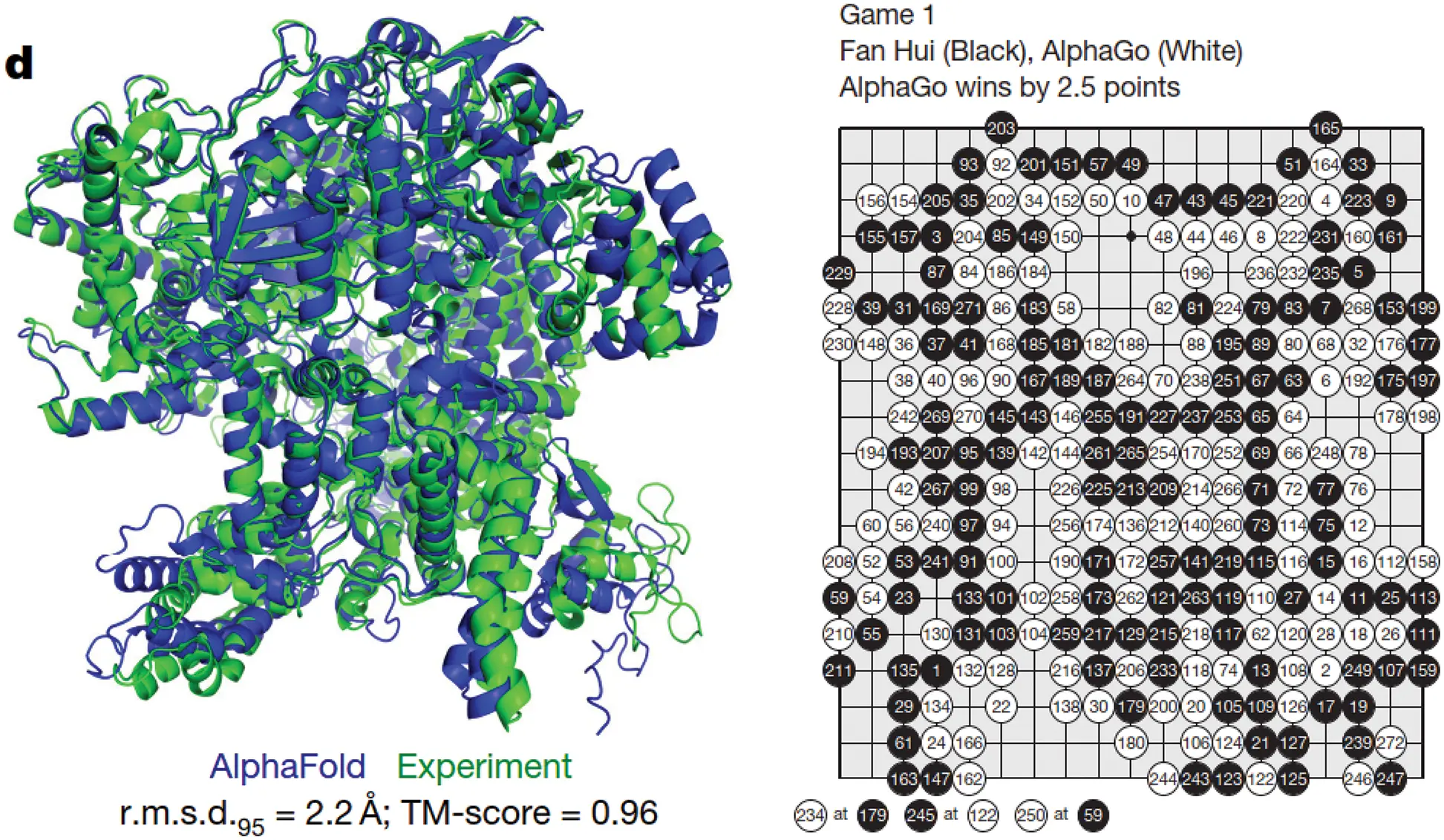Figure 2: Breakthroughs in AI (Section 2). Left: The journal Science 2021 Breakthough of the Year. Protein folded 3-D shape produced by the AI software AlphaFold compared to experiment with high accuracy . The AlphaFold Protein Structure Database contains more than 200 million protein structure predictions, a holy grail sought after in the last 50 years. Right: The AI solfware AlphaGo, a runner-up in the journal Science 2016 Breakthough of the Year, beat the European Go champion Fan Hui five games to zero in 2015 , and then went on to defeat the world Go grandmaster Lee Sedol in 2016 . (Permission by Nature.)

Deep-learning software libraries. Just as there is a large number of available software in different subfields of computational mechanics, there are many excellent deep-learning libraries ready for use in applications; see Section 9, in which some examples of the use of these libraries in engineering applications are provided with the associated computer code. Similar to learning finite-element formulations versus learning how to run finite-element codes, our focus here is to discuss various algorithmic aspects of deep-learning and their applications in computational mechanics, rather than how to use deep-learning libraries in applications. We agree with the view that “a solid understanding of the core principles of neural networks and deep learning” would provide “insights that will still be relevant years from now” , and that would not be obtained from just learning to run some hot libraries.

Readers already familiar with neural networks may find the presentation refreshing,6 and even find new information on neural networks, depending how they used deep learning, or when they stopped working in this area due to the waning wave of connectionism and the new wave of deep learning.7 If not, readers can skip these sections to go directly to the sections on applications of deep learning to computational mechanics.

Applications of deep learning in computational mechanics. We select some recent papers on application of deep learning to computational mechanics to review in details in a way that readers would also understand the computational mechanics contents well enough without having to read through the original papers:

•   Fully-connected feedforward neural networks were employed to make element-matrix integration more efficient, while retaining the accuracy of the traditional Gauss-Legendre quadrature ;8

•   Recurrent neural network (RNN) with Long Short-Term Memory (LSTM) units9 was applied to multiple-scale, multi-physics problems in solid mechanics ;

•   RNNs with LSTM units were employed to obtain reduced-order model for turbulence in fluids based on the proper orthogonal decomposition (POD), a classic linear project method also known as principal components analysis (PCA) . More recent nonlinear-manifold model-order reduction methods, incorporating encoder / decoder and hyper-reduction of dimentionality using gappy (incomplete) data, were introduced, e.g.,  .

Organization of contents. Our review of each of the above papers is divided into two parts. The first part is to summarize the main results and to identify the concepts of deep learning used in the paper, expected to be new for first-time learners, for subsequent elaboration. The second part is to explain in details how these deep-learning concepts were used to produce the results.

The results of deep-learning numerical integration  are presented in Section 2.3.1, where the deep-learning concepts employed are identified and listed, whereas the details of the formulation in  are discussed in Section 10. Similarly, the results and additional deep-learning concepts used in a multi-scale, multi-physics problem of geomechanics  are presented in Section 2.3.2, whereas the details of this formulation are discussed in Section 11. Finally, the results and additional deep-learning concepts used in turbulent fluid simulation with proper orthogonal decomposition  are presented in Section 2.3.3, whereas the details of this formulation, together with the nonlinear-manifold model-order reduction  , are discussed in Section 12.

All of the deep-learning concepts identified from the above selected papers for in-depth are subsequently explained in detail in Sections 3 to 7, and then more in Section 13 on “Historical perspective”.

The parallelism between computational mechanics, neuroscience, and deep learning is summarized in Section 3, which would put computational-mechanics first-time learners at ease, before delving into the details of deep-learning concepts.

Both time-independent (static) and time-dependent (dynamic) problems are discussed. The architecture of (static, time-independent) feedforward multilayer neural networks in Section 4 is expounded in detail, with first-time learners in mind, without assuming prior knowledge, and where experts may find a refreshing presentation and even new information.

Backpropagation, explained in Section 5, is an important method to compute the gradient of the cost function relative to the network parameters for use as a descent direction to decrease the cost function for network training.

For training networks—i.e., finding optimal parameters that yield low training error and lowest validation error—both classic deterministic optimization methods (using full batch) and stochastic optimization methods (using minibatches) are reviewed in detail, and at times even derived, in Section 6, which would be useful for both first-time learners and experts alike.

The examples used in training a network form the training set, which is complemented by the validation set (to determine when to stop the optimization iterations) and the test set (to see whether the resulting network could work on examples never seen before); see Section 6.1.

Deterministic gradient descent with classical line search methods, such as Armijo’s rule (Section 6.2), were generalized to add stochasticity. Detailed pseudocodes for these methods are provided. The classic stochastic gradient descent (SGD) by Robbins & Monro (1951)  (Section 6.3, Section 6.3.1), with add-on tricks such as momentum Polyak (1964)  and fast (accelerated) gradient by Nesterov (1983 , 2018 ) (Section 6.3.2), step-length decay (Section 6.3.4), cyclic annealing (Section 6.3.4), minibatch-size increase (Section 6.3.5), weight decay (Section 6.3.6) are presented, often with detailed derivations.

Step-length decay is shown to be equivalent to simulated annealing using stochastic differential equation equivalent to the discrete parameter update. A consequence is to increase the minibatch size, instead of decaying the step length (Section 6.3.5). In particular, we obtain a new result for minibatch-size increase.

In Section 6.5, highly popular adaptive step-length (learning-rate) methods are discussed in a unified manner in Section 6.5.1, followed by the first paper on AdaGrad  (Section 6.5.2).

Overlooked in (or unknown to) other review papers and even well-known books on deep learning, exponential smoothing of time series originating from the field of forecasting dated since the 1950s, the key technique of adaptive methods, is carefully explained in Section 6.5.3.

The first adaptive methods that employed exponential smoothing were RMSProp  (Section 6.5.4) and AdaDelta  (Section 6.5.5), both introduced at about the same time, followed by the “immensely successful” Adam (Section 6.5.6) and its variants (Sections 6.5.7 and 6.5.8).

Particular attention is then given to a recent criticism of adaptive methods in , revealing their marginal value for generalization, compared to the good old SGD with effective initial step-length tuning and step-length decay (Section 6.5.9). The results were confirmed in three recent independent papers, among which is the recent AdamW adaptive method in  (Section 6.5.10).

Dynamics, sequential data, and sequence modeling are the subjects of Section 7. Discrete time-dependent problems, as a sequence of data, can be modeled with recurrent neural networks discussed in Section 7.1, using the 1997 classic architecture such as Long Short-Term Memory (LSTM) in Section 7.2, but also the recent 2017-18 architectures such as transformer introduced in  (Section 7.4.3), based on the concept of attention . Continuous recurrent neural networks originally developed in neuroscience to model the brain and the connection to their discrete counterparts in deep learning are also discussed in detail,  and Section 13.2.2 on “Dynamic, time dependence, Volterra series”.

The features of several popular, open-source deep-learning frameworks and libraries—such as TensorFlow, Keras, PyTorch, etc.—are summarized in Section 9.

As mentioned above, detailed formulations of deep learning applied to computational mechanics in      are reviewed in Sections 10, 11, 12.

History of AI, limitations, danger, and the classics. Finally, a broader historical perspective of deep learning, machine learning, and artificial intelligence is discussed in Section 13, ending with comments on the geopolitics, limitations, and (identified-and-proven, not just speculated) danger of artificial intelligence in Section 14.

A rare feature is in a detailed review of some important classics to connect to the relevant concepts in modern literature, sometimes revealing misunderstanding in recent works, likely due to a lack of verification of the assertions made with the corresponding classics. For example, the first artificial neural network, conceived by Rosenblatt (1957) , (1962) , had 1000 neurons, but was reported as having a single neuron (Figure 42). Going beyond probabilistic analysis, Rosenblatt even built the Mark I computer to implement his 1000-neuron network (Figure 133, Sections 13.2 and 13.2.1). Another example is the “heavy ball” method, for which everyone referred to Polyak (1964) , but who more precisely called the “small heavy sphere” method (Remark 6.6). Others were quick to dismiss classical deterministic line-search methods that have been generalized to add stochasticity for network training (Remark 6.4). Unintended misrepresentation of the classics would mislead first-time learners, and unfortunately even seasoned researchers who used second-hand information from others, without checking the original classics themselves.

The use of Volterra series to model the nonlinear behavior of neuron in term of input and output firing rates, leading to continuous recurrent neural networks is examined in detail. The linear term of the Volterra series is a convolution integral that provides a theoretical foundation for the use of linear combination of inputs to a neuron, with weights and biases ; see Section 13.2.2.

The experiments in the 1950s by Furshpan et al.   that revealed the rectified linear behavior in neuronal axon, modeled as a circuit with a diode, together with the use of the rectified linear activation function in neural networks in neuroscience years before being adopted for use in deep learning network, are reviewed in Section 13.3.2.

Reference hypertext links and Internet archive. For the convenience of the readers, whenever we refer to an online article, we provide both the link to original website, and if possible, also the link to its archived version in the Internet Archive. For example, we included in the bibliography entry of Ref.  the links to both the Original website and the Internet archive.10

2  Deep Learning, resurgence of Artificial Intelligence

In Dec 2021, the journal Science named, as its “2021 Breakthrough of the Year,” the development of the AI software AlphaFold and its amazing feat of predicting a large number of protein structures . “For nearly 50 years, scientists have struggled to solve one of nature’s most perplexing challenges—predicting the complex 3D shape a string of amino acids will twist and fold into as it becomes a fully functional protein. This year, scientists have shown that artificial intelligence (AI)-driven software can achieve this long-standing goal and predict accurate protein structures by the thousands and at a fraction of the time and cost involved with previous methods” .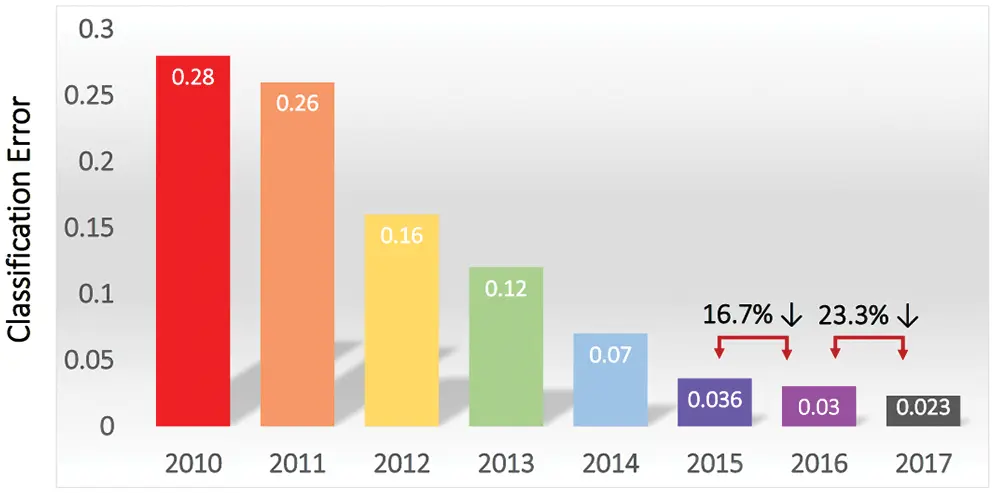Figure 3: ImageNet competitions (Section 2). Top (smallest) classification error rate versus competition year. A sharp decrease in error rate in 2012 sparked a resurgence in AI interest and research . By 2015, the top classification error rate surpassed human classification error rate of 5.1% with Parametric Rectified Linear Unit ; see Section 5.3.3 and also . Figure from . (Figure reproduced with permission of the authors.)

The 3-D shape of a protein, obtained by folding a linear chain of amino acid, determines how this protein would interact with other molecules, and thus establishes its biological functions . There are some 200 million proteins, the building blocks of life, in all living creatures, and 400,000 in the human body . The AlphaFold Protein Structure Database already contained “over 200 million protein structure predictions.”11 For comparison, there were only about 190 thousand protein structures obtained through experiments as of 2022.07.28 . “Some of AlphaFold’s predictions were on par with very good experimental models [Figure 2, left], and potentially precise enough to detail atomic features useful for drug design, such as the active site of an enzyme” . The influence of this software and its developers “would be epochal.”

On the 2019 new-year day, The Guardian  reported the most recent breakthrough in AI, published less than a month before on 2018 Dec 07 in the journal Science in  on the development of the software AlphaZero, based on deep reinforcement learning (a combination of deep learning and reinforcement learning), that can teach itself through self-play, and then “convincingly defeated a world champion program in the games of chess, shogi (Japanese chess), as well as Go”; see Figure 2, right.

Go is the most complex game that mankind ever created, with more combinations of possible moves than chess, and thus the number of atoms in the observable universe.12 It is “the most challenging of classic games for artificial intelligence [AI] owing to its enormous search space and the difficulty of evaluating board positions and moves” .

This breakthrough is the crowning achievement in a string of astounding successes of deep learning (and reinforcenent learning) in taking on this difficult challenge for AI.13 The success of this recent breakthrough prompted an AI expert to declare close the multidecade long, arduous chapter of AI research to conquer immensely-complex games such as chess, shogi, and Go, and to suggest AI researchers to consider a new generation of games to provide the next set of challenges .

In its long history, AI research went through several cycles of ups and downs, in and out of fashion, as described in , ‘Why artificial intelligence is enjoying a renaissance’ (see also Section 13 on historical perspective):

“THE TERM “artificial intelligence” has been associated with hubris and disappointment since its earliest days. It was coined in a research proposal from 1956, which imagined that significant progress could be made in getting machines to “solve kinds of problems now reserved for humans if a carefully selected group of scientists work on it together for a summer”. That proved to be rather optimistic, to say the least, and despite occasional bursts of progress and enthusiasm in the decades that followed, AI research became notorious for promising much more than it could deliver. Researchers mostly ended up avoiding the term altogether, preferring to talk instead about “expert systems” or “neural networks”. But in the past couple of years there has been a dramatic turnaround. Suddenly AI systems are achieving impressive results in a range of tasks, and people are once again using the term without embarrassment.”

The recent resurgence of enthusiasm for AI research and applications dated only since 2012 with a spectacular success of almost halving the error rate in image classification in the ImageNet competition,14 Going from 26% down to 16%; Figure 3 . In 2015, deep-learning error rate of 3.6% was smaller than human-level error rate of 5.1%,15 and then decreased by more than half to 2.3% by 2017.

The 2012 success16 of a deep-learning application, which brought renewed interest in AI research out of its recurrent doldrums known as “AI winters”,17 is due to the following reasons:

•   Availability of much larger datasets for training deep neural networks (find optimized parameters). It is possible to say that without ImageNet, there would be no spectacular success in 2012, and thus no resurgence of AI. Once the importance of having large datasets to develop versatile, working deep networks was realized, many more large datasets have been developed. See, e.g., .

•   Emergence of more powerful computers than in the 1990s, e.g., the graphical processing unit (or GPU), “which packs thousands of relatively simple processing cores on a single chip” for use to process and display complex imagery, and to provide fast actions in today’s video games” .

•   Advanced software infrastructure (libraries) that facilitates faster development of deep-learning applications, e.g., TensorFlow, PyTorch, Keras, MXNet, etc. , p. 25. See Section 9 on some reviews and rankings of deep-learning libraries.

•   Larger neural networks and better training techniques (i.e., optimizing network parameters) that were not available in the 1980s. Today’s much larger networks, which can solve once intractatable / difficult problems, are “one of the most important trends in the history of deep learning”, but are still much smaller than the nervous system of a frog , p. 21; see also Section 4.6. A 2006 breakthrough, ushering in the dawn of a new wave of AI research and interest, has allowed for efficient training of deeper neural networks , p. 18.18 The training of large-scale deep neural networks, which frequently involve highly nonlinear and non-convex optimization problems with many local minima, owes its success to the use of stochastic-gradient descent method first introduced in the 1950s .

•   Successful applications to difficult, complex problems that help people in their every-day lives, e.g., image recognition, speech translation, etc.

In medicine, AI “is beginning to meet (and sometimes exceed) assessments by doctors in various clinical situations. A.I. can now diagnose skin cancer like dermatologists, seizures like neurologists, and diabetic retinopathy like ophthalmologists. Algorithms are being developed to predict which patients will get diarrhea or end up in the ICU,19 and the FDA20 recently approved the first machine learning algorithm to measure how much blood flows through the heart—a tedious, time-consuming calculation traditionally done by cardiologists.” Doctors lamented that they spent “a decade in medical training learning the art of diagnosis and treatment,” and were now easily surpassed by computers . “The use of artificial intelligence is proliferating in American health care—outpacing the development of government regulation. From diagnosing patients to policing drug theft in hospitals, AI has crept into nearly every facet of the health-care system, eclipsing the use of machine intelligence in other industries” .

In micro-lending, AI has helped the Chinese company SmartFinance reduce the default rates of more than 2 millions loans per month to low single digits, a track record that makes traditional brick-and-mortar banks extremely jealous” .

In the popular TED talk “How AI can save humanity” , the speaker alluded to the above-mentioned 2006 breakthrough (, p. 18) that marked the beginning of the “deep learning” wave of AI research when he said:21 “About 10 years ago, the grand AI discovery was made by three North American scientists,22 and it’s known as deep learning”.

Section 13 provices a historical perspective on the development of AI, with additional details on current and future applications.

It was, however, disappointing that despite the above-mentioned exciting outcomes of AI, during the Covid-19 pandemic beginning in 2020,23 none of the hundreds of AI systems developed for Covid-19 diagnosis were usable for clinical applications; see Section 13.5.1. As of June 2022, the Tesla electric vehicle autopilot system is under increased scrutiny by the National Highway Traffic Safety Administration as there were “16 crashes into emergency vehicles and trucks with warning signs, causing 15 injuries and one death.”24 In addition, there are many limitations and danger in the current state-of-the-art of AI; see Section 14.

2.1 Handwritten equation to LaTeX code, image recognition

An image-recognition software useful for computational mechanicists is Mathpix Snip,25 which recognizes hand-written math equations, and transforms them into LaTex codes. For example, Mathpix Snip transforms the hand-written equation below by an 11-year old pupil:Figure 4: Handwritten equation 1 (Section 2.1)

into this LaTeX code “p \times q = m \Rightarrow p = \frac { m } { q }” to yield the equation image:

p×q=mp=mq(1)

Another example is the hand-written multiplication work below by the same pupil: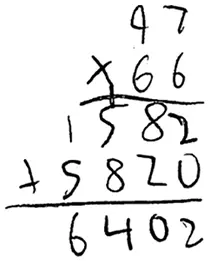Figure 5: Handwritten equation 2 (Section 2.1). Hand-written multiplication work of an eleven-year old pupil.

that Mathpix Snip transformed into the equation image below:26

97×661582+58206402(2)

2.2 Artificial intelligence, machine learning, deep learning

We want to immediately clarify the meaning of the terminologies “Artificial Intelligence” (AI), “Machine Learning” (ML), and “Deep Learning” (DL), since their casual use could be confusing for first-time learners.

For example, it was stated in a review of primarily two computer-science topics called “Neural Networks” (NNs) and “Support Vector Machines” (SVMs) and a physics topic that :27

“The respective underlying fields of basic research—quantum information versus machine learning (ML) and artificial intelligence (AI)—have their own specific questions and challenges, which have hitherto been investigated largely independently.”

Questions would immediately arise in the mind of first-time learners: Are ML and AI two different fields, or the same fields with different names? If one field is a subset of the other, then would it be more general to just refer to the larger set? On the other hand, would it be more specific to just refer to the subset?

In fact, Deep Learning is a subset of methods inside a larger set of methods known as Machine Learning, which in itself is a subset of methods generally known as Artificial Intelligence. In other words, Deep Learning is Machine Learning, which is Artificial Intelligence; , p. 9.28 On the other hand, Artificial Intelligence is not necessarily Machine Learning, which in itself is not necessarily Deep Learning.

The review in  was restricted to Neural Networks (which could be deep or shallow)29 and Support Vector Machine (which is Machine Learning, but not Deep Learning); see Figure 6. Deep Learning can be thought of as multiple levels of composition, going from simpler (less abstract) concepts (or representations) to more complex (abstract) concepts (or representations).30

Based on the above relationship between AI, ML, and DL, it would be much clearer if the phrase “machine learning (ML) and artificial intelligence (AI)” in both the title of  and the original sentence quoted above is replaced by the phrase “machine learning (ML)” to be more specific, since the authors mainly reviewed Multi-Layer Neural (MLN) networks (deep learning, and thus machine learning) and Support Vector Machine (machine learning).31 MultiLayer Neural (MLN) network is also known as MultiLayer Perceptron (MLP).32 both MLN networks and SVMs are considered as artificial intelligence, which in itself is too broad and thus not specific enough.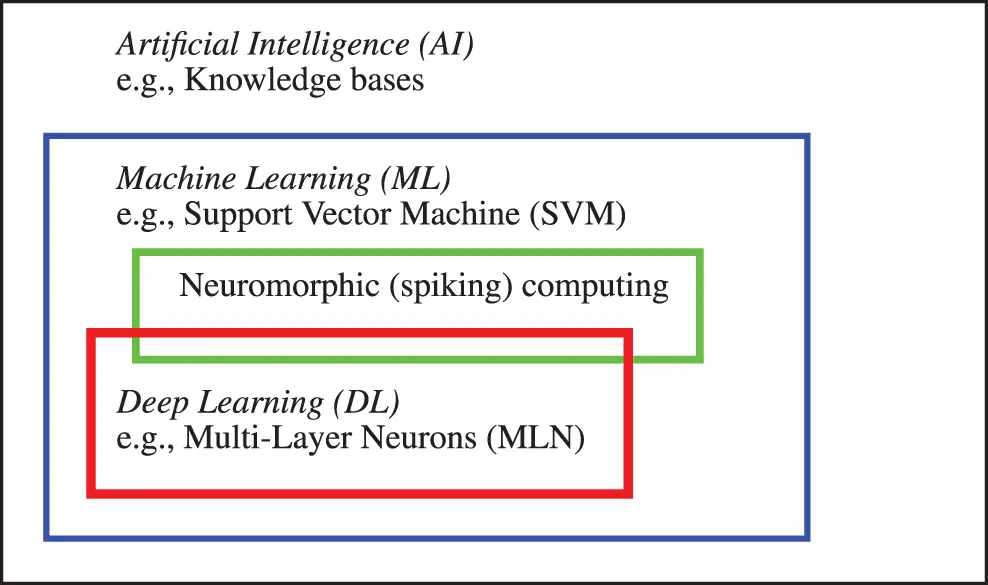Figure 6: Artificial intelligence and subfields (Section 2.2). Three classes of methods—Artificial Intelligence (AI), Machine Learning (ML), and Deep Learning (DL)—and their relationship, with an example of method in each class. A knowledge-base method is an AI method, but is neither a ML method, nor a DL method. Support Vector Machine and spiking computing are ML methods, and thus AI methods, but not a DL method. Multi-Layer Neural (MLN) network is a DL method, and is thus both an ML method and an AI method. See also Figure 158 in Appendix 4 on Cybernetics, which encompassed all of the above three classes.

Another reason for simplifying the title in  is that the authors did not consider using any other AI methods, except for two specific ML methods, even though they discussed AI in the general historical context.

The engine of neuromorphic computing, also known as spiking computing, is a hardware network built into the IBM TrueNorth chip, which contains “1 million programmable spiking neurons and 256 million configurable synapses”,33 and consumes “extremely low power” . Despite the apparent difference with the software approach of deep computing, neuromorphic chip could implement deep-learning networks, and thus the difference was not fundamental . There is thus an overlap between neuromorphic computing and deep learning, as shown in Figure 6, instead of two disconnected subfields of machine learning as reported in .34

2.3 Motivation, applications to mechanics

As motivation, we present in this section the results in three recent papers in computational mechanics, mentioned in the Opening Remarks in Section 1, and identify some deep-learning fundamental concepts (in italics) employed in these papers, together with the corresponding sections in the present paper where these concepts are explained in detail. First-time learners of deep learning likely find these fundamental concepts described by obscure technical jargon, whose meaning will be explained in details in the identified subsequent sections. Experts of deep learning would understand how deep learning is applied to computational mechanics.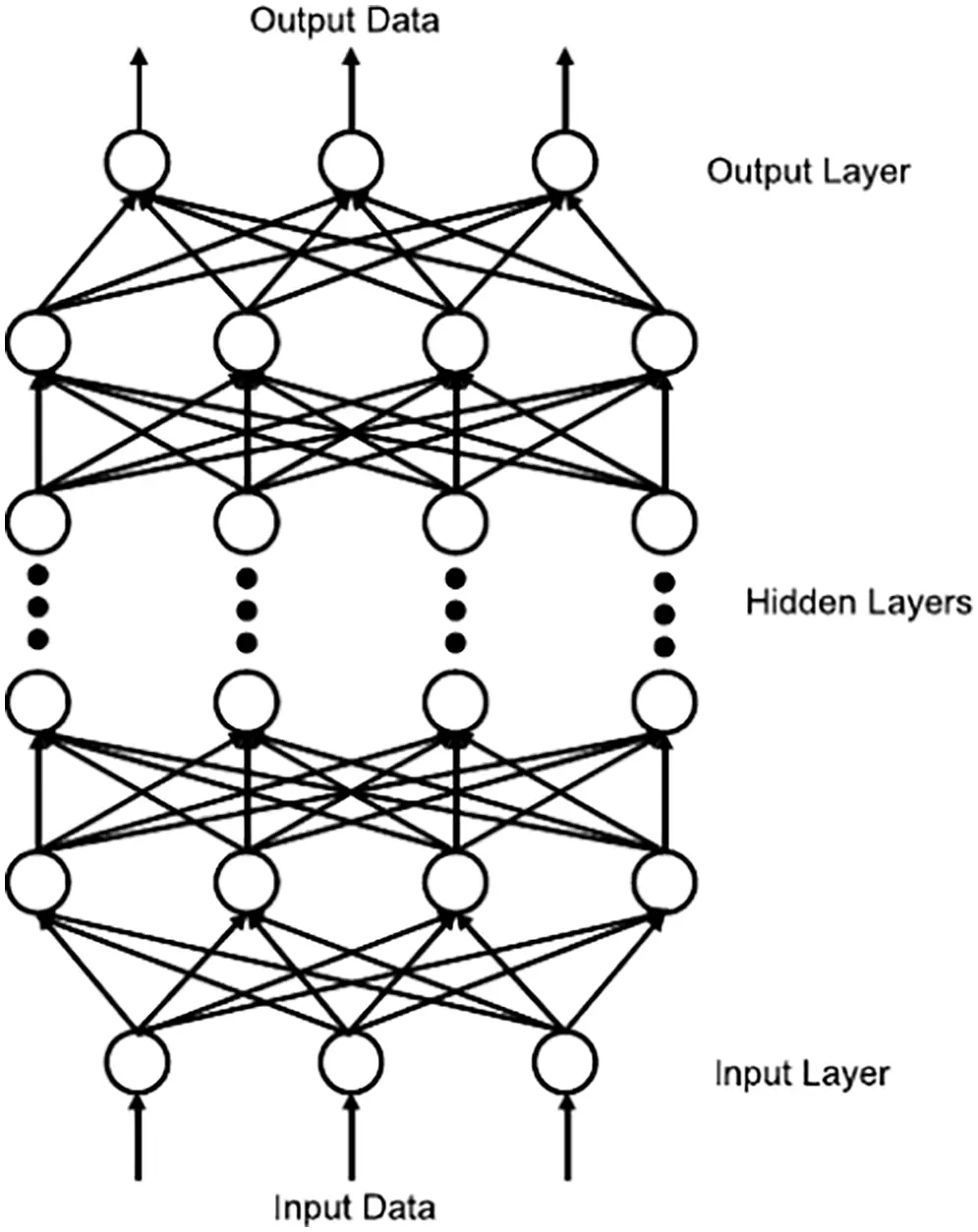Figure 7: Feedforward neural network (Section 2.3.1). A feedforward neural network in , rotated clockwise by 90 degrees to compare to its equivalent in Figure 23 and Figure 35 further below. All terminologies and fundamental concepts will be explained in detail in subsequent sections as listed. See Section 4.1.1 for a top-down explanation and Section 4.1.2 for bottom-up explanation. This figure of a network could be confusing to first-time learners, as already indicated in Footnote 5. (Figure reproduced with permission of the authors.)

2.3.1 Enhanced numerical quadrature for finite elements

To integrate efficiently and accurately the element matrices in a general finite element mesh of 3-D hexahedral elements (including distorted elements), the power of Deep Learning was harnessed in two applications of feedforward MultiLayer Neural networks (MLN,35 Figures 7-8, Section 4) :

(1)  Application 1.1: For each element (particularly distorted elements), find the number of integration points that provides accurate integration within a given error tolerance. Section 10.2 contains the details.

(2)  Application 1.2: Uniformly use 2×2×2 integration points for all elements, distorted or not, and find the appropriate quadrature weights36 (different from the traditional quadrature weights of the Gauss-Legendre method) at these integration points. Section 10.3 contains the details.

To train37 the networks—i.e., to optimize the network parameters (weights and biases, Figure 8) to minimize some loss (cost, error) function (Sections 5.1, 6)—up to 20000 randomly distorted hexahedrals were generated by displacing nodes from a regularly shaped element , see Figure 9. For each distorted shape, the following are determined: (1) the minimum number of integration points required to reach a prescribed accuracy, and (2) corrections to the quadrature weights by trying one million randomly generated sets of correction factors, among which the best one was retained.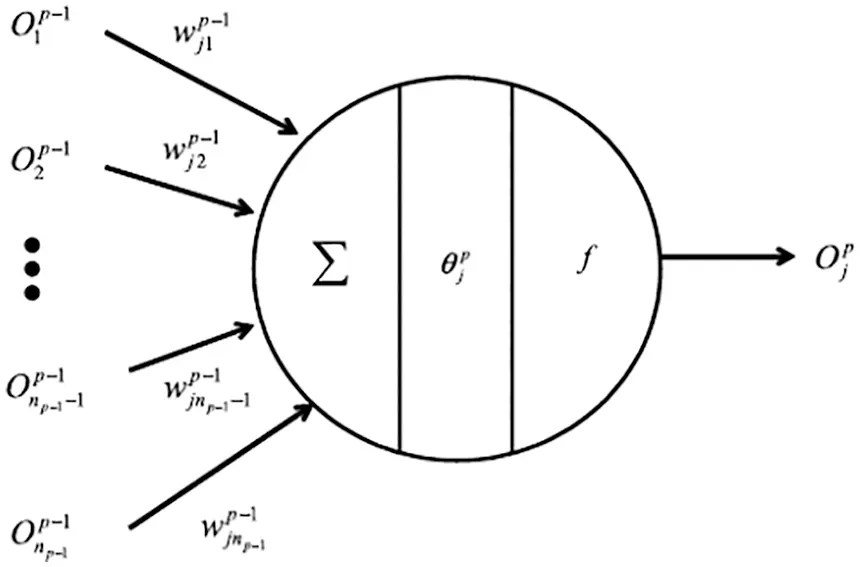Figure 8: Artificial neuron (Section 2.3.1). A neuron with its multiple inputs Oip1 (which are outputs from the previous layer (p1), and thus the variable name “O”), processing operations (multiply inputs with network weights wjip1, sum weighted inputs, add bias θjp, activation function f), and single output Ojp . See the equivalent Figure 36, Section 4.4.3 further below. All terminologies and fundamental concepts will be explained in detail in subsequent sections as listed. See Section 4 on feedforward networks, Section 4.1.1 on top-down explanation and Section 4.1.2 on bottom-up explanation. This figure of a neuron could be confusing to first-time learners, as already indicated in Footnote 5. (Figure reproduced with permission of the authors.)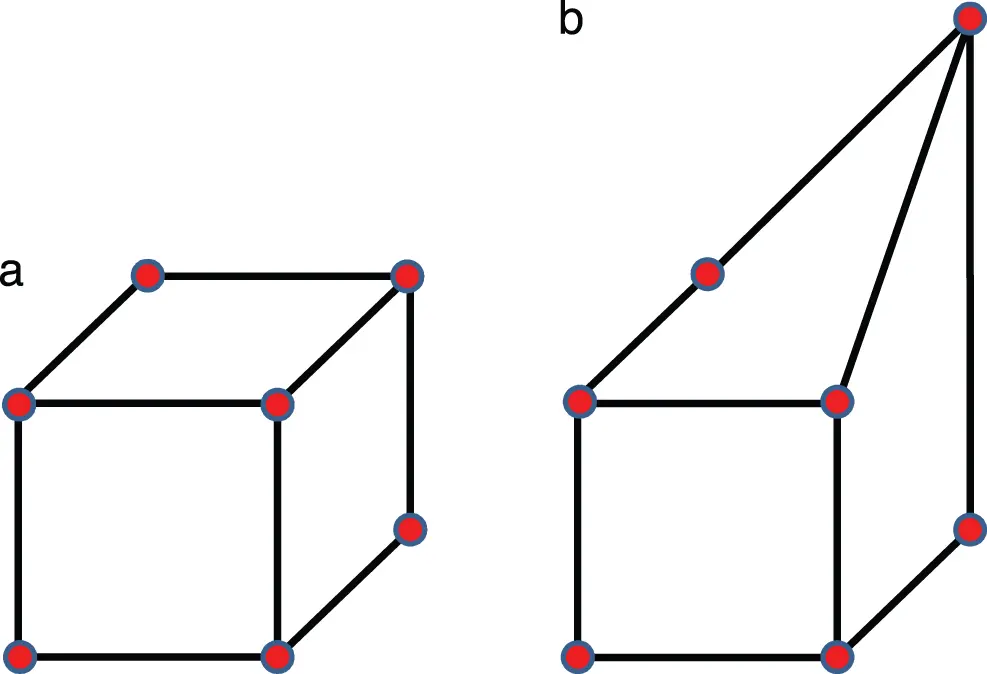Figure 9: Cube and distorted cube elements (Section 2.3.1). Regular and distorted linear hexahedral elements . (Figure reproduced with permission of the authors.)

While Application 1.1 used one fully-connected (Section 4.6.1) feedforward neural network (Section 4), Application 1.2 relied on two neural networks: The first neural network was a classifier that took the element shape (18 normalized nodal coordinates) as input and estimated whether or not the numerical integration (quadrature) could be improved by adjusting the quadrature weights for the given element (one output), i.e., the network classifier only produced two outcomes, yes or no. If an error reduction was possible, a second neural network performed regression to predict the corrected quadrature weights (eight outputs for 2×2×2 quadrature) from the input element shape (usually distorted).

To train the classifier network, 10,000 element shapes were selected from the prepared dataset of 20,000 hexahedrals, which were divided into a training set and a validation set (Section 6.1) of 5000 elements each.38

To train the second regression network, 10,000 element shapes were selected for which quadrature could be improved by adjusting the quadrature weights .

Again, the training set and the test set comprised 5000 elements each. The parameters of the neural networks (weights, biases, Figure 8, Section 4.4) were optimized (trained) using a gradient descent method (Section 6) that minimizes a loss function (Section 5.1), whose gradients with respect to the parameters are computed using backpropagation (Section 5).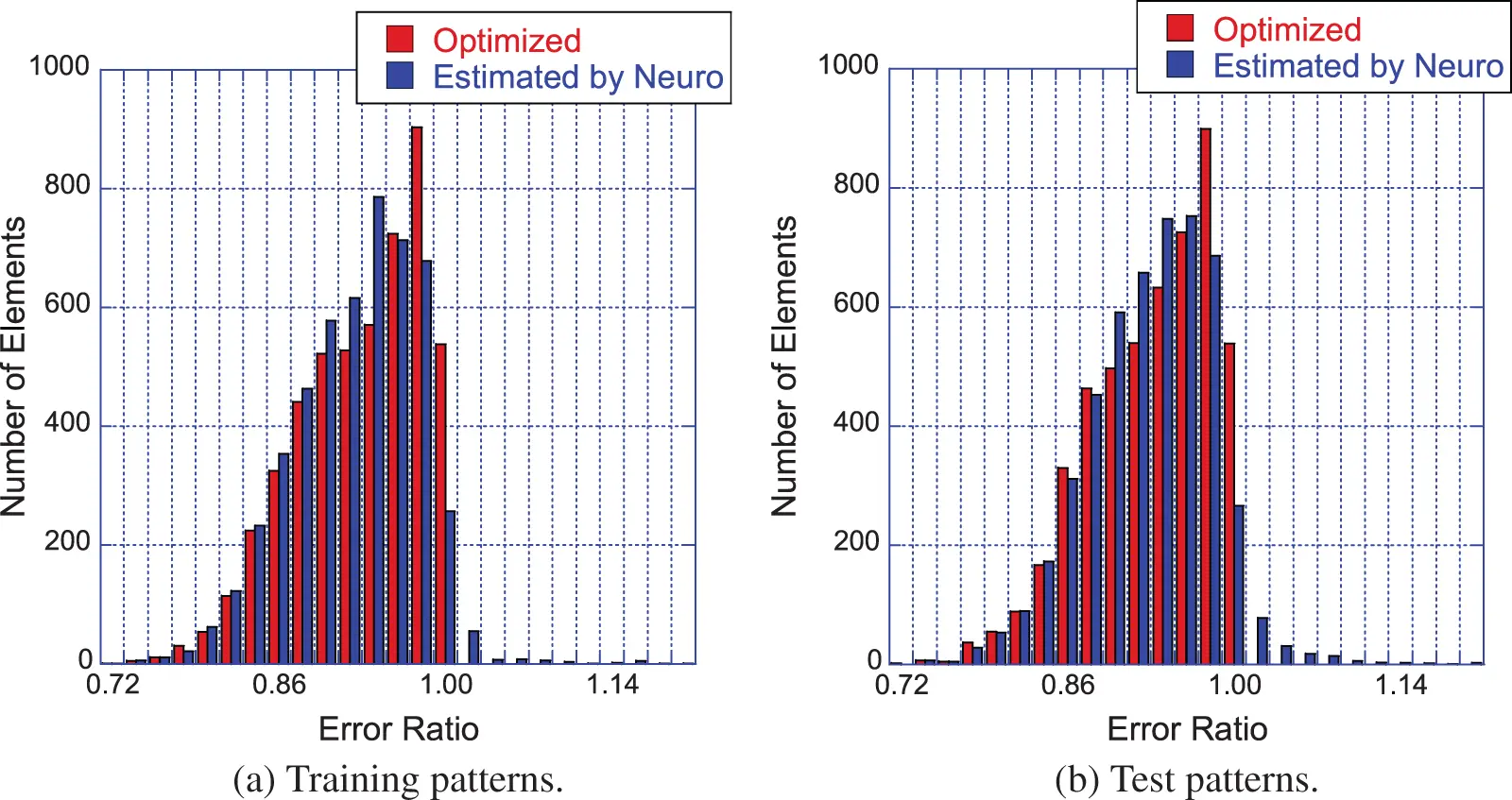Figure 10: Effectiveness of quadrature weight prediction (Section 2.3.1). Subfigure (a): Distribution of error-reduction ratio Rerror for the 5,000 elements in the training set. The red bars (“Optimized”) are the error ratios obtained from the optimal weights (found by a large number of trials-and-errors) that were used to train the network. The blue bars (“Estimated by Neuro”) are the error ratios obtained from the trained neural network. Rerror<1 indicates improved quadrature accuracy. As a result of using the optimal weights, there were no red bars with Rerror>1. That there were very few blue bars with Rerror>1 showed that the proposed method worked in reducing the integration error in more than 97% of the elements. Subfigure (b): Error ratios for the test set with 5000 elements . More detailed explanation is provided in Section 10.3.3. (Figure reproduced with permission of the authors.)

The best results were obtained from a classifier with four hidden layers (Figure 7, Section 4.3, Remark 4.2) with 30 neurons (Figure 8, Figure 36, Section 4.4.3) each and a regression network that had a depth of five hidden layers, where each layer was 50 neurons wide, Figure 7. The results were obtained using the logistic sigmoid function (Figure 30) as activation function (Section 4.4.2) due to existing software, even though the rectified linear function (Figure 24) were more efficient, but yielded comparable accuracy on a few test cases.39

For most element shapes of both the training set (a) and the test set (b), each of which comprised 5000 elements, the blue bars in Figure 10 indicate an error ratio below one, i.e., the quadrature weight correction effectively improved the accuracy of numerical quadrature.

Readers familiar with Deep Learning and neural networks can go directly to Section 10, where the details of the formulations in  are presented. Other sections are also of interest such as classic and state-of-the-art optimization methods in Section 6, attention and transformer unit in Section 7, historical perspective in Section 13, limitations and danger of AI in Section 14.

Readers not familiar with Deep Learning and neural networks will find below a list of the concepts that will be explained in subsequent sections. To facilitate the reading, we also provide the section number (and the link to jump to) for each concept.

Deep-learning concepts to explain and explore:

(1)   Feedforward neural network (Figure 7): Figure 23 and Figure 35, Section 4

(2)   Neuron (Figure 8): Figure 36 in Section 4.4.4 (artificial neuron), and Figure 131 in Section 13.1 (biological neuron)

(3)   Inputs, output, hidden layers, Section 4.3

(4)   Network depth and width: Section 4.3

(5)   Parameters, weights, biases 4.4.1

(6)   Activation functions: Section 4.4.2

(7)   What is “deep” in “deep networks” ? Size, architecture, Section 4.6.1, Section 4.6.2

(8)   Backpropagation, computation of gradient: Section 5

(9)   Loss (cost, error) function, Section 5.1

(10)   Training, optimization, stochastic gradient descent: Section 6

(11)   Training error, validation error, test (or generalization) error: Section 6.1

This list is continued further below in Section 2.3.2. Details of the formulation in  are discussed in Section 10.Figure 11: Dual-porosity single-permeability medium (Section 2.3.2). Left: Actual reservoir. Dual (or double) porosity indicates the presence of two types of porosity in naturally-fractured reservoirs (e.g., of oil): (1) Primary porosity in the matrix (e.g., voids in sands) with low permeability, within which fluid does not flow, (2) Secondary porosity due to fractures and vugs (cavities in rocks) with high (anisotropic) permeability, within which fluid flows. Fluid exchange is permitted between the matrix and the fractures, but not between the matrix blocks (sugar cubes), of which the permeability is much smaller than in the fractures. Right: Model reservoir, idealization. The primary porosity is an array of cubes of homogeneous, isotropic material. The secondary porosity is an “orthogonal system of continuous, uniform fractures”, oriented along the principal axes of anisotropic permeability . (Figure reproduced with permission of the publisher SPE.)

2.3.2 Solid mechanics, multiscale modeling

One way that deep learning can be used in solid mechanics is to model complex, nonlinear constitutive behavior of materials. In single physics, balance of linear momentum and strain-displacement relation are considered as definitions or “universal principles”, leaving the constitutive law, or stress-strain relation, to a large number of models that have limitations, no matter how advanced . Deep learning can help model complex constitutive behaviors in ways that traditional phenomenological models could not; see Figure 105.

Deep recurrent neural networks (RNNs) (Section 7.1) was used as a scale-bridging method to efficiently simulate multiscale problems in hydromechanics, specifically plasticity in porous media with dual porosity and dual permeability .40

The dual-porosity single-permeability (DPSP) model was first introduced for use in oil-reservoir simulation , Figure 11, where the fracture system was the main flow path for the fluid (e.g., two phase oil-water mixture, one-phase oil-solvent mixture). Fluid exchange is permitted between the rock matrix and the fracture system, but not between the matrix blocks. In the DPSP model, the fracture system and the rock matrix, each has its own porosity, with values not differing from each other by a large factor. On the contrary, the permeability of the fracture system is much larger than that in the rock matrix, and thus the system is considered as having only a single permeability. When the permeability of the fracture system and that of the rock matrix do not differ by a large factor, then both permeabilities are included in the more general dual-porosity dual-permeability (DPDP) model .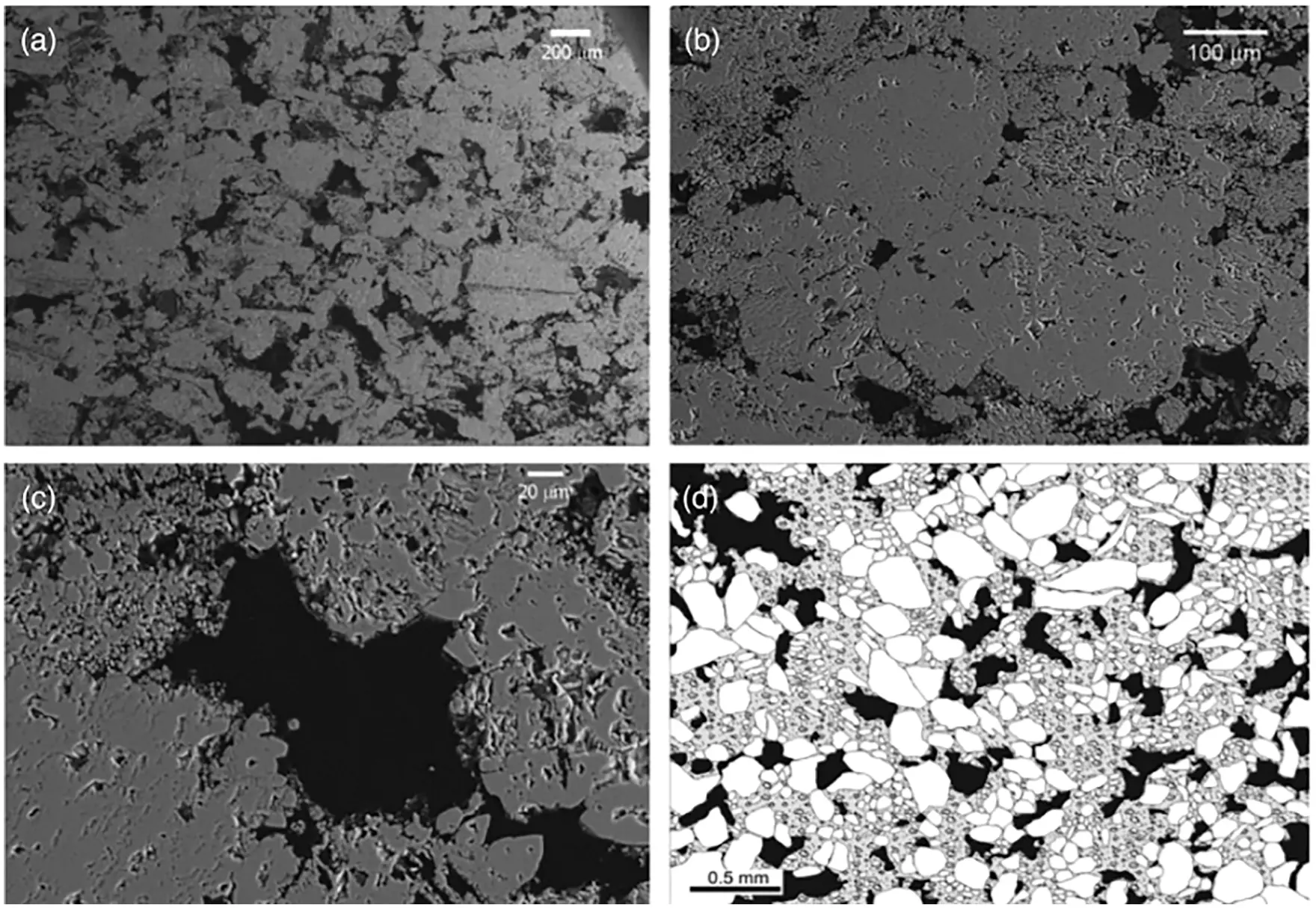Figure 12: Pore structure of Majella limestone, dual porosity (Section 2.3.2), a carbonate rock with high total porisity at 30%. Backscattered SEM images of Majella limestone: (a)-(c) sequence of zoomed-ins; (d) zoomed-out. (a) The larger macropores (dark areas) have dimensions comparable to the grains (allochems), having an average diameter of 54 μm, with macroporosity at 11.4%. (b) Micropores embedded in the grains and cemented regions, with microporosity at 19.6%, which is equal to the total porosity at 30% minus the macroporosity. (c) Numerous micropores in the periphery of a macropore. (d) Map performed manually under optical microscope showing the partitioning of grains, matrix (mostly cement) and porosity . See Section 11 and Remark 11.8. (Figure reproduced with permission of the authors.)

Since 60% of the world’s oil reserve and 40% of the world’s gas reserve are held in carbonate rocks, there has been a clear interest in developing an understanding of the mechanical behavior of carbonate rocks such as limestones, having from lowest porosity (Solenhofen at 3%) to high porosity (e.g., Majella at 30%). Chalk (Lixhe) is a carbonate rock with highest porosity at 42.8%. Carbonate rock reservoirs are also considered to store carbon dioxide and nuclear waste  .

In oil-reservoir simulations in which the primary interest is the flow of oil, water, and solvent, the porosity (and pore size) within each domain (rock matrix or fracture system) is treated as constant and homogeneous  .41 On the other hand, under mechanical stress, the pore size would change, cracks and other defects would close, leading to a change in the porosity in carbonate rocks. Indeed, “at small stresses, experimental mechanical deformation of carbonate rock is usually characterized by a non-linear stress-strain relationship, interpreted to be related to the closure of cracks, pores, and other defects. The non-linear stress-strain relationship can be related to the amount of cracks and various type of pores” , p. 202. Once the pores and cracks are closed, the stress-strain relation becomes linear, at different stress stages, depending on the initial porosity and the geometry of the pore space .Figure 13: Majella limestone, nonlinear stress-strain relations (Section 2.3.2). Differential stress (i.e., the difference between the largest principal stress and the smallest one) vs axial strain (left) and vs volumetric strain (right) . See Remark 11.7, Section 11.3.4, and Remark 11.10, Section 11.3.5. (Figure reproduced with permission of the authors.)

Moreover, pores have different sizes, and can be classified into different pore sub-systems. For the Majella limestone in Figure 12 with total porosity at 30%, its pore space can be partitioned into two subsystems (and thus dual porosity), the macropores with macroporosity at 11.4% and the micropores with microporosity at 19.6%. Thus the meaning of dual-porosity as used in  is different from that in oil-reservoir simulation. Also characteristic of porous rocks such as the Majella limestone is the non-linear stress-strain relation observed in experiments, Figure 13, due the changing size, and collapse, of the pores.

Likewise, the meaning of “dual permeability” is different in  in the sense that “one does not seek to obtain a single effective permeability for the entire pore space”. Even though it was not explicitly spelled out,42 it appears that each of the two pore sub-systems would have its own permeability, and that fluid is allowed to exchange between the two pore sub-systems, similar to the fluid exchange between the rock matrix and the fracture system in the DPSP and DPDP models in oil-reservoir simulation .

In the problem investigated in , the presence of localized discontinuities demands three scales—microscale (μ), mesoscale (cm), macroscale (km)—to be considered in the modeling, see Figure 14. Classical approaches to consistently integrate microstructural properties into macroscopic constitutive laws relied on hierarchical simulation models and homogenization methods (e.g., discrete element method (DEM)–FEM coupling, FEM2). If more than two scales were to be considered, the computational complexity would become prohibitively, if not intractably, large.

Instead of coupling multiple simulation models online, two (adjacent) scales were linked by a neural network that was trained offline using data generated by simulations on the smaller scale . The trained network subsequently served as a surrogate model in online simulations on the larger scale. With three scales being considered, two recurrent neural networks (RNNs) with Long Short-Term Memory (LSTM) units were employed consecutively:

(1)   Mesoscale RNN with LSTM units: On the microscopic scale, a representative volume element (RVE) was an assembly of discrete-element particles, subjected to large variety of representative loading paths to generate training data for the supervised learning of the mesoscale RNN with LSTM units, a neural network that was referred to as “Mesoscale data-driven constitutive model”  (Figure 14). Homogenizing the results of DEM-flow model provided constitutive equations for the traction-separation law and the evolution of anisotropic permeabilities in damaged regions.

(2)   Macroscale RNN with LSTM units: The mesoscale RVE (middle row in Figure 14), in turn, was a finite-element model of a porous material with embedded strong discontinuities equivalent to the fracture system in oil-reservoir simulation in Figure 11. The host matrix of the RVE was represented by an isotropic linearly elastic solid. In localized fracture zones within, the traction-separation law and the hydraulic response were provided by the mesoscale RNN with LSTM units developed above. Training data for the macroscale RNN with LSTM units—a network referred to as “Macroscale data-driven constitutive model” —is generated by computing the (homogenized) response of the mesoscale RVE to various loadings. In macroscopic simulations, the mesoscale RNN with LSTM units provided the constitutive response at a sealing fault that represented a strong discontinuity.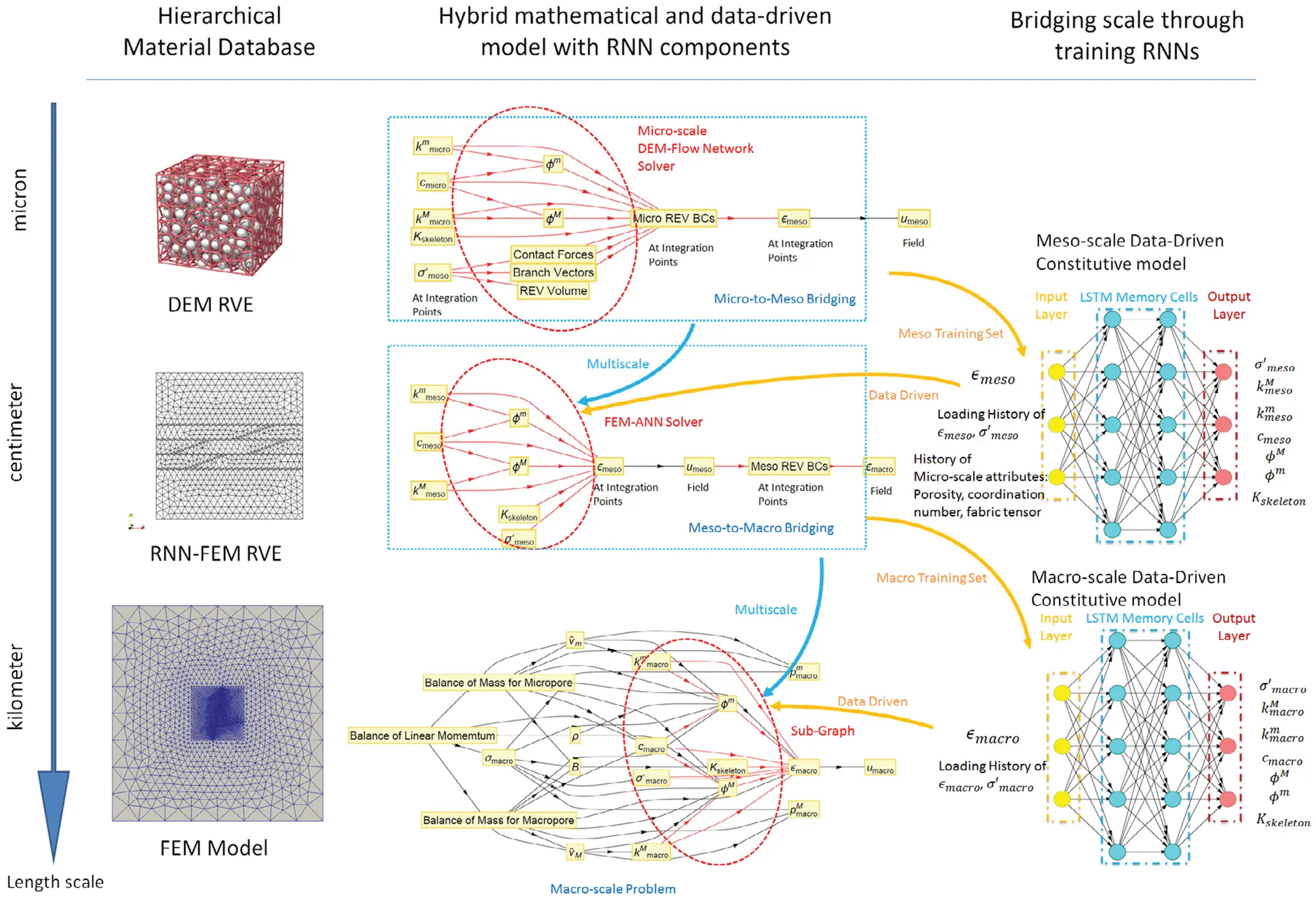Figure 14: Hierarchy of a multi-scale multi-physics poromechanics problem for fluid-infiltrating media  (Sections 2.3.2, 11.1, 11.3.1, 11.3.3, 11.3.5). Microscale (μ), mesoscale (cm), macroscale (km). DEM RVE = Discrete Element Method Representative Volume Element. RNN-FEM = Recurrent Neural Network (Section 7.1) - Finite Element Method. LSTM = Long Short-Term Memory (Section 7.2). The mesoscale has embedded strong discontinuities equivalent to the fracture system in Figure 11. See Figure 104, where the orientations of the RVEs are shown, Figure 106 for the microscale RVE (Remark 11.1) and Figure 113 for the mesoscale RVE (Remark 11.9). (Figure reproduced with permission of the authors.)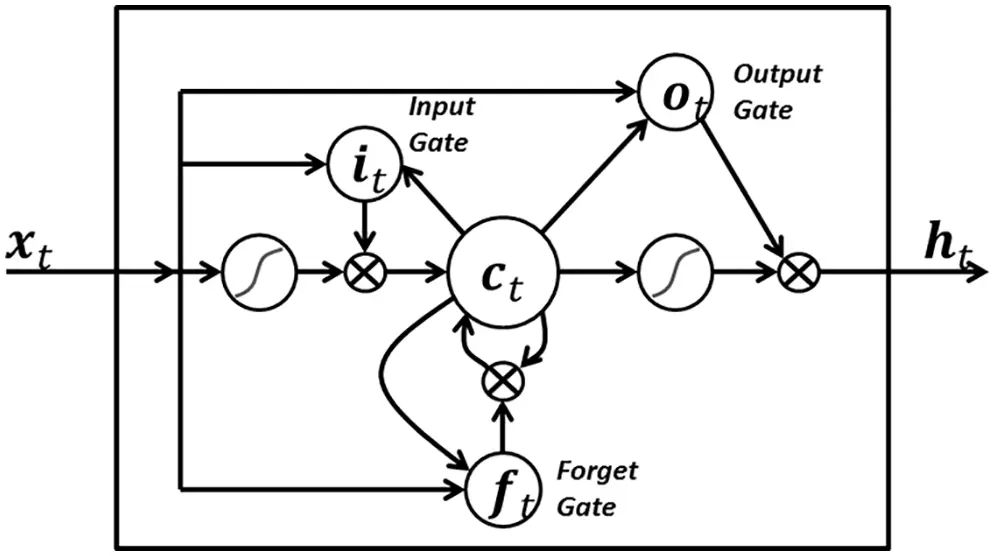Figure 15: LSTM variant with “peephole” connections, block diagram (Sections 2.3.2, 7.2).43 Unlike the original LSTM unit (see Section 7.2), both the input gate and the forget gate in an LSTM unit with peephole connections receive the cell state as input. The above figure from Wikipedia, version 22:56, 4 October 2015, is identical to Figure 10 in , whose authors erroneously used this figure without mentioning the source, but where the original LSTM unit without “peepholes” was actually used, and with the detailed block diagram in Figure 81, Section 7.2. See also Figure 82 and Figure 117 for the original LSTM unit applied to fluid mechanics. (CC-BY-SA 4.0)

Path-dependence is a common characteristic feature of the constitutive models that are often realized as neural networks; see, e.g., . For this reason, it was decided to employ RNN with LSTM units, which could mimick internal variables and corresponding evolution equations that were intrinsic to path-dependent material behavior . These authors chose to use a neural network that had a depth of two hidden layers with 80 LSTM units per layer, and that had proved to be a good compromise of performance and training efforts. After each hidden layer, a dropout layer with a dropout rate 0.2 were introduced to reduce overfitting on noisy data, but yielded minor effects, as reported in . The output layer was a fully-connected layer with a logistic sigmoid as activation function.

An important observation is that including micro-structural data—the porosity ϕ, the coordination number CN (number of contact points, Figure 16), the fabric tensor (defined based on the normals at the contact points, Eq. (404) in Section 11; Figure 16 provides a visualization)—as network inputs significantly improved the prediction capability of the neural network. Such improvement is not surprising since soil fabric—described by scalars (porosity, coordination number, particle size) and vectors (fabric tensors, particle orientation, branch vectors)—exerts great influence on soil behavior . Coordination number44 has been used to predict soil particle breakage , morphology and crushability , and in a study of internally-unstable soil involving a mixture of coarse and fine particles . Fabric tensors, with theoretical foundation developed in , provide a mean to represent directional data such as normals at contact points, even though other types of directional data have been proposed to develop fabric tensors . To model anisotropic behavior of granular materials, contact-normal fabric tensor was incorporated in an isotropic constitutive law.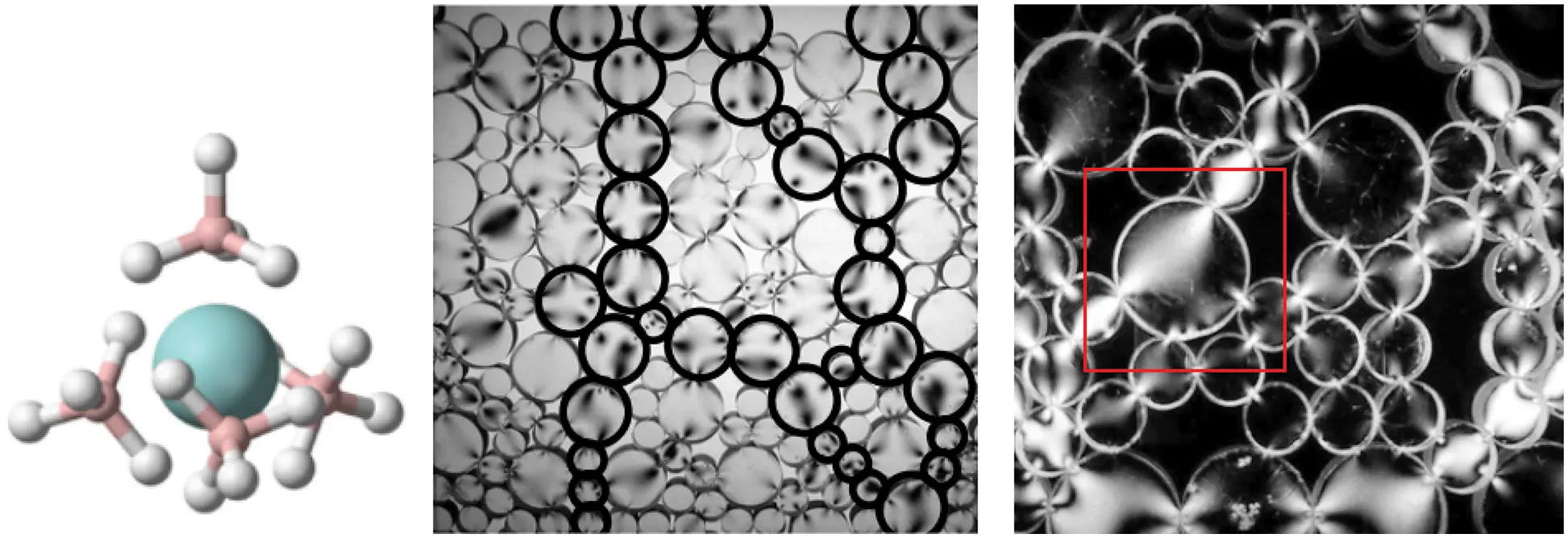Figure 16: Coordination number CN (Section 2.3.2, 11.3.2). (a) Chemistry. Number of bonds to the central atom. Uranium borohydride U(BH4)4 has CN = 12 hydrogen bonds to uranium. (b, c) Photoelastic discs showing number of contact points (coordination number) on a particle. (b) Random packing and force chains, different force directions along principal chains and in secondary particles. (c) Arches around large pores, precarious stability around pores. The coordination number for the large disc (particle) in red square is 5, but only 4 of those had nonzero contact forces based on the bright areas showing stress action. Figures (b, c) also provide a visualization of the “flow” of the contact normals, and thus the fabric tensor . See also Figure 17. (Figure reproduced with permission of the author.)

Figure 17 illustrates the importance of incorporating microstructure data, particularly the fabric tensor, in network training to improve prediction accuracy.

Deep-learning concepts to explain and explore: (continued from above in Section 2.3.1)

(12)   Recurrent neural network (RNN), Section 7.1

(13)   Long Short-Term Memory (LSTM), Section 7.2

(14)   Attention and Transformer, Section 7.4.3

(15)   Dropout layer and dropout rate,45 which had minor effects in the particular work repoorted in , and thus will not be covered here. See , p. 251, Section 7.12.

Details of the formulation in  are discussed in Section 11.

2.3.3 Fluid mechanics, reduced-order model for turbulence

The accurate simulation of turbulence in fluid flows ranks among the most demanding tasks in computational mechanics. Owing to both the spatial and the temporal resolution, transient analysis of turbulence by means of high-fidelity methods such as Large Eddy Simulation (LES) or direct numerical simulation (DNS) involves millions of unknowns even for simple domains.

To simulate complex geometries over larger time periods, reduced-order models (ROMs) that can capture the key features of turbulent flows within a low-dimensional approximation space need to be resorted to. Proper Orthogonal Decomposition (POD) is a common data-driven approach to construct an orthogonal basis {ϕ1(x),ϕ2(x),ϕ(x)} from high-resolution data obtained from high-fidelity models or measurements, in which x is a point in a 3-D fluid domain ; see Section 12.1. A flow dynamic quantity u(x,t), such as a component of the flow velocity field, can be projected on the POD basis by separation of variables as (Figure 18)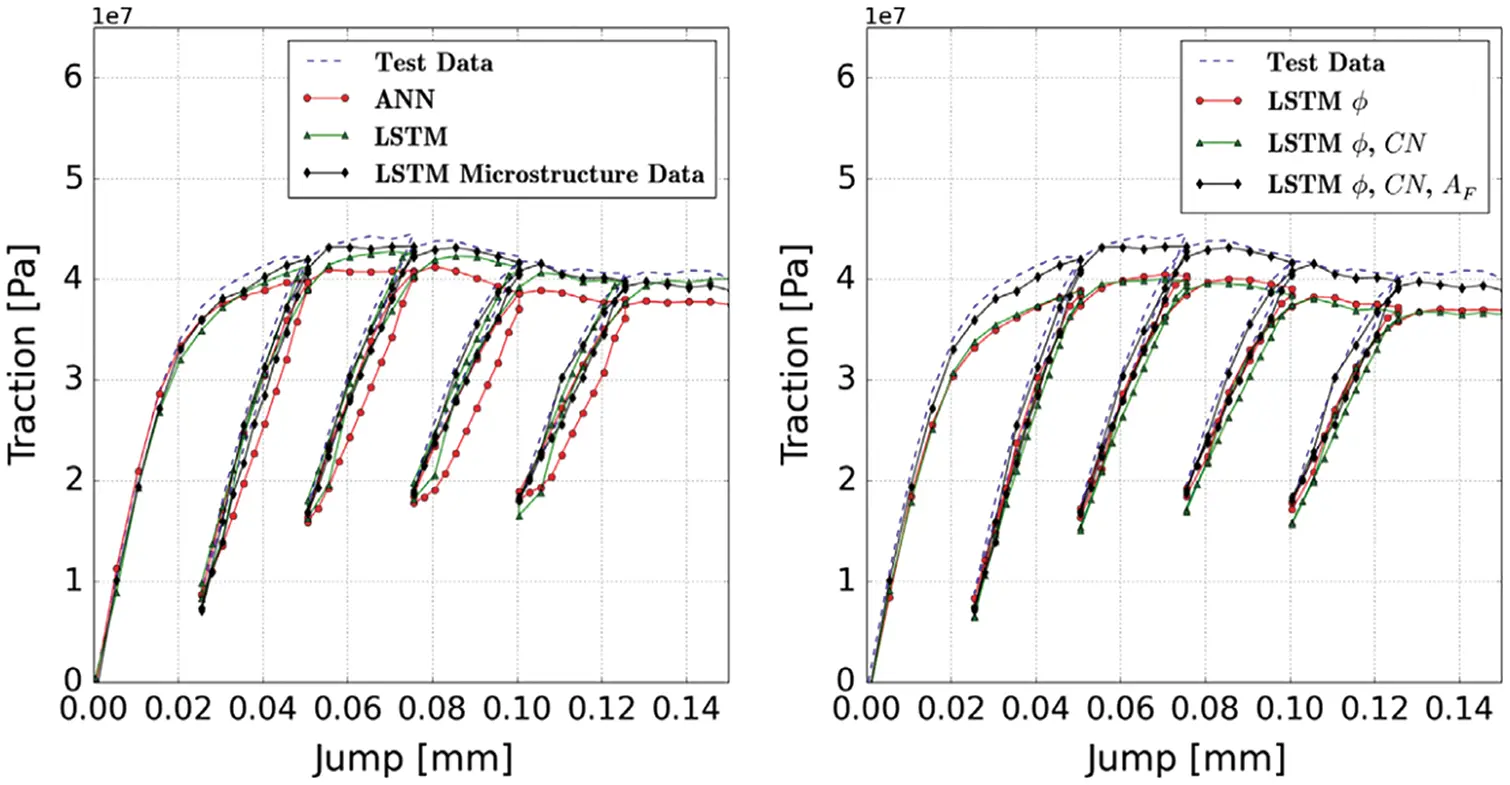Figure 17: Network with LSTM and microstructure data (porosity ϕ, coordination number CN=Nc, Figure 16, fabric tensor F=AFAF, Eq. (404)) (Section 2.3.2, 11.3.2). Simple shear test using Discrete Element Method to provide network training data under loading-unloading conditions. ANN = Artificial Neural Network with no LSTM units. While network with LSTM units and (ϕ, CN, AF) improved the predicted traction, compared to network with LSTM units and only (ϕ) or (ϕ, CN); the latter two networks produced predicted traction that was worse compared to network with LSTM alone, indicating the important role of the fabric tensor, which contained directional data that were absent in scalar fields like (ϕ, CN) . (Figure reproduced with permission of the author.)

u(x,t)=i=1i=ϕi(x)αi(t)i=1i=kϕi(x)αi(t), with k<,(3)

where k is a finite number, which could be large, and αi(t) a time-dependent coefficient for ϕi(x). The computation would be more efficient if a much smaller subset with, say, mk, POD basis functions,

u(x,t)j=1j=mϕij(x)αij(t), with mk and ij{1,,k},(4)

where {ij,j=1,,m} is a subset of indices in the set {1,,k}, and such that the approximation in Eq. (4) is with minimum error compared to the approximation in Eq. (3)2 using the much larger set ofk POD basis functions.

In a Galerkin-Project (GP) approach to reduced-order model, a small subset of dominant modes form a basis onto which high-dimensional differential equations are projected to obtain a set of lower-dimensional differential equations for cost-efficient computational analysis.

Instead of using GP, RNNs (Recurrent Neural Networks) were used in  to predict the evolution of fluid flows, specifically the coefficients of the dominant POD modes, rather than solving differential equations. For this purpose, their LSTM-ROM (Long Short-Term Memory - Reduced Order Model) approach combined concepts of ROM based on POD with deep-learning neural networks using either the original LSTM units, Figure 117 (left) , or the bidirectional LSTM (BiLSTM), Figure 117 (right) , the internal states of which were well-suited for the modeling of dynamical systems.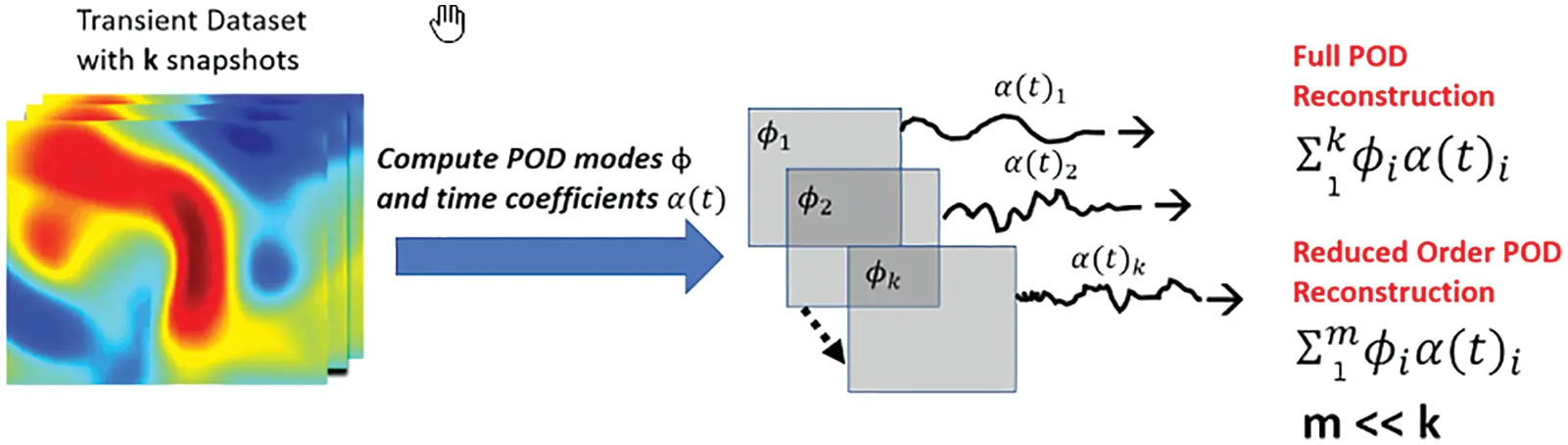Figure 18: Reduced-order POD basis (Sections 2.3.3, 12.1). For each dataset (also Figure 116), which contained k snapshots, the full POD reconstruction of the flow-field dynamical quantity u(x,t), where x is a point in the 3-D flow field, consists of all k basis functions ϕi(x), with i=1,,k, using Eq. (3); see also Eq. (439). Typically, k is large; a reduced-order POD basis consists of selecting mk basis functions for the reconstruction, with the smallest error possible. See Figure 19 for the use of deep-learning networks to predict αi(t+t), with t>0, given αi(t) . (Figure reproduced with permission of the author.)

To obtain training/testing data, which were crucial to train/test neural networks, the data from transient 3-D Direct Navier-Stokes (DNS) simulations of two physical problems, as provided by the Johns Hopkins turbulence database  were used : (1) The Forced Isotropic Turbulence (ISO) and (2) The Magnetohydrodynamic Turbulence (MHD).

To generate training data for LSTM/BiLSTM networks, the 3-D turbulent fluid flow domain of each physical problem was decomposed into five equidistant 2-D planes (slices), with one additional equidistant 2-D plane served to generate testing data (Section 12, Figure 116, Remark 12.1). For the same subregion in each of those 2-D planes, POD was applied on the k snapshots of the velocity field (k=5,023 for ISO, k=1,024 for MHD, Section 12.1), and out of k POD modes {ϕi(x), i=1,,k}, the five (m=5k) most dominant POD modes {ϕi(x), i=1,,m} representative of the flow dynamics (Figure 18) were retained to form a reduced-order basis onto which the velocity field was projected. The coefficient αi(t) of the POD mode ϕi(x) represented the evolution of the participation of that mode in the velocity field, and was decomposed into thousands of small samples using a moving window. The first half of each sample was used as input signal to an LSTM network, whereas the second half of the sample was used as output signal for supervised training of the network. Two different methods were proposed :

(1)   Multiple-network method: Use a RNN for each coefficient of the dominant POD modes

(2)   Single-network method: Use a single RNN for all coefficients of the dominant POD modes

For both methods, variants with the original LSTM units or the BiLSTM units were implemented. Each of the employed RNN had a single hidden layer.

Demonstrative results for the prediction capabilities of both the original LSTM and the BiLSTM networks are illustrated in Figure 20. Contrary to the authors’ expectation, networks with the original LSTM units performed better than those using BiLSTM units in both physical problems of isotropic turbulence (ISO) (Figure 20a) and magnetohydrodyanmics (MHD) (Figure 20b) .

Details of the formulation in  are discussed in Section 12.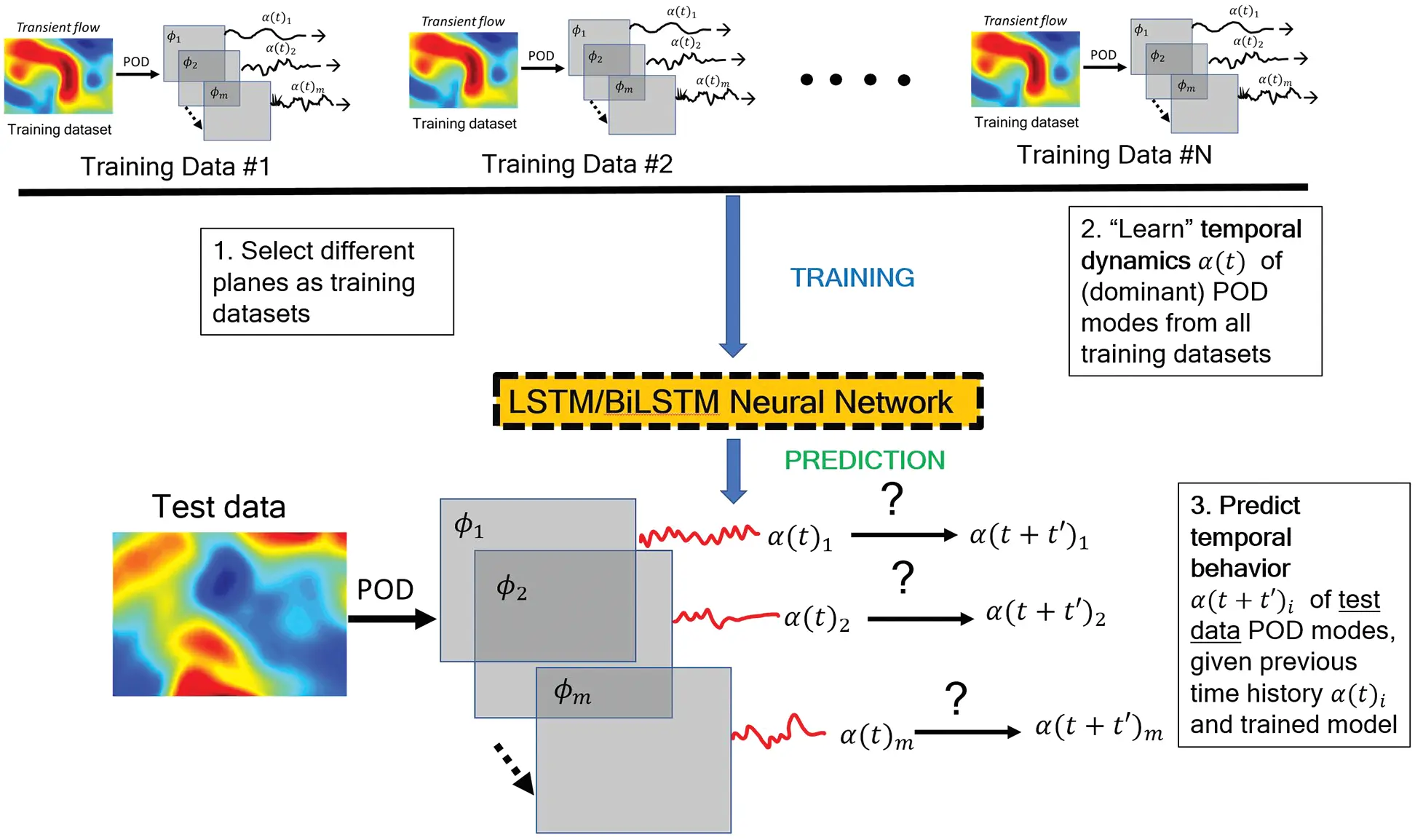Figure 19: Deep-learning LSTM/BiLSTM Reduced Order Model (Sections 2.3.3, 12.2). See Figure 18 for POD reduced-order basis. The time-dependent coefficients αi(t) of the dominant POD modes ϕi, with i=1,,m, from each training dataset were used to train LSTM (or BiLSTM, Figure 117) neural networks to predict αi(t+t), with t>0, given αi(t) of the test datasets. . (Figure reproduced with permission of the author.)

3  Computational mechanics, neuroscience, deep learning

Table 1 below presents a rough comparison that shows the parallelism in the modeling steps in three fields: Computational mechanics, neuroscience, and deep learning, which heavily indebted to neuroscience until it reached more mature state, and then took on its own development.

We assume that readers are familiar with the concepts listed in the second column on “Computational mechanics”, and briefly explain some key concepts in the third column on “Neuroscience” to connect to the fourth column “Deep learning”, which is explained in detail in subsequent sections.

See Section 13.2 for more details on the theoretical foundation based on Volterra series for the spatial and temporal combinations of inputs, weights, and biases, widely used in artificial neural networks or multilayer perceptrons.

Neuron spiking response such as shown in Figure 21 can be modelled accurately using a model such as “Integrate-and-Fire”. The firing-rate response r() of a biological neuron to a stimulus s() is described by a convolution integral46

r(t)=r0+τ=τ=t𝒦(tτ)s(τ)dτ,(5)

where r0 is the background firing rate at zero stimulus, 𝒦() is the synaptic kernel, and s() the stimulus; see, e.g., , p. 46, Eq. (2.1).47. The stimulus s() in Eq. (5) is a train (sequence in time) of spikes described by

s(τ)=iδ(τti)(6)

where δ() is the Dirac delta. Eq. (5) then describes the firing rate r(t) at time t as the collective memory effect of all spikes, going from the current time τ=t back far in the past with τ=, with the weight for the spike s(τ) at time τ provided by the value of the synaptic kernel 𝒦(tτ) at the same time τ.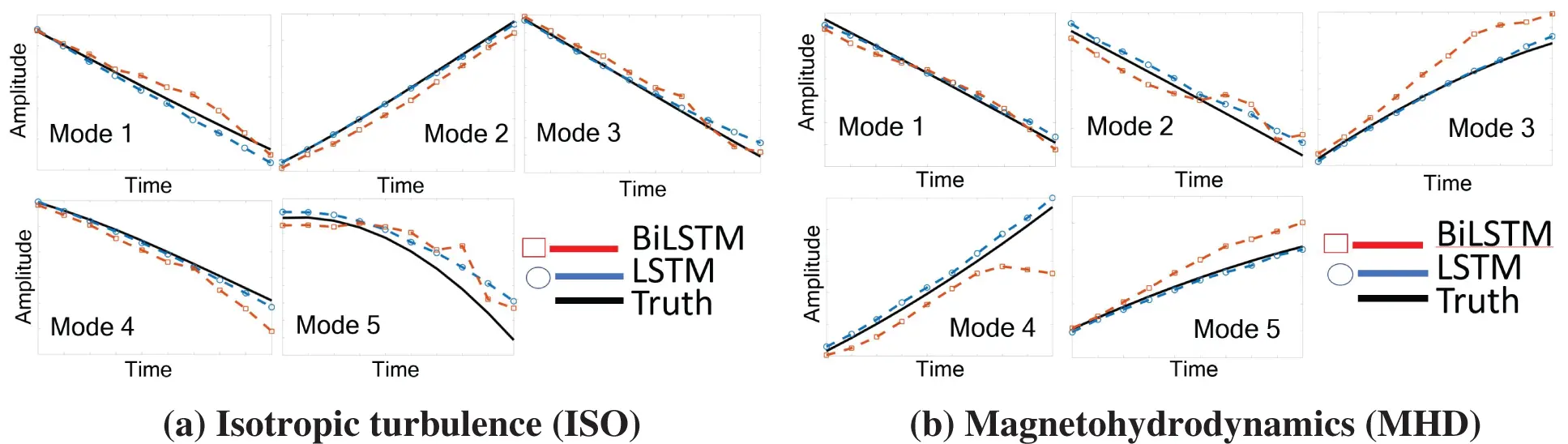Figure 20: Prediction results and errors for LSTM/BiLSTM networks (Sections 2.3.3, 12.3). Coefficients αi(t), for i = 1, . . . , 5, of dominant POD modes. LSTM had smaller errors compared to BiLSTM for both physical problems (ISO and MHD).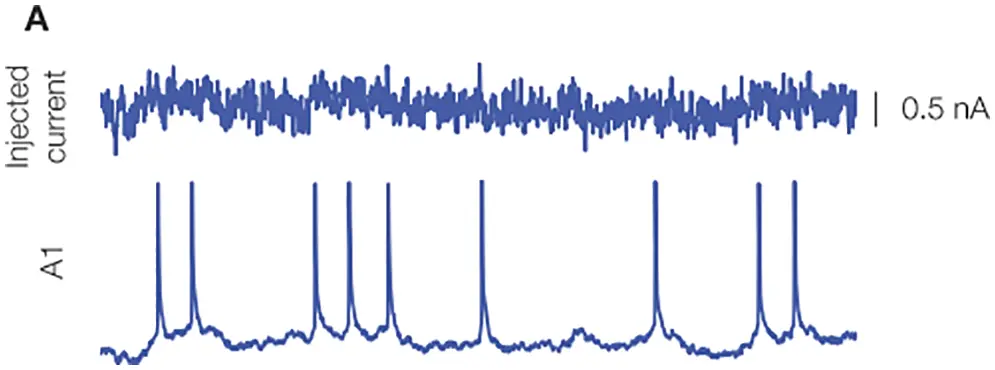Figure 21: Biological neuron response to stimulus, experimental result (Section 3). An oscillating current was injected into the neuron (top), and neuron spiking response was recorded (below) , with permission from the authors and Frontiers Media SA. A spike, also called an action potential, is an electrical potential pulse across the cell membrane that lasts about 100 milivolts over 1 miliseconds. “Neurons represent and transmit information by firing sequences of spikes in various temporal patterns” , pp. 3-4.

It will be seen in Section 13.2.2 on “Dynamics, time dependence, Volterra series” that the convolution integral in Eq. (5) corresponds to the linear part of the Volterra series of nonlinear response of a biological neuron in terms of the stimulus, Eq. (497), which in turn provides the theoretical foundation for taking the linear combination of inputs, weights, and biases for an artificial neuron in a multilayer neural networks, as represented by Eq. (26).

The Integrate-and-Fire model for biological neuron provides a motivation for the use of the rectified linear units (ReLU) as activation function in multilayer neural networks (or perceptrons); see Figure 28.

Eq. (5) is also related to the exponential smoothing technique used in forecasting and applied to stochastic optimization methods to train multilayer neural networks; see Section 6.5.3 on “Forecasting time series, exponential smoothing”.

4  Statics, feedforward networks

We examine in detail the forward propagation in feedforward networks, in which the function mappings flow48 only one forward direction, from input to output.

4.1 Two concept presentations

There are two ways to present the concept of deep-learning neural networks: The top-down approach versus the bottom-up approach.

4.1.1 Top-down approach

The top-down approach starts by giving up-front the mathematical big picture of what a neural network is, with the big-picture (high level) graphical representation, then gradually goes down to the detailed specifics of a processing unit (often referred to as an artificial neuron) and its low-level graphical representation. A definite advantage of this top-down approach is that readers new to the field immediately have the big picture in mind, before going down to the nitty-gritty details, and thus tend not to get lost. An excellent reference for the top-down approach is , and there are not many such references.

Specifically, for a multilayer feedforward network, by top-down, we mean starting from a general description in Eq. (18) and going down to the detailed construct of a neuron through a weighted sum with bias in Eq. (26) and then a nonlinear activation function in Eq. (35).

In terms of block diagrams, we begin our top-down descent from the big picture of the overall multilayer neural network with L layers in Figure 23, through Figure 34 for a typical layer () and Figure 35 for the lower-level details of layer (), then down to the most basic level, a neuron in Figure 36 as one row in layer () in Figure 35, the equivalent figure of Figure 8, which in turn was the starting point in .

4.1.2 Bottom-up approach

The bottom-up approach typically starts with a biological neuron (see Figure 131 in Section 13.1 below), then introduces an artificial neuron that looks similar to the biological neuron (compare Figure 8 to Figure 131), with multiple inputs and a single output, which becomes an input to each of a multitude of other artificial neurons; see, e.g.,    .49 Even though Figure 7, which preceded Figure 8 in , showed a network, but the information content is not the same as Figure 23.

Unfamiliar readers when looking at the graphical representation of an artificial neural network (see, e.g., Figure 7) could be misled in thinking in terms of electrical (or fluid-flow) networks, in which Kirchhoff’s law applies at the junction where the output is split into different directions to go toward other artificial neurons. The big picture is not clear at the outset, and could be confusing to readers new to the field, who would take some time to understand; see also Footnote 5. By contrast, Figure 23 clearly shows a multilevel function composition, assuming that first-time learners are familiar with this basic mathematical concept.

4.2 Matrix notation

In mechanics and physics, tensors are intrinsic geometrical objects, which can be represented by infinitely many matrices of components, depending on the coordinate systems.50 vectors are tensors of order 1. For this reason, we do not use neither the name “vector” for a column matrix, nor the name “tensor” for an array with more than two indices.51 All arrays are matrices.The matrix notation used here can follow either (1) the Matlab / Octave code syntax, or (2) the more compact component convention for tensors in mechanics.

Using Matlab / Octave code syntax, the inputs to a network (to be defined soon) are gathered in an n×1 column matrix x of real numbers, and the (target or labeled) outputs52 in an m×1 column matrix y

x=[x1,,xn]T=[x1;;xn]=[x1xn]Rn×1,y=[y1,,ym]TRm×1(7)

where the commas are separators for matrix elements in a row, and the semicolons are separators for rows. For matrix transpose, we stick to the standard notation using the superscript “T” for written documents, instead of the prime “” as used in matlab / octave code. In addition, the prime “” is more customarily used to denote derivative in handwritten and in typeset equations.

Using the component convention for tensors in mechanics,53 The coefficients of a n×m matrix shown below

[Aij]=[Aji]Rn×m(8)

are arranged according to the following convention for the free indices i and j, which are automatically expanded to their respective full range, i.e., i=1,,n and j=1,m when the variable Aij=Aji are enclosed in square brackets:

(1)  In case both indices are subscripts, then the left subscript (index i of Aij in Eq. (8)) denotes the row index, whereas the right subscript (index j of Aij in Eq. (8)) denotes the column index.

(2)  In case one index is a superscript, and the other index is a subscript, then the superscript (upper index i of Aji in Eq. (8)) is the row index, and the subscript (lower index j of Aji in Eq. (8)) is the column index.54

With this convention (lower index designates column index, while upper index designates row index), the coefficients of array x in Eq. (7) can be presented either in row form (with lower index) or in column form (with upper index) as follows:

[xi]=[x1,x2,,xn]R1×n,[xi]=[x1x2xn]Rn×1, with xi=xi,i=1,,n(9)

Instead of automatically associating any matrix variable such as x to the column matrix of its components, the matrix dimensions are clearly indicated as in Eq. (7) and Eq. (9), i.e., by specifying the values m (number of rows) and n (number of columns) of its containing space Rm×n.

Consider the Jacobian matrix

yx=[yixj]Rm×n and let Aji:=yixj(10)

where y and x are column matrices shown in Eq. (7). Then the coefficients of this Jacobian matrix are arranged with the upper index i being the row index, and the lower index j being the column index.55 This convention is natural when converting a chain rule in component form into matrix form, i.e., consider the composition of matrix functions

z(y(x(θ))), with θRp,x:RpRn,y:RnRm,z:RmRl(11)

where implicitly

RpRp×1,RnRn×1,RmRm×1,RlRl×1(12)

are the spaces of column matrices, Then using the chain rule

ziθj=ziyryrxsxsθj(13)

where the summation convention on the repeated indices r and s is implied. Then the Jacobian matrix zθ can be obtained directly as a product of Jacobian matrices from the chain rule just by putting square brackets around each factor:

z(y(x(θ)))θ=[ziθj]=[ziyr][yrxs][xsθj](14)

Consider the scalar function E:RmR that maps the column matrix yRm into a scalar, then the components of the gradient of E with respect to y are arranged in a row matrix defined as follows:

[Eyj]1×m=[Ey1,,Eym]=yTER1×m(15)

with yTE being the transpose of the m×1 column matrix yE containing these same components.56

Now consider this particular scalar function below:57

Z=wy with wR1×n and yRn×1(16)

Then the gradients of z are58

zwj=yj[zwj]=[yj]=yTR1×n and [zyj]=[wj]=wR1×n(17)

4.3 Big picture, composition of concepts

A fully-connected feedforward network is a chain of successive applications of functions f() with =1,,L, one after another—with L being the number of “layers” or the depth of the network—on the input x to produce the predicted output y˜ for the target output y:

y˜=f(x)=(f(L)f(L1)f()f(2)f(1))(x)=f(L)(f(L1)((f()((f(2)(f(1)(x)))))))(18)

or breaking Eq. (18) down, step by step, from inputs to outputs:59

y(0)=x(1)=x (inputs) y()=f()(x())=x(+1) with =1,,L1y(L)=f(L)(x(L))=y˜ (predicted outputs) (19)

Remark 4.1. The notation y(), for =0,,L in Eq. (19) will be useful to develop a concise formulation for the computation of the gradient of the cost function relative to the parameters by backpropagation for use in training (finding optimal parameters); see Eqs. (91)-(92) in Section 5 on Backpropagation.    ■

The quantities associated with layer () in a network are indicated with the superscript (), so that the inputs to layer () as gathered in the m(1)×1 column matrix

x()=[x1(),,xm(1)()]T=y(1)Rm(1)×1(20)

are the predicted outputs from the previous layer (1), gathered in the matrix y(1), With m(1) being the width of layer (1). Similarly, the outputs of layer () as gathered in the m()×1 matrix

y()=[y1(),,ym()]T=x(+1)Rm()×1(21)

are the inputs to the subsequent layer (+1), gathered in the matrix x(+1), with m() being the width of layer ().

Remark 4.2. The output for layer (), denoted by y(), can also be written as h(), where “h” is mnemonic for “hidden”, since the inner layers between the input layer (1) and the output layer (L) are considered as being “hidden”. Both notations y() and h() are equivalent

y()h()(22)

and can be used interchangeably. In the current Section 4 on “Static, feedforward networks”, the notation y() is used, whereas in Section 7 on “Dynamics, sequential data, sequence modeling”, the notation h[n] is used to designate the output of the “hidden cell” at state [n] in a recurrent neural network, keeping in mind the equivalence in Eq. (276) in Remark 7.1. Whenever necessary, readers are reminded of the equivalence in Eq. (22) to avoid possible confusion when reading deep-learning literature.    ■

The above chain in Eq. (18)—see also Eq. (23) and Figure 23—is referred to as “multiple levels of composition” that characterizes modern deep learning, which no longer attempts to mimic the working of the brain from the neuroscientific perspective.60 Besides, a complete understanding of how the brain functions is still far remote.61

4.3.1 Graphical representation, block diagrams

A function can be graphically represented as in Figure 22.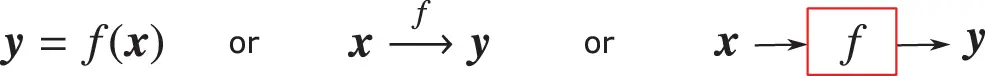Figure 22: Function mapping, graphical representation (Section 4.3.1): n inputs in xRn×1 (n×1 column matrix of real numbers) are fed into function f to produce m outputs in yRm×1.

The multiple levels of compositions in Eq. (18) can then be represented by

x=y(0)Inputf(1)y(1)f(2)y(1)f()y()f(L1)y(L1)f(L)Network as multilevel composition of functionsy(L)=y˜Output(23)

revealing the structure of the feedforward network as a multilevel composition of functions (or chain-based network) in which the output y(1) of the previous layer (1) serves as the input for the current layer (), to be processed by the function f() to produce the output y(). the input x=y(0) for the input layer (1) is the input for the entire network. The output y(L)=y˜ of the (last) layer (L) is the predicted output for the entire network.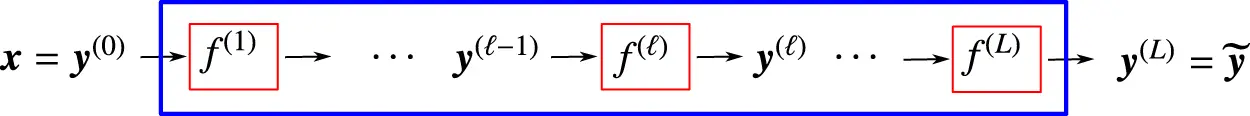Figure 23: Feedforward network (Sections 4.3.1, 4.4.4): Multilevel composition in feedforward network with L layers represented as a sequential application of functions f(), with =1,,L, to n inputs gathered in x=y(0)Rn×1 (n×1 column matrix of real numbers) to produce m outputs gathered in y(L)=y~Rm×1. This figure is a higher-level block diagram that corresponds to the lower-level neural network in Figure 7 or in Figure 35.

Remark 4.3. Layer definitions, action layers, state layers. In Eq. (23) and in Figure 23, an action layer is defined by the action, i.e., the function f(), on the inputs y(1) to produce the outputs y(). There are L action layers. A state layer is a collection of inputs or outputs, i.e., y(),=0,,L, each describes a state of the system, thence the number of state layers is L+1, and the number of hidden (state) layers (excluding the input layer y(0) and the output layer y(L)) is (L1). For an illustration of state layers, see , p. 6, Figure1.2. See also Remark 11.3. From here on, “hidden layer” means “hidden state layer”, agreeing with the terminology in . See also Remark 4.5 on depth definitions in Section 4.6.1 on “Depth, size”.    ■

4.4 Network layer, detailed construct

4.4.1 Linear combination of inputs and biases

First, an affine transformation on the inputs (see Eq. (26)) is carried out, in which the coefficients of the inputs are called the weights, and the constants are called the biases. The output y(1) of layer (1) is the input to layer ()

y(1)=[y1(1),,ym(1)(1)]TRm(1)×1.(24)

The column matrix z()

z()=[z1(),,zm()()]TRm()×1(25)

is a linear combination of the inputs in y(1) plus the biases (i.e., an affine transformation)62

z()=W()y(1)+b() such that zi()=wi()y(1)+bi(), for i=1,,m(),(26)

where the m()×m(1) matrix W contains the weights63

wi()=[wi1(),,wim(1)()]Rm(1)×1,W()=[w1();;wm()()]=[w1()wm()()]Rm()×m(1),(27)

and the m()×1 column matrix b() the biases:64

z()=[z1(),,zm()()]TRm()×1,b()=[b1(),,bm()()]TRm()×1.(28)

Both the weights and the biases are collectively known as the network parameters, defined in the following matrices for layer ():

θi()=[θij()]=[θi1(),,θim(1)() | θi(m(1)+1)()]=[wi1(),,wim(1)() | bi()]=[wi() | bi()]R1×[m(1)+1](29)

Θ()=[θij()]=[θ1()θm()()]=[W() | b()]Rm()×[m(1)+1](30)

For simplicity and convenience, the set of all parameters in the network is denoted by θ, and the set of all parameters in layer () by θ():65

θ={θ(1),,θ(),,θ(L)}={Θ(1),,Θ(),,Θ(L)}, such that θ()Θ()(31)

Note that the set θ in Eq. (31) is not a matrix, but a set of matrices, since the number of rows m() for a layer () may vary for different values of , even though in practice, the widths of the layers in a fully connected feed-forward network may generally be chosen to be the same.

Similar to the definition of the parameter matrix θ() in Eq. (30), which includes the biases b(), it is convenient for use later in elucidating the backpropagation method in Section 5 (and Section 5.2 in particular) to expand the matrix y(1) in Eq. (26) into the matrix y¯(1) (with an overbar) as follows:

z()=W()y(1)+b()[W() | b()][y(1)1]=:θ() y¯(1),(32)

with

θ():=[W() | b()]Rm()×[m(1)+1], and y¯(1):=[y(1)1]R[m(1)+1]×1.(33)

The total number of parameters of a fully-connected feedforward network is then

PT:==1=Lm()×[m(1)+1].(34)

But why using a linear (additive) combination (or superposition) of inputs with weights, plus biases, as expressed in Eq. (26) ? See Section 13.2.

4.4.2 Activation functions

An activation function a:RR, which is a nonlinear real-valued function, is used to decide when the information in its argument is relevant for a neuron to activate. In other words, an activation function filters out information deemed insignificant, and is applied element-wise to the matrix z() in Eq. (26), obtained as a linear combination of the inputs plus the biases:

y()=a(z()) such that yi()=a(zi())(35)

Without the activation function, the neural network is simply a linear regression, and cannot learn and perform complex tasks, such as image classification, language translation, guiding a driver-less car, etc. See Figure 32 for the block diagram of a one-layer network.

An example is a linear one-layer network, without activation function, being unable to represent the seemingly simple XOR (exclusive-or) function, which brought down the first wave of AI (cybernetics), and that is described in Section 4.5.

Rectified linear units (ReLU). Nowadays, for the choice of activation function a(), Most modern large deep-learning networks use the default,66 well-proven rectified linear function (more often known as the “positive part” function) defined as67

a(z)=z+=[z]+=max(0,z)={0 for z0z for 0<z(36)

and depicted in Figure 24, for which the processing unit is called the rectified linear unit (ReLU),68 which was demonstrated to be superior to other activation functions in many problems.69 Therefore, in this section, we discuss in detail the rectified linear function, with careful explanation and motivation. It is important to note that ReLU is superior for large network size, and may have about the same, or less, accuracy than the older logistic sigmoid function for “very small” networks, while requiring less computational efforts.70Figure 24: Activation function (Section 4.4.2): Rectified linear function and its derivatives. See also Section 5.3.3 and Figure 54 for Parametric ReLU that helped surpass human level performance in ImageNet competition for the first time in 2015, Figure 3 . See also Figure 26 for a halfwave rectifier.

To transform an alternative current into a direct current, the first step is to rectify the alternative current by eliminating its negative parts, and thus The meaning of the adjective “rectified” in rectified linear unit (ReLU). Figure 25 shows the current-voltage relation for an ideal diode, for a resistance, which is in series with the diode, and for the resulting ReLU function that rectifies an alternative current as input into the halfwave rectifier circuit in Figure 26, resulting in a halfwave current as output.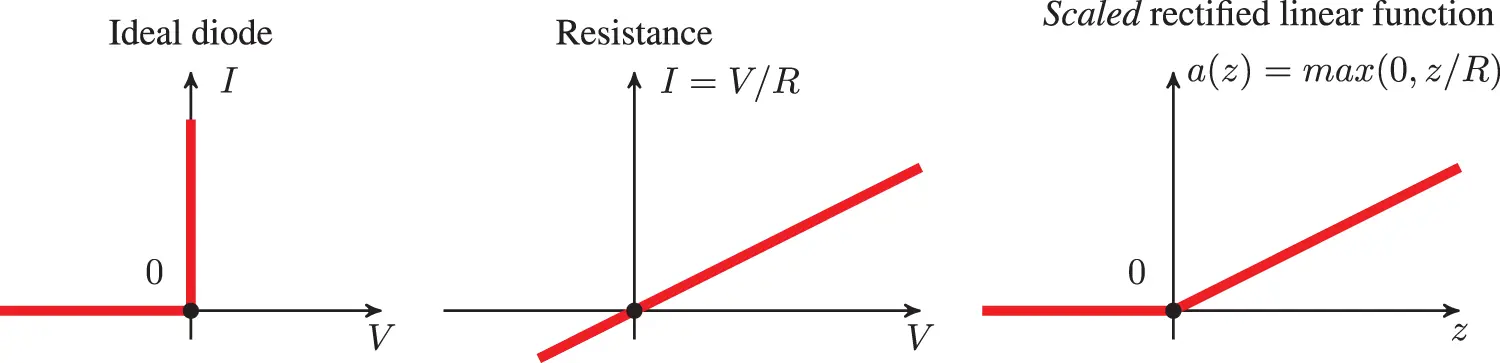Figure 25: Current I versus voltage V (Section 4.4.2): Ideal diode, resistance, scaled rectified linear function as activation (transfer) function for the ideal diode and resistance in series. (Figure plotted with R = 2.) See also Figure 26 for a halfwave rectifier.

Mathematically, a periodic function remains periodic after passing through a (nonlinear) rectifier (active function):

z(x+T)=z(x)y(x+T)=a(z(x+T))=a(z(x))=y(x)(37)

where T in Eq. (37) is the period of the input current z.

Biological neurons encode and transmit information over long distance by generating (firing) electrical pulses called action potentials or spikes with a wide range of frequencies , p. 1; see Figure 27. “To reliably encode a wide range of signals, neurons need to achieve a broad range of firing frequencies and to move smoothly between low and high firing rates” . From the neuroscientific standpoint, the rectified linear function could be motivated as an idealization of the “Type I” relation between the firing rate (F) of a biological neuron and the input current (I), called the FI curve. Figure 27 describes three types of FI curves, with Type I in the middle subfigure, where there is a continuous increase in the firing rate with increase in input current.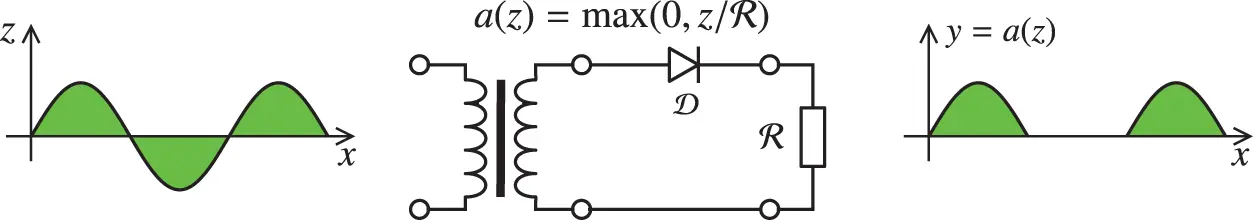Figure 26: Halfwave rectifier circuit (Section 4.4.2), with a primary alternative current z going in as input (left), passing through a transformer to lower the voltage amplitude, with the secondary alternative current out of the transformer being put through a closed circuit with an ideal diode 𝒟 and a resistor in series, resulting in a halfwave output current, which can be grossly approximated by the scaled rectified linear function ya(z)=max(0,z/) (right) as shown in Figure 25, with scaling factor 1/. The rectified linear unit in Figure 24 corresponds to the case with =1. For a more accurate Shockley diode model, The relation between current I and voltage V for this circuit is given in Figure 29. Figure based on source in Wikipedia, version 01:49, 7 January 2015.

The Shockley equation for a current I going through a diode 𝒟, in terms of the voltage VD across the diode, is given in mathematical form as:

I=p[eqVD1]VD=1qlog(Ip+1).(38)

With the voltage across the resistance being VR=RI, the voltage across the diode and the resistance in series is then

V=VD+VR=VD=1qlog(Ip+1)+RI,(39)

which is plotted in Figure 29. The rectified linear function could be seen from Figure 29 as a very rough approximation of the current-voltage relation in a halfwave rectifier circuit in Figure 26, in which a diode and a resistance are in series. In the Shockley model, the diode is leaky in the sense that there is a small amount of current flow when the polarity is reversed, unlike the case of an ideal diode or ReLU (Figure 24), and is better modeled by the Leaky ReLU activation function, in which there is a small positive (instead of just flat zero) slope for negative z:

a(z)=max(0.01z,z)={0.01z for z0z for 0<z(40)

Prior to the introduction of ReLU, which had been long widely used in neuroscience as activation function prior to 2011,71 the state-of-the-art for deep-learning activation function was the hyperbolic tangent (Figure 31), which performed better than the widely used, and much older, sigmoid function72 (Figure 30); see , in which it was reported thatFigure 27: FI curves (Sections 4.4.2, 13.2.2). Firing rate frequency (F) versus applied depolarizing current (I), thus FI curves. Three types of FI curves. The time histories of voltage Vm provide a visualization of the spikes, current threshold, and spike firing rates. The applied (input) current Iapp in increased gradually until it passes a current threshold, then the neuron begins to fire. Two input current levels (two black dots on FI curves at the bottom) near the current threshold are shown, with one just below the threshold (black-line time history for Iapp) and and one just above the threshold (blue line). two corresponding histories of voltage Vm (flat black line, and blue line with spikes) are also shown. Type I displays a continuous increase in firing frequency from zero to higher values when the current continues to increase past the current threshold. Type II displays a discontinuity in firing frequency, with a sudden jump from zero to a finite frequency, when the current passes the threshold. At low concentration g¯A of potassium, the neuron exhibits Type-II FI curve, then transitions to Type-I FI curve as g¯A is increased, and returns to Type-II for higher concentration g¯A. see . the scaled rectified linear unit (scaled ReLU, Figure 25 and Figure 26) can be viewed as approximating Type-I FI curve, see also Figure 28 and Eq. (505) where the FI curve is used in biological neuron firing-rate models. Permission of NAS.

“While logistic sigmoid neurons are more biologically plausible than hyperbolic tangent neurons, the latter work better for training multilayer neural networks. Rectifying neurons are an even better model of biological neurons and yield equal or better performance than hyperbolic tangent networks in spite of the hard non-linearity and non-differentiability at zero.”

The hard non-linearity of ReLU is localized at zero, but otherwise ReLU is a very simple function—identity map for positive argument, zero for negative argument—making it highly efficient for computation.

Also, due to errors in numerical computation, it is rare to hit exactly zero, where there is a hard non-linearity in ReLU:

“In the case of g(z)=max(0,z), the left derivative at z=0 is 0, and the right derivative is 1. Software implementations of neural network training usually return one of the one-sided derivatives rather than reporting that the derivative is undefined or raising an error. This may be heuristically justified by observing that gradient-based optimization on a digital computer is subject to numerical error anyway. When a function is asked to evaluate g(0), it is very unlikely that the underlying value truly was 0. Instead, it was likely to be some small value that was rounded to 0.” , p. 186.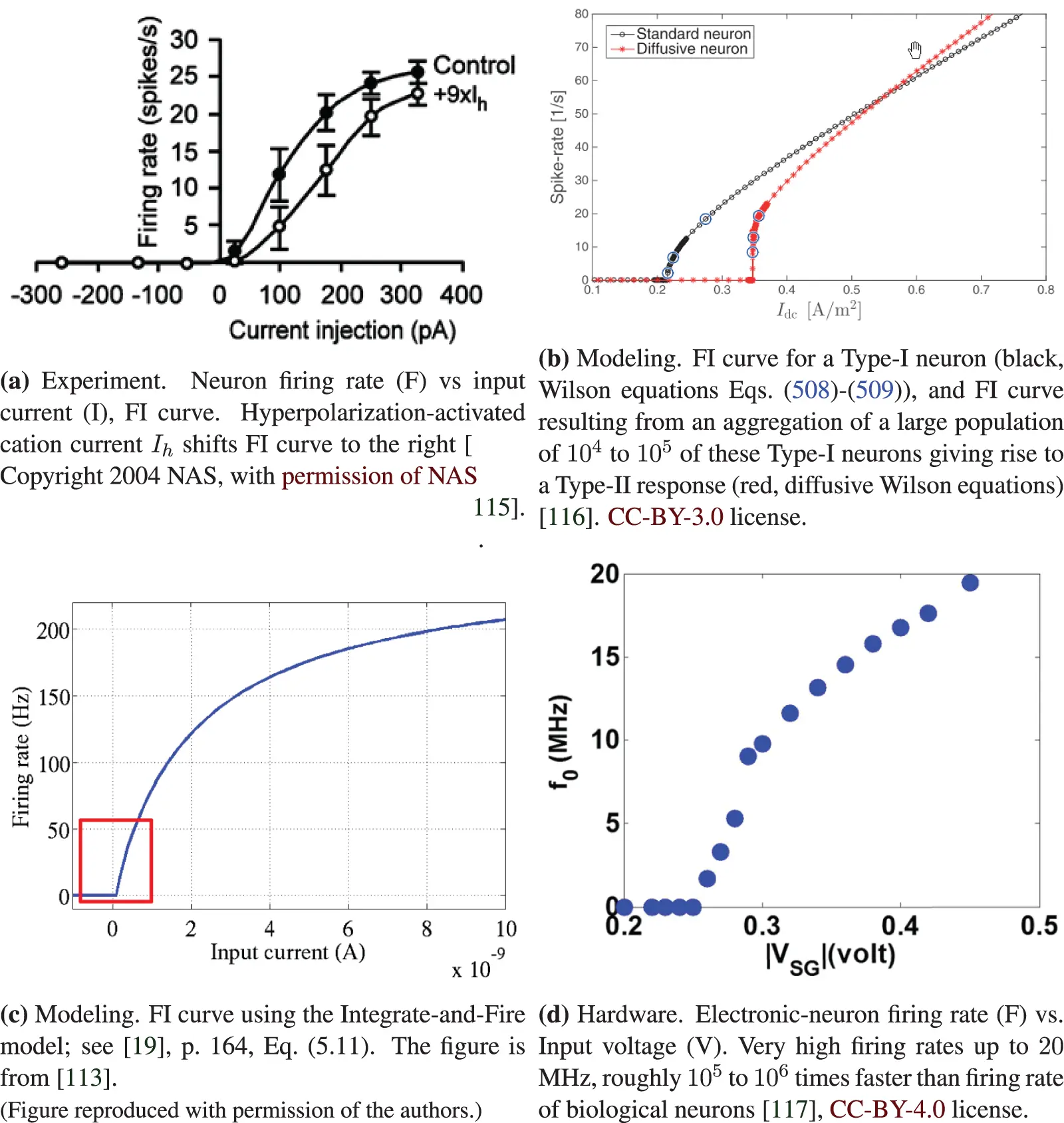Figure 28: FI or FV curves (Sections 3, 4.4.2, 13.2.2). Neuron firing rate (F) versus input current (I) (FI curves, a,b,c) or voltage (V). The Integrate-and-Fire model in SubFigure (c) can be used to replace the sigmoid function to fit the experimental data points in SubFigure (a). The ReLU function in Figure 24 can be used to approximate the region of the FI curve just beyond the current or voltage thresholds, as indicated in the red rectangle in SubFigure (c). Despite the advanced mathematics employed to produce the Type-II FI curve of a large number of Type-I neurons, as shown in SubFigure (b), it is not clear whether a similar result would be obtained if the single neuron displays a behavior as in Figure 27, with transition from Type II to Type I to Type II* in a single neuron. See Section 13.2.2 on “Dynamic, time dependence, Volterra series” for more discussion on Wilson’s equations Eqs. (508)-(509) . On the other hand for deep-learning networks, the above results are more than sufficient to motivate the use of ReLU, which has deep roots in neuroscience.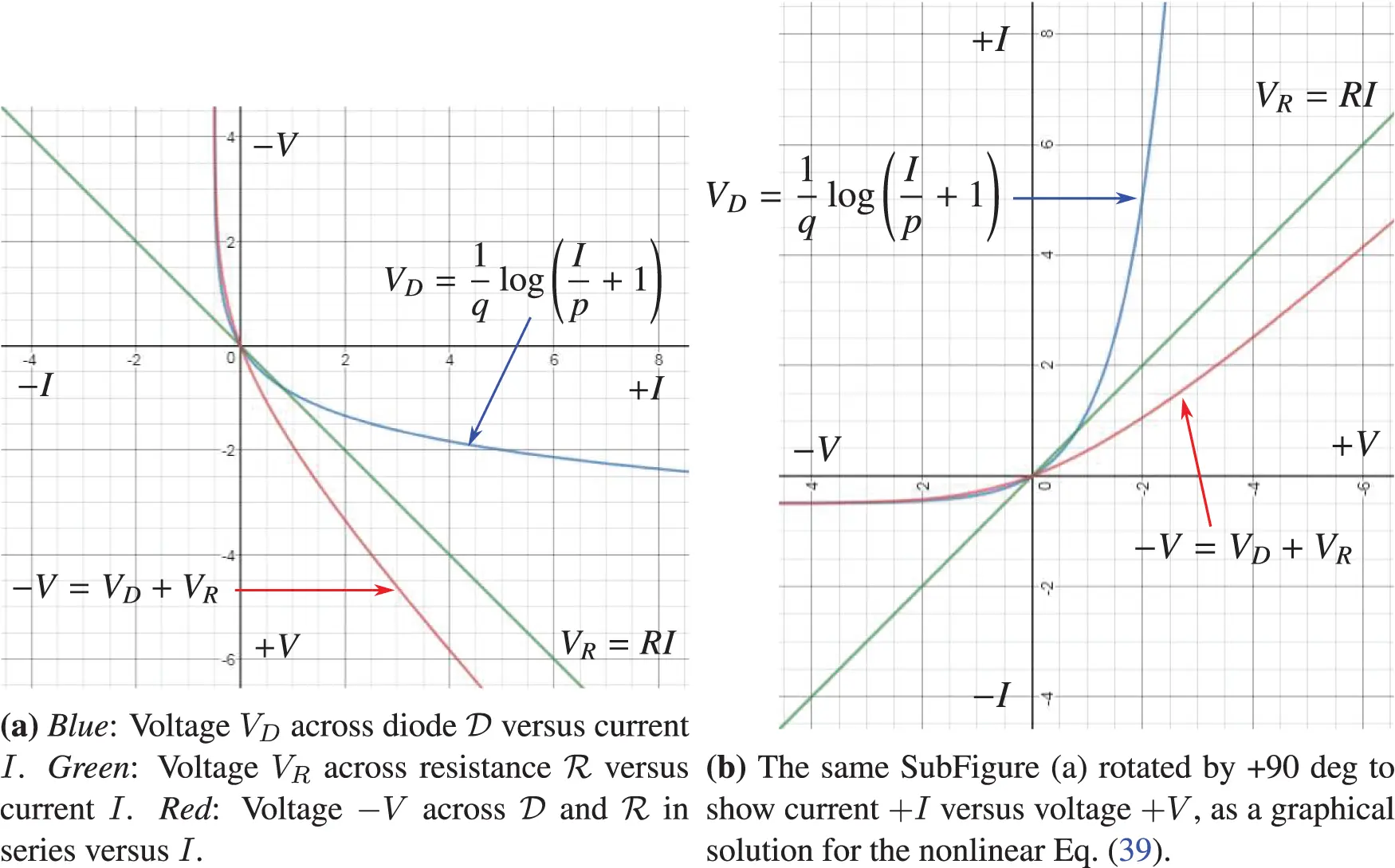Figure 29: Halfwave rectifier (Sections 4.4.2, 5.3.2). Current I versus voltage V [red line in SubFigure (b)] in the halfwave rectifier circuit of Figure 26, for which the ReLU function in Figure 24 is a gross approximation. SubFigure (a) was plotted with p = 0.5, q = −1.2, R = 1. See also Figure 138 for the synaptic response of crayfish similar to the red line in SubFigure (b).

Thus, in addition to the ability to train deep networks, another advantage of using ReLU is the high efficiency in computing both the layer outputs and the gradients for use in optimizing the parameters (weights and biases) to lower cost or loss, i.e., training; see Section 6 on Training, and in particular Section 6.3 on Stochastic Gradient Descent.

The activation function ReLU approximates closer to how biological neurons work than other activation functions (e.g., logistic sigmoid, tanh, etc.), as it was established through experiments some sixty years ago, and have been used in neuroscience long (at least ten years) before being adopted in deep learning in 2011. Its use in deep learning is a clear influence from neuroscience; see Section 13.3 on the history of activation functions, and Section 13.3.2 on the history of the rectified linear function.

Deep-learning networks using ReLU mimic biological neural networks in the brain through a trade-off between two competing properties :

(1)  Sparsity. Only 1% to 4% of brain neurons are active at any one point in time. Sparsity saves brain energy. In deep networks, “rectifying non-linearity gives rise to real zeros of activations and thus truly sparse representations.” Sparsity provides representation robustness in that the non-zero features73 would have small changes for small changes of the data.

(2)  Distributivity. Each feature of the data is represented distributively by many inputs, and each input is involved in distributively representing many features. Distributed representation is a key concept dated since the revival of connectionism with   and others; see Section 13.2.1.Figure 30: Logistic sigmoid function (Sections 4.4.2, 5.1.3, 5.3.1, 13.3.3): s(z)=[1+exp(z)]1=[tanh(z/2)+1]/2 (red), with the tangent at the origin z=0 (blue). See also Remark 5.3 and Figure 46 on the softmax function.Figure 31: Hyperbolic tangent function (Section 4.4.2): g(z)=tanh(z)=2s(2z)1 (red) and its tangent g(z)=z at the coordinate origin (blue), showing that this activation function is identity for small signals.

4.4.3 Graphical representation, block diagrams

The block diagram for a one-layer network is given in Figure 32, with more details in terms of the number of inputs and of outputs given in Figure 33.Figure 32: One-layer network (Section 4.4.3) representing the relation between the predicted output y~ and the input x, i.e., y~=f(x)=a(Wx+b)=a(z), with the weighted sum z:=Wx+b; see Eq. (26) and Eq. (35) with =1. For a lower-level details of this one layer, see Figure 33.

For a multilayer neural network with L layers, with input-output relation shown in Figure 34, the detailed components are given in Figure 35, which generalizes Figure 33 to layer ().Figure 33: One-layer network (Section 4.4.3) in Figure 32: Lower level details, with m processing units (rows or neurons), inputs x=[x1,x2,,xn]T and predicted outputs y~=[y~1,y~2,,y~m]T.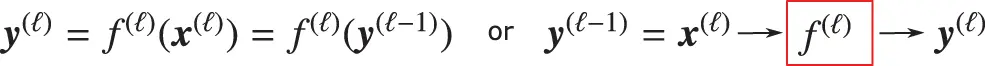Figure 34: Input-to-output mapping (Sections 4.4.3, 4.4.4): Layer () in network with L layers in Figure 23, input-to-output mapping f() for layer ().Figure 35: Low-level details of layer () (Sections 4.4.3, 4.4.4) of the multilayer neural network in Figure 23, with m as the number of processing units (rows or neurons), and thus the width of this layer, representing the layer processing (input-to-output) function f() in Figure 34.

4.4.4 Artificial neuron

And finally, we now complete our top-down descent from the big picture of the overall multilayer neural network with L layers in Figure 23, through Figure 34 for a typical layer () and Figure 35 for the lower-level details of layer (), then down to the most basic level, a neuron in Figure 36 as one row in layer () in Figure 35.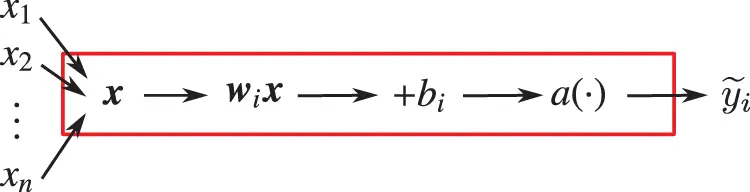Figure 36: Artificial neuron (Sections 2.3.1, 4.4.4, 13.1), row i in layer () in Figure 35, representing the multiple-inputs-to-single-output relation y~i=a(wix+bi)=a(i=1nwijxj+bi) with x=[x1,x2,,xn]T and wi=[wi1,wi2,,win]. This block diagram is the exact equivalent of Figure 8, Section 2.3.1, and in . See Figure 131 for the corresponding biological neuron in Section 13.1 on “Early inspiration from biological neurons”.

4.5 Representing XOR function with two-layer network

The XOR (exclusive-or) function played an important role in bringing down the first wave of AI, known as the cybernetics wave (, p. 14) since it was shown in  that Rosenblatt’s perceptron (1958 , 1962  ) could not represent the XOR function, defined in Table 2: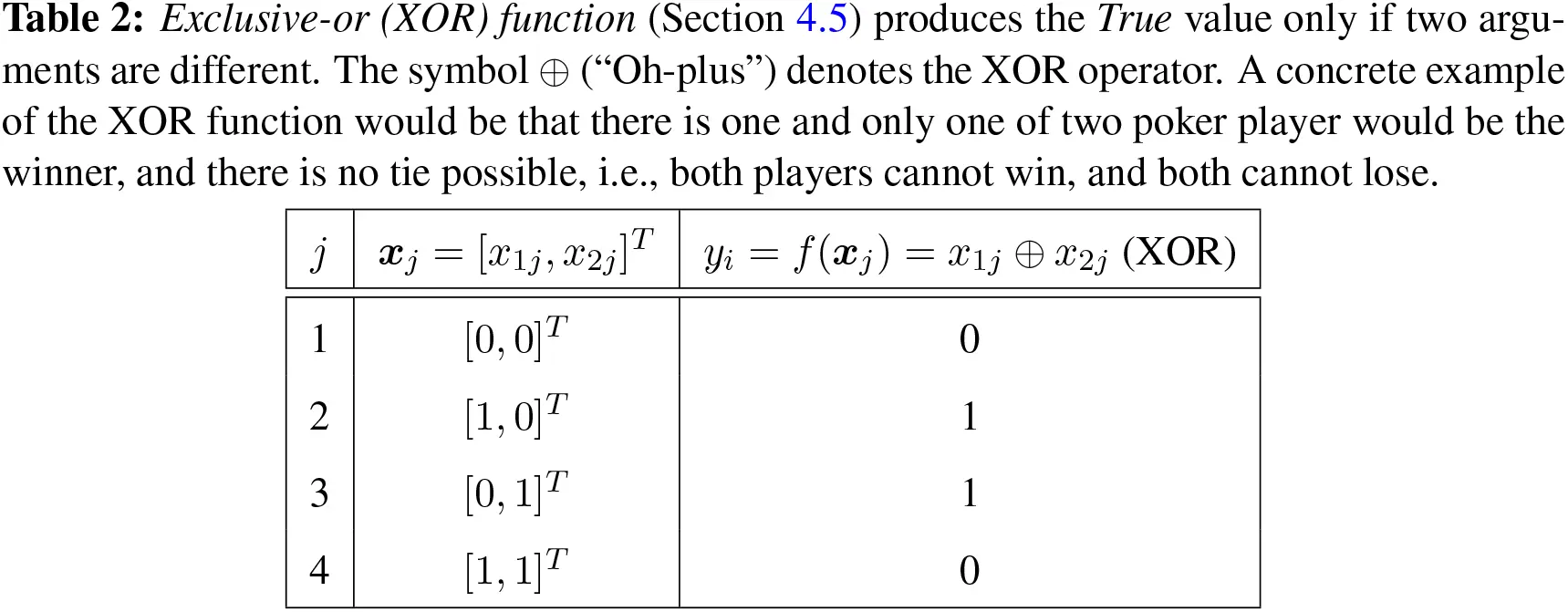The dataset or design matrix74 X is the collection of the coordinates of all four points in Table 2:

X=[x1,,x4]=R2×4, with xiR2×1(41)

An approximation (or prediction) for the XOR function y=f(x) with θ parameters is denoted by y˜=f˜(x,θ), with mean squared error (MSE) being:

J(θ)=14i=14[f˜(xi,θ)f(xi)]2=14i=14[y˜iyi]2(42)

We begin with a one-layer network to show that it cannot represent the XOR function,75 then move on to a two-layer network, which can.

4.5.1 One-layer network

Consider the following one-layer network,76 in which the output y˜ is a linear combination of the coordinates (x1,x2) as inputs:

y˜=f(x,θ)=f(1)(x)=w1(1)x+b1(1)=wx+b=w1x1+w2x2+b,(43)

with the following matrices

w1(1)=w=[w1,w2]R1×2,x=[x1,x2]TR2×1,b1(1)=bR1×1(44)

θ=[θ1,θ2,θ3]T=[w1,w2,b]T(45)

since it is written in , p. 14:

“Model based on the f(x,w)=iwixi used by the perceptron and ADALINE are called linear models. Linear models have many limitations... Most famously, they cannot learn the XOR function... Critics who observed these flaws in linear models caused a backlash against biologically inspired learning in general (Minsky and Papert, 1969). This was the first major dip in the popularity of neural networks.”

First-time learners, who have not seen the definition of Rosenblatt’s (1958) perceptron , could confuse Eq. (43) as the perceptron—which was not a linear model, but more importantly the Rosenblatt perceptron was a network with many neurons77—because Eq. (43) is only a linear unit (a single neuron), and does not have an (nonlinear) activation function. A neuron in the Rosenblatt perceptron is Eq. (489) in Section 13.2, with the Heaviside (nonlinear step) function as activation function; see Figure 132.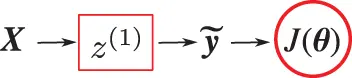Figure 37: Representing XOR function (Sections 4.5, 13.2). This one-layer network (which is not the Rosenblatt perceptron in Figure 132) cannot perform this task. For each input matrix xi in the design matrix X=[x1,,x4]R2×4, with i=1,,4 (see Table 2), the linear unit (neuron) z(1)(xi)=wxi+bR in Eq. (43) predict a value y~i=z(1)(xi) as output, which is collected in the output matrix y~=[y~1,,y~4]R1×4. The MSE cost function J(θ) in Eq. (42) is used in a gradient descent to find the parameters θ=[w,b]. The result is a constant function, y~i=12, for i=1,,4, which cannot represent the XOR function.

The MSE cost function in Eq. (42) becomes

J(θ)=14i=14[b2+(1w1b)2+(1w2b)2+(w1+w2+b)2]2(46)

Setting the gradient of the cost function in Eq. (46) to zero and solving the resulting equations, we obtain the weights and the bias:

θJ(θ)=[Jθ1,Jθ2,Jθ3]T=[Jw1,Jw2,Jb]T(47)

Jw1=02w1+w2+2b=1Jw2=0w1+2w2+2b=1Jb=0w1+w2+2b=1}w1=w2=0,b=12,(48)

from which the predicted output y˜ in Eq. (43) is a constant for any points in the dataset (or design matrix) X=[x1,,x4]:

y˜i=f(xi,θ)=12, for i=1,,4(49)

and thus this one-layer network cannot represent the XOR function. Eqs. (48) are called the “normal” equations.78

4.5.2 Two-layer network

The four points in Table 2 are not linearly separable, i.e., there is no straight line that separates these four points such that the value of the XOR function is zero for two points on one side of the line, and one for the two points on the other side of the line. One layer could not represent the XOR function, as shown above. Rosenblatt (1958)  wrote:

“It has, in fact, been widely conceded by psychologists that there is little point in trying to ‘disprove’ any of the major learning theories in use today, since by extension, or a change in parameters, they have all proved capable of adapting to any specific empirical data. In considering this approach, one is reminded of a remark attributed to Kistiakowsky, that ‘given seven parameters, I could fit an elephant.’ ”

So we now add a second layer, and thus more parameters in the hope to be able to represent the XOR function, as shown in Figure 38.79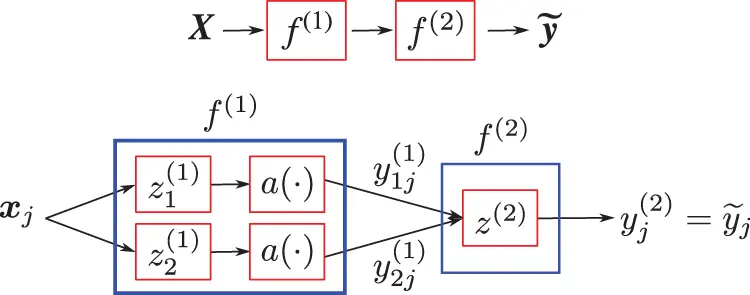Figure 38: Representing XOR function (Sections 4.5). This two-layer network can perform this task. The four points in the design matrix X=[x1,,x4]R2×4 (see Table 2) are converted into three points that are linearly separable by the two nonlinear units (neurons or rows) of Layer (1), i.e., Y(1)=[y1(1),,y1(4)]=f(1)(X(1))=a(Z(1))=X(2)R2×4, with Z(1)=w(1)X(1)+B(1)R2×4 , as in Eq. (58), and a(·) a nonlinear activation function. Layer (2) consists of a single linear unit (neuron or row) with three parameters, i.e., y˜=[y˜1,,y˜4]=f(2)(X(2))=w1(2)X(2)+b1(2)R1×4. The three non-aligned points in X(2) offer three equations to solve for the three parameters θ(2)=[w1(2),b1(2)]R1×3; see Eq. (61).

Layer (1): six parameters (4 weights, 2 biases), plus a (nonlinear) activation function. The purpose is to change coordinates to move the four input points of the XOR function into three points, such that the two points with XOR value equal 1 are coalesced into a single point, and such that these three points are aligned on a straight line. Since these three points remain not linearly separable, the activation function then moves these three points out of alignment, and thus linearly separable.

zi(1)=wi(1)xi+bi(1)=w(1)xi+b(1), for i=1,,4(50)

wi(1)=w(1)=,bi(1)=b(1)=, for i=1,,4(51)

Z(1)=[z1(1),,z4(1)]R2×4,X(1)=[x1(1),,x4(1)]=R2×4(52)

B(1)=[b1(1),,b4(1)]=R2×4(53)

Z(1)=w(1)X(1)+B(1)R2×4.(54)

To map the two points x2=[1,0]T and x3=[0,1]T, at which the XOR value is 1, into a single point, the two rows of w(1) are selected to be identically [1,1] as shown in Eq. (51). The first term in Eq. (54) yields three points aligned along the bisector in the first quadrant (i.e., the line z2=z1 in the z-plane), with all positive coordinates, Figure 39:

w(1)X(1)=R2×4.(55)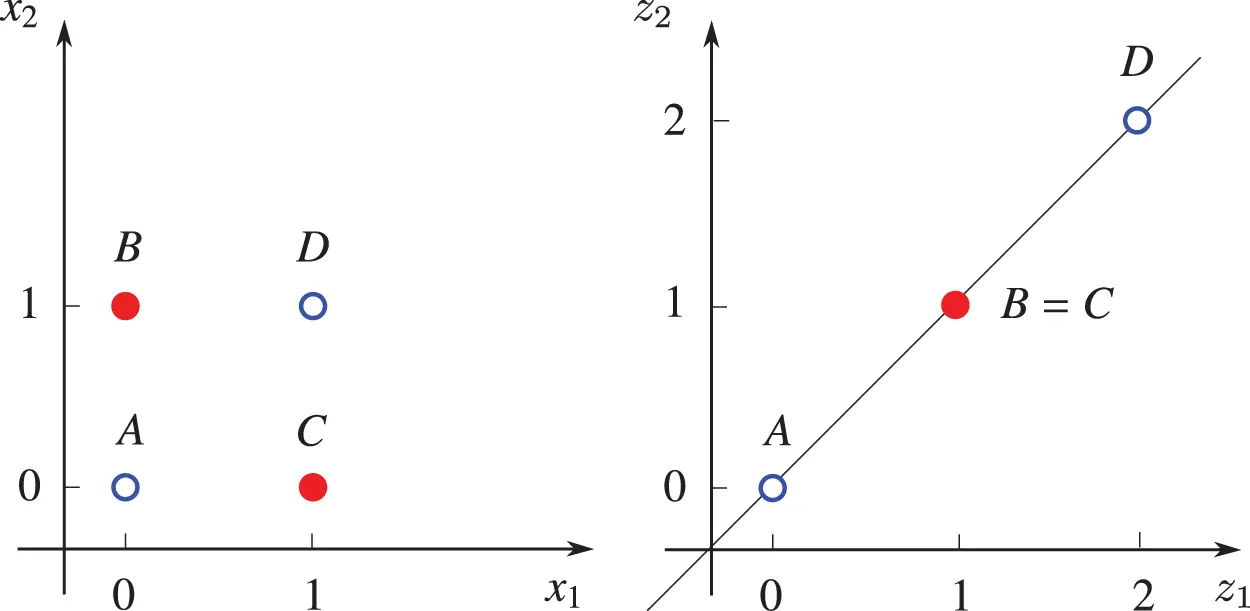Figure 39: Two-layer network for XOR representation (Sections 4.5). Left: XOR function, with A=x1(1)=[0,0]T, B=x2(1)=[0,1]T, C=x3(1)=[1,0]T, D=x4(1)=[1,1]T; see Eq. (52). The XOR value for the solid red dots is 1, and for the open blue dots 0. Right: Images of points A,B,C,D in the z-plane due only to the first term of Eq. (54), i.e., w(1)X(1), which is shown in Eq. (55). See also Figure 40.

For activation functions such as ReLu or Heaviside80 to have any effect, the above three points are next translated in the negative z2 direction using the biases in Eq. (53), so that Eq. (54) yields:

Z(1)=R2×4,(56)

and thus

Y(1)=[y1(1),,y4(1)]=a(Z(1))==X(2)=[x1(2),,x4(2)]R2×4,(57)

For general activation function a(), the outputs of Layer (1) are:

Y(1)=X(2)=a(Z(1))=[a(0)a(1)a(1)a(2)a(1)a(0)a(0)a(1)]R2×4.(58)

Layer (2): three parameters (2 weights, 1 bias), no activation function. Eq. (59) for this layer is identical to Eq. (43) for the one-layer network above, with the output y(1) of Layer (1) as input x(2)=y(1), as shown in Eq. (57):

y˜j=f(xj(2),θ(2))=f(2)(xj(2))=w1(2)xj(2)+b1(2)=wxj(2)+b=w1x1j(2)+w2x2j(2)+b,(59)

with three distinct points in Eq. (57), because x2(2)=x3(2)=[1,0]T, to solve for these three parameters:

θ(2)=[w1(2),b1(2)]=[w1,w2,b](60)

We have three equations:

[y˜1y˜2y˜4]=[a(0)a(1)1a(1)a(0)1a(2)a(1)1][w1w2b]=[y1y2y4]=,(61)

for which the exact analytical solution for the parameters θ(2) is easy to obtain, but the expressions are rather lengthy. Hence, here we only give the numerical solution for θ(2) in the case of the logistic sigmoid function in Table 3.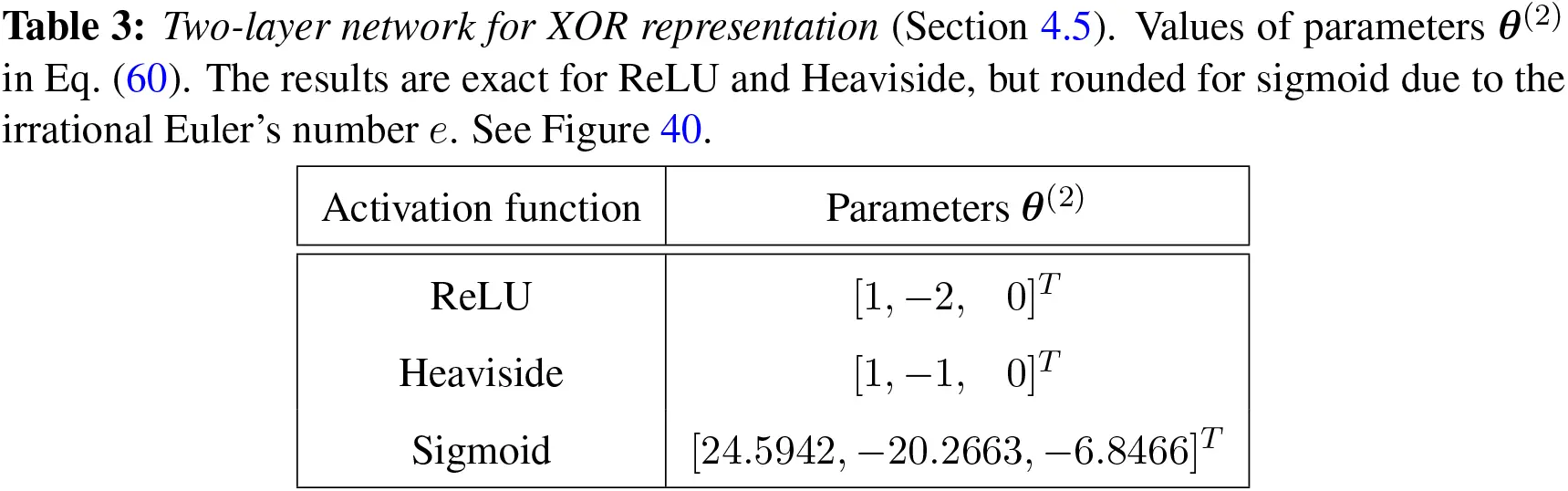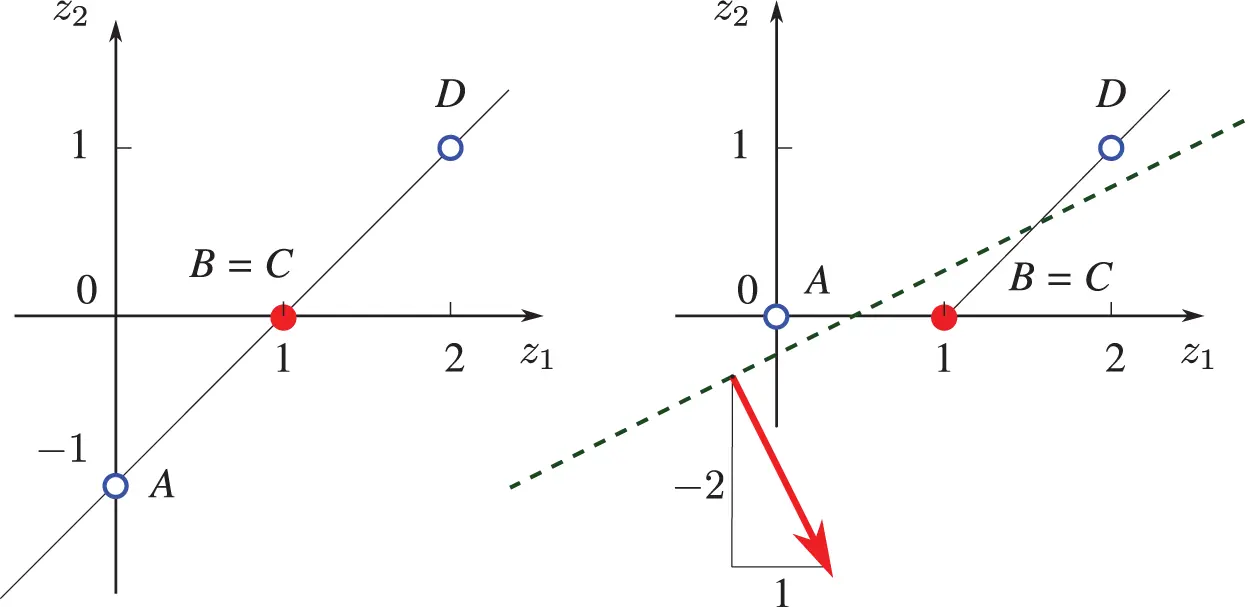Figure 40: Two-layer network for XOR representation (Sections 4.5). Left: Images of points A,B,C,D of Z(1) in Eq. (56), obtained after a translation by adding the bias b(1)=[0,1]T in Eq. (51) to the same points A,B,C,D in the right subfigure of Figure 39. The XOR value for the solid red dots is 1, and for the open blue dots 0. Right: Images of points A,B,C,D after applying the ReLU activation function, which moves point A to the origin; see Eq. (57). the points A, B (=C), D are no longer aligned, and thus linearly separable by the green dotted line, whose normal vector has the components [1,2]T, which are the weights shown in Table 3.

We conjecture that any (nonlinear) function a() in the zoo of activation functions listed, e.g., in “Activation function”, Wikipedia, version 21:00, 18 May 2019 or in  (see Figure 139), would move the three points in Z(1) in Eq. (56) out of alignment, and thus provide the corresponding unique solution θ(2) for Eq. (61).

Remark 4.4. Number of parameters. In 1953, Physicist Freeman Dyson (Princeton Institute of Advanced Study) once consulted with Nobel Laureate Enrico Fermi about a new mathematical model for a difficult physics problem that Dyson and his students had just developed. Fermi asked Dyson how many parameters they had. “Four”, Dyson replied. Fermi then gave his now famous comment “I remember my friend Johnny von Neumann used to say, with four parameters I can fit an elephant, and with five I can make him wiggle his trunk” .

But it was only more than sixty years later that physicists were able to plot an elephant in 2-D using a model with four complex numbers as parameters .

With nine parameters, the elephant can be made to walk (representing the XOR function), and with a billion parameters, it may even perform some acrobatic maneuver in 3-D; see Section 4.6 on depth of multilayer networks.    ■

4.6 What is “deep” in “deep networks” ? Size, architecture

4.6.1 Depth, size

The concept of network depth turns out to be more complex than initially thought. While for a fully-connected feedforward neural network (in which all outputs of a layer are connected to a neuron in the following layer), depth could be considered as the number of layers, there is in general no consensus on the accepted definition of depth. It was stated in , p. 8, that:81

“There is no single correct value for the depth of an architecture,82 just as there is no single correct value for the length of a computer Program. Nor is there a consensus about how much depth a model requires to Qualify as “deep.” ”

For example, keeping the number of layers the same, then the “depth” of a sparsely-connected feedforward network (in which not all outputs of a layer are connected to a neuron in the following layer) should be smaller than the “depth” of a fully-connected feedforward network.

The lack of consensus on the boundary between “shallow” and “deep” networks is echoed in :

“At which problem depth does Shallow Learning end, and Deep Learning begin? Discussions with DL experts have not yet yielded a conclusive response to this question. Instead of committing myself to a precise answer, let me just define for the purposes of this overview: problems of depth > 10 require Very Deep Learning.”

Remark 4.5. Action depth, state depth. In view of Remark 4.3, which type of layer (action or state) were they talking about in the above quotation? We define here action depth as the number of action layers, and state depth as the number of state layers. The abstract network in Figure 23 has action depth L and state depth (L+1), with (L1) as the number of hidden (state) layers.    ■

The review paper  was attributed in  for stating that “training neural networks with more than three hidden layers is called deep learning”, implying that a network is considered “deep” if its number of hidden (state) layers (L1)>3. In the work reported in , the authors used networks with number of hidden (state) layers (L1) varying from one to five, and with a constant hidden (state) layer width of m()=50, for all hidden (state) layers =1,,5; see Table 1 in , reproduced in Figure 99 in Section 10.2.2.

An example of recognizing multidigit numbers in photographs of addresses, in which the test accuracy increased (or test error decreased) with increasing depth, is provided in , p. 196; see Figure 41.Figure 41: Test accuracy versus network depth (Section 4.6.1), showing that test accuracy for this example increases monotonically with the network depth (number of layers). , p. 196. (Figure reproduced with permission of the authors.)

But it is not clear where in  that it was actually said that a network is “deep” if the number of hidden (state) layers is greater than three. An example in image recognition having more than three layers was, however, given in  (emphases are ours):

“An image, for example, comes in the form of an array of pixel values, and the learned features in the first layer of representation typically represent the presence or absence of edges at particular orientations and locations in the image. The second layer typically detects motifs by spotting particular arrangements of edges, regardless of small variations in the edge positions. The third layer may assemble motifs into larger combinations that correspond to parts of familiar objects, and subsequent layers would detect objects as combinations of these parts.”

But the above was not a criterion for a network to be considered as “deep”. It was further noted on the number of the model parameters (weights and biases) and the size of the training dataset for a “typical deep-learning system” as follows  (emphases are ours):

“ In a typical deep-learning system, there may be hundreds of millions of these adjustable weights, and hundreds of millions of labelled examples with which to train the machine.”

See Remark 7.2 on recurrent neural networks (RNNs) as equivalent to “very deep feedforward networks”. Another example was also provided in :

“Recent ConvNet [convolutional neural network, or CNN]83 architectures have 10 to 20 layers of ReLUs [rectified linear units], hundreds of millions of weights, and billions of connections between Units.”84

A neural network with 160 billion parameters was perhaps the largest in 2015 :

“Digital Reasoning, a cognitive computing company based in Franklin, Tenn., recently announced that it has trained a neural network consisting of 160 billion parameters—more than 10 times larger than previous neural networks.

The Digital Reasoning neural network easily surpassed previous records held by Google’s 11.2-billion parameter system and Lawrence Livermore National Laboratory’s 15-billion parameter system.”

As mentioned above, for general network architectures (other than feedforward networks), not only that there is no consensus on the definition of depth, there is also no consensus on how much depth a network must have to qualify as being “deep”; see , p. 8, who offered the following intentionally vague definition:

“Deep learning can be safely regarded as the study of models that involve a greater amount of composition of either learned functions or learned concepts than traditional machine learning does.”

Figure 42 depicts the increase in the number of neurons in neural networks over time, from 1958 (Network 1 by Rosenblatt (1958)  in Figure 42 with one neuron, which was an error in , as discussed in Section 13.2) to 2014 (Network 20 GoogleNet with more than one million neurons), which was still far below the more than ten million biological neurons in a frog.

4.6.2 Architecture

The architecture of a network is the number of layers (depth), the layer width (number of neurons per layer), and the connection among the neurons.85 We have seen the architecture of fully-connected feedforward neural networks above; see Figure 23 and Figure 35.

One example of an architecture different from that fully-connected feedforward networks is convolutional neural networks, which are based on the convolutional integral (see Eq. (497) in Section 13.2.2 on “Dynamic, time dependence, Volterra series”), and which had proven to be successful long before deep-learning networks:

“Convolutional networks were also some of the first neural networks to solve important commercial applications and remain at the forefront of commercial applications of deep learning today. By the end of the 1990s, this system deployed by NEC was reading over 10 percent of all the checks in the United States. Later, several OCR and handwriting recognition systems based on convolutional nets were deployed by Microsoft.” , p. 360.

“Fully-connected networks were believed not to work well. It may be that the primary barriers to the success of neural networks were psychological (practitioners did not expect neural networks to work, so they did not make a serious effort to use neural networks). Whatever the case, it is fortunate that convolutional networks performed well decades ago. In many ways, they carried the torch for the rest of deep learning and paved the way to the acceptance of neural networks in general.” , p. 361.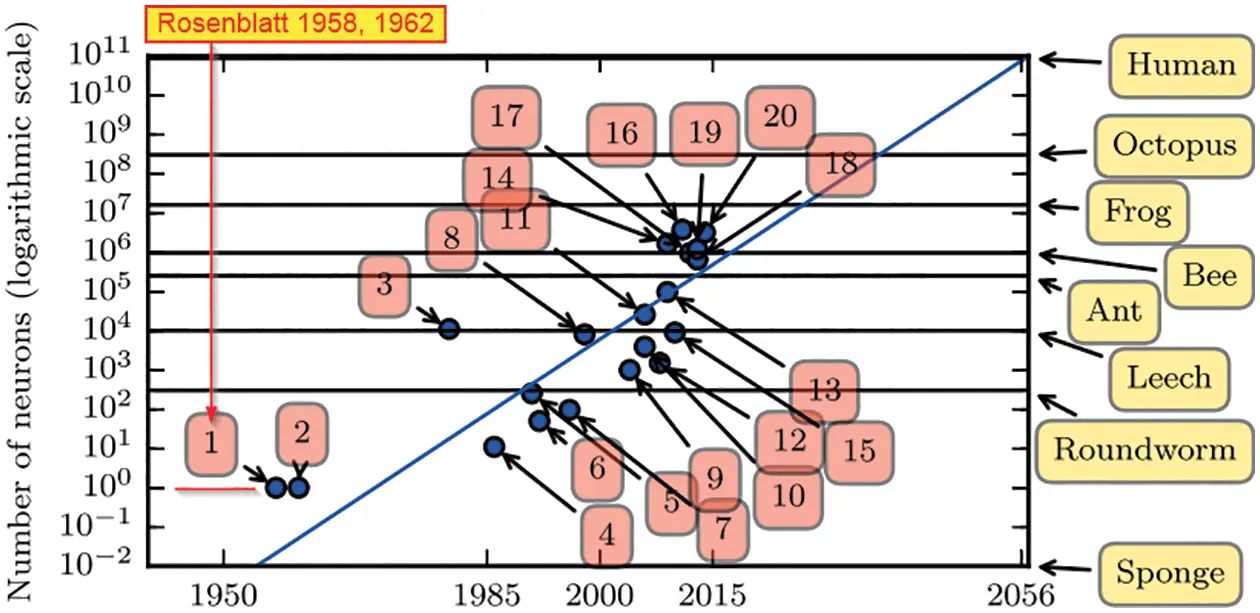Figure 42: Increasing network size over time (Section 4.6.1, 13.2). All networks before 2015 had their number of neurons smaller than that of a frog at 1.6×107, and still far below that in a human brain at 8.6×1010; see “List of animals by number of neurons”, Wikipedia, version 02:46, 9 May 2019. In , p. 23, it was estimated that neural network size would double every 2.4 years (a clear parallel to Moore’s law, which stated that the number of transistors on integrated circuits doubled every 2 years). It was mentioned in , p. 23, that Network 1 by Rosenblatt (1958 , 1962 ) as having one neuron (see figure above), which was incorrect, since Rosenblatt (1957)  conceived a network with 1000 neurons, and even built the Mark I computer to run this network; see Section 13.2 and Figure 133. (Figure reproduced with permission of the authors.)

Here, we present a more recent and successful network architecture different from the fully-connected feedforward network. Residual network was introduced in  to address the problem of vanishing gradient that plagued “very deep” networks with as few as 16 layers during training (see Section 5 on Backpropagation) and the problem of increased training error and test error with increased network depth as shown in Figure 43.

Remark 4.6. Training error, test (generalization) error. Using a set of data, called training data, to find the parameters that minimize the loss function (i.e., doing the training) provides the training error, which is the least square error between the predicted outputs and the training data. Then running the optimally trained model on a different set of data, which was not been used for the training, called test data, provides the test error, also known as generalization error. More details can be found in Section 6, and in , p. 107.    ■

The basic building block of residual network is shown in Figure 44, and a full residual network in Figure 45. The rationale for residual networks was that, if the identity map were optimal, it would be easier for the optimization (training) process to drive the residual (x) down to zero than to fit the identity map with a bunch of nonlinear layers; see , where it was mentioned that deep residual networks won 1st places in several image recognition competitions.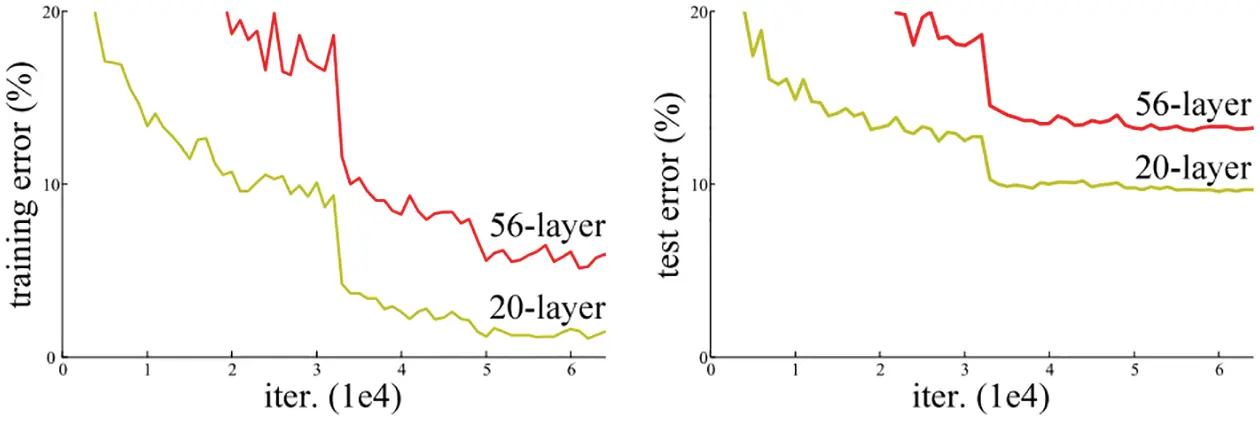Figure 43: Training/test error vs. iterations, depth (Sections 4.6.2, 6). The training error and test error of deep fully-connected networks increased when the number of layers (depth) increased . (Figure reproduced with permission of the authors.)Figure 44: Residual network (Sections 4.6.2, 6), basic building block having two layers with the rectified linear activation function (ReLU), for which the input is x, the output is (x)=(x)+x, where the internal mapping function (x)=(x)x is called the residual. Chaining this building block one after another forms a deep residual network; see Figure 45 . (Figure reproduced with permission of the authors.)

Remark 4.7. The identity map that jumps over a number of layers in the residual network building block in Figure 44 and in the full residual network in Figure 45 is based on a concept close to that for the path of the cell state c[k] in the Long Short-Term Memory (LSTM) unit for recurrent neural networks (RNN), as described in Figure 81 in Section 7.2.    ■

A deep residual network with more than 1,200 layers was proposed in . A wide residual-network architecture that outperformed deep and thin networks was proposed in : “For instance, [their] wide 16-layer deep network has the same accuracy as a 1000-layer thin deep network and a comparable number of parameters, although being several times faster to train.”

It is still not clear why some architecture worked well, while others did not:

“The design of hidden units is an extremely active area of research and does not yet have many definitive guiding theoretical principles.” , p. 186.Figure 45: Full residual network (Sections 4.6.2, 6) with 34 layers, made up from 16 building blocks with two layers each (Figure 44), together with an input and an output layer. This residual network has a total of 3.6 billion floating-point operations (FLOPs with fused multiply-add operations), which could be considered as the network “computational depth” . (Figure reproduced with permission of the authors.)

5  Backpropagation

Backpropagation, sometimes abbreviated as “backprop”, was a child of whom many could claim to be the father, and is used to compute the gradient of the cost function with respect to the parameters (weights and biases); see Section 13.4.1 for a history of backpropagation. This gradient is then subsequently used in an optimization process, usually the Stochastic Gradient Descent method, to find the parameters that minimize the cost or loss function.

5.1 Cost (loss, error) function

Two types of cost function are discussed here: (1) the mean squared error (MSE), and (2) the maximum likelihood (probability cost).86

5.1.1 Mean squared error

For a given input x (a single example) and target output yRm, the squared error (SE) of the predicted output y˜Rm for use in least squared error problem is defined as half the squared error:

J(θ)=12SE=12yy˜2=12k=1k=m(yky˜k)2.(62)

The factor 12 is for the convenience of avoiding to carry the factor 2 when taking the gradient of the cost (or loss) function J.87

While the components yk on the output matrix y cannot be independent and identically distributed (i.i.d.), since y must represent a recognizable pattern (e.g., an image), in the case of training with 𝗆 examples as inputs:88

X={x|1|,,x|𝗆|} and Y={y|1|,,y|𝗆|}(63)

where X is the set of 𝗆 examples, and Y the set of the corresponding outputs, the examples x|k| can be i.i.d., and the half MSE cost function for these outputs is half the expectation of the SE:

J(θ)=12MSE=12E({SE|k|,k=1,,𝗆})=12𝗆k=1k=𝗆y|k|y˜|k|2.(64)

5.1.2 Maximum likelihood (probability cost)

Many (if not most) modern networks employed a probability cost function based in the principle of maximum likelihood, which has the form of negative log-likelihood, describing the cross-entropy between the training data with probability distribution p^data and the model with probability distribution pmodel (, p. 173):

J(θ)=Ex,yp^datalogpmodel(y|x;θ),(65)

where E is the expectation; x and y are random variables for training data with distribution p^data; the inputs x and the target outputs y are values of x and y, respectively, and pmodel is the conditional probability of the distribution of the target outputs y given the inputs x and the parameters θ, with the predicted outputs y˜ given by the model f (neural network), having as arguments the inputs x and the parameters θ:

y˜=f(x,θ)y˜k=fk(x,θ)(66)

The expectations of a function g(x=x) of a random variable x, having a probability distribution P(x=x) for the discrete case, and the probability distribution density p(x=x) for the continuous case, are respectively89

ExPg(x)=xg(x)P(x)=g(x),Expg(x)=g(x)p(x)dx=g(x).(67)

Remark 5.1. Information content, Shannon entropy, maximum likelihood. The expression in Eq. (65)—with the minus sign and the log function—can be abstract to readers not familiar with the probability concept of maximum likelihood, which is related to the concepts of information content and Shannon entropy. First, an event x with low probability (e.g., an asteroid will hit the Earth tomorrow) would have higher information content than an event with high probability (e.g., the sun will rise tomorrow morning). since the probability of x, i.e., P(x), is between 0 and 1, the negative of the logarithm of P(x), i.e.,

I(x)=logP(x),(68)

called the information content of x, would have large values near zero, and small values near 1. In addition, the probability of two independent events to occur is the product of the probabilities of these events, e.g., the probability of having two heads in two coin tosses is

The product (chain) rule of conditional probabilities consists of expressing a joint probability of several random variables {x|1|,x|2|,,x|n|} as the product90

P(x|1|,,x|n|)=P(x|1|)k=2k=nP(x|k||x|1|,,x|k1|)(70)

The logarithm of the products in Eq. (69) and Eq. (70) is the sum of the factor probabilities, and provides another reason to use the logarithm in the expression for information content in Eq. (68): Independent events have additive information. Concretely, the information content of two asteroids independently hitting the Earth should double that of one asteroid hitting the Earth.

The parameters θ~ that minimize the probability cost J(θ) in Eq. (65) can be expressed as91

θ~=argminθEx,yp^datalogpmodel(y|x;θ)=argmaxθ1𝗆k=1k=𝗆logpmodel(y|k||x|k|;θ)(71)

=1𝗆argmaxθlogk=1k=𝗆Pmodel(y|k||x|k|;θ)=argmaxθpmodel(Y|X;θ)(72)

with X={x|1|,,x|𝗆|} and Y={y|1|,,y|𝗆|},(73)

where X is the set of 𝗆 examples that are independent and identically distributed (i.i.d.), and Y the set of the corresponding outputs. The final form in Eq. (72), i.e.,

θ~=argmaxθpmodel(Y|X;θ)(74)

is called the Principle of Maximum Likelihood, in which the model parameters are optimized to maximize the likelihood to reproduce the empirical data.92    ■

Remark 5.2. Relation between Mean Squared Error and Maximum Likelihood. The MSE is a particular case of the Maximum Likelihood. Consider having 𝗆 examples X={x|1|,,x|𝗆|} that are independent and identically distributed (i.i.d.), as in Eq. (63). If the model probability pmodel(y|k||x|k|;θ) has a normal distribution, with the predicted output

y˜|k|=f(x|k|,θ)(75)

as in Eq. (66), predicting the mean of this normal distribution,93 then

pmodel(y|k||x|k|;θ)=𝒩(y|k|;f(x|k|,θ),σ2)=𝒩(y|k|;y˜|k|,σ2)=1[2πσ2]12exp[y|k|y˜|k|22σ2],(76)

with σ designating the standard deviation, i.e., the error between the target output y|k| and the predicted output y˜|k| is normally distributed. By taking the negative of the logarithm of pmodel(y|k||x|k|;θ), we have

logpmodel(y|k||x|k|;θ)=12log(2πσ2)y|k|y˜|k|22σ2.(77)

Then summing Eq. (77) over all examples k=1,,𝗆 as in the last expression in Eq. (71) yields

k=1k=𝗆logpmodel(y|k||x|k|;θ)=𝗆2log(2πσ2)k=1k=𝗆y|k|y˜|k|22σ2,(78)

and thus the minimizer θ˜ in Eq. (71) can be written as

θ˜=argmaxθk=1k=𝗆y|k|y˜|k|22σ2=argminθk=1k=𝗆y|k|y˜|k|22σ2=argminθJ(θ),(79)

where the MSE cost function J(θ) was defined in Eq. (64), noting that constants such as m or 2m do not affect the value of the minimizer θ~.

Thus finding the minimizer of the maximum likelihood cost function in Eq. (65) is the same as finding the minimizer of the MSE in Eq. (62); see also , p. 130.    ■

Remark 5.2 justifies the use of Mean Squared Error as a Maximum Likelihood estimator.94 For the purpose of this review paper, it is sufficient to use the MSE cost function in Eq. (42) to develop the backpropagation procedure.

5.1.3 Classification loss function

In classification tasks—such as used in , Section 10.2, and Footnote 265—a neural network is trained to predict which of k different classes (categories) an input x belongs to. The most simple classification problem only has two classes (k=2), which can be represented by the values {0,1} of a single binary variable y. The probability distribution of such single boolean-valued variable is called Bernoulli distribution.95 The Bernoulli distribution is characterized by a single parameter p(y=1|x), i.e., the conditional probability of x belonging to the class y=1. To perform binary classification, a neural network is therefore trained to estimate the conditional probability distribution y˜=p(y=1|x) using the principle of maximum likelihood (see Section 5.1.2, Eq. (65)):

J(θ)=Ex,y~p^datalogpmodel(y|x;θ)      =1𝗆k=1k=𝗆{ y|k|logpmodel(y|k|=1|x|k|;θ)+(1y|k|)log(1pmodel(y|k|=1|x|k|;θ)) }.(80)

The output of the neural network is supposed to represent the probability pmodel(y=1|x;θ), i.e., a real-valued number in the interval [0,1]. A linear output layer y˜=y(L)=z(L)=W(L)y(L1)+b(L) does not meet this constraint in general. To squash the output of the linear layer into the range of [0,1], the logistic sigmoid function s (see Figure 30) can be added to the linear output unit to render z(L) a probability

y˜=y(L)=a(z(L)),a(z(L))=s(z(L)).(81)

In case more than two categories occur in a classification problem, a neural network is trained to estimate the probability distribution over the discrete number (k>2) of classes. Such distribution is referred to as multinoulli or categorial distribution, which is parameterized by the conditional probabilities pi=p(y=i|x)[0,1],i=1,,k of an input x belonging to the i-th category. The output of the neural network accordingly is a k-dimensional vector y˜Rk×1, where y˜i=p(y=i|x). In addition to the requirement of each component y˜i being in the range [0,1], we must also guarantee that all components sum up to 1 to satisfy the definition of a probability distribution.

For this purpose, the idea of exponentiation and normalization, which can be expressed as a change of variable in the logistic sigmoid function s (Figure 30, Section 5.3.1), as in the following example , p. 177:

p(y)=s[(2y1)z]=11+exp[(2y1)z], with y{0,1} and z=constant,(82)

p(0)+p(1)=s(z)+s(z)=11+exp(z)+11+exp(z)=1,(83)

The softmax function converts the vector formed by a linear unit z(L)=W(L)y(L1)+b(L)Rk into the vector of probabilities y˜ by means of

y˜i=(softmaxz(L))i=expzi(L)j=1j=kexpzj(L),(84)

and is a smoothed version of the max function , p. 198.96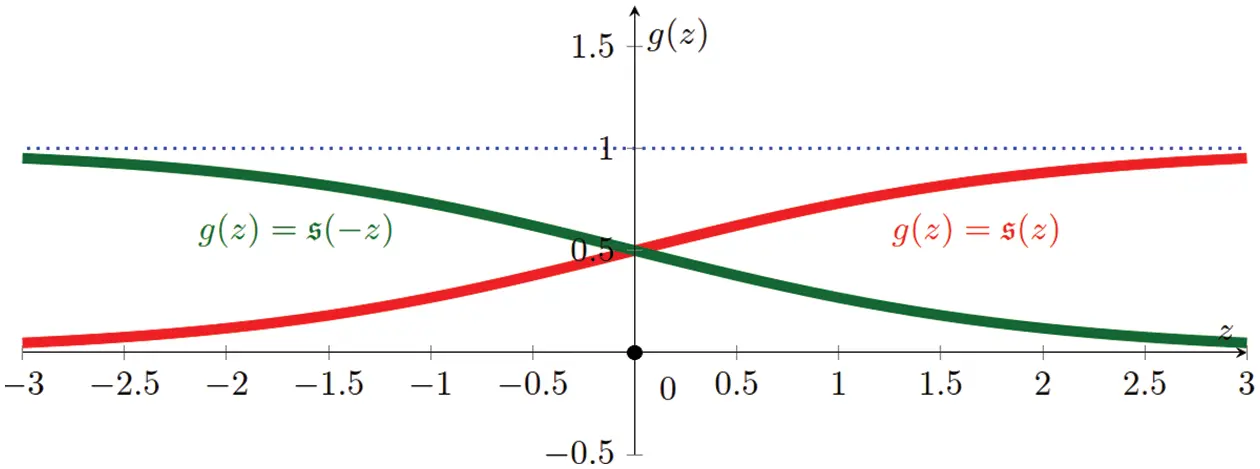Figure 46: Sofmax function for two classes, logistic sigmoid (Section 5.1.3, 5.3.1): s(z)=[1+exp(z)]1 and s(z)=[1+exp(z)]1, such that s(z)+s(z)=17. See also Figure 30.

Remark 5.3. Softmax function from Bayes’ theorem. For a classification with multiple classes {𝒞k,k=1,,K}, particularized to the case of two classes with K=2, the probability for class 𝒞1, given the input column matrix x, is obtained from Bayes’ theorem97 as follows (, p. 197):

p(𝒞1|x)=p(x|𝒞1)p(𝒞1)p(x)=p(x,𝒞1)p(x,𝒞1)+p(x,𝒞2)=exp(z)exp(z)+1=11+exp(z)=s(z),(85)

with z:=lnp(x,𝒞1)p(x,𝒞2)p(x,𝒞1)p(x,𝒞2)=exp(z),(86)

where the product rule was applied to the numerator of Eq. (85)2, the sum rule to the denominator, and s the logistic sigmoid. Likewise,

p(𝒞2|x)=p(x,𝒞2)p(x,𝒞1)+p(x,𝒞2)=1exp(z)+1=s(z)(87)

p(𝒞1|x)+p(𝒞2|x)=s(z)+s(z)=1,(88)

as in Eq. (83), and s is also called a normalized exponential or softmax function for K=2. Using the same procedure, for K>2, the softmax function (version 1) can be written as98

p(𝒞i|x)=[1+j=1,jiKexp(zij)]1, with zij:=lnp(x,𝒞i)p(x,𝒞j).(89)

Using a different definition, the softmax function (version 2) can be written as

p(𝒞i|x)=exp(zi)j=1Kexp(zj), with zi:=lnp(x,𝒞i),(90)

which is the same as Eq. (84).    ■

5.2 Gradient of cost function by backpropagation

The gradient of a cost function J(θ) with respect to the parameters θ is obtained using the chain rule of differentiation, and backpropagation is an efficient way to compute the chain rule. In the forward propagation, the computation (or function composition) moves from the first layer (1) to the last layer (L); in the backpropagation, the computation moves in reverse order, from the last layer (L) to the first layer (1).

Remark 5.4. We focus our attention on developing backpropagation for fully-connected networks, for which an explicit derivation was not provided in , but would help clarify the pseudocode.99 The approach in  was based on computational graph, which would not be familiar to first-time learners from computational mechanics, albeit more general in that it was applicable to networks with more general architecture, such as those with skipped connections, which require keeping track of parent and child processing units for constructing the path of backpropagation. See also Appendix 1 where the backprop Algorithm 1 below is rewritten in a different form to explain the equivalent Algorithm 6.4 in , p. 206.    ■

It is convenient to recall here some equations developed earlier (keeping the same equation numbers) for the computation of the gradient J/θ() of the cost function J(θ) with respect to the parameters θ() in layer (), going backward from the last layer =L,,1.

•   Cost function J(θ):

J(θ)=12MSE=12𝗆yy˜2=12𝗆k=1k=𝗆(yky˜k)2,(62)

•   Inputs x=y(0)Rm(0)×1 with m(0)=n and predicted outputs y(L)=y˜Rm(L)×1 with m(0)=m:

y(0)=x(1)=x (inputs) y()=f()(x())=x(+1) with =1,,L1y(L)=f(L)(x(L))=y˜ (predicted outputs) (19)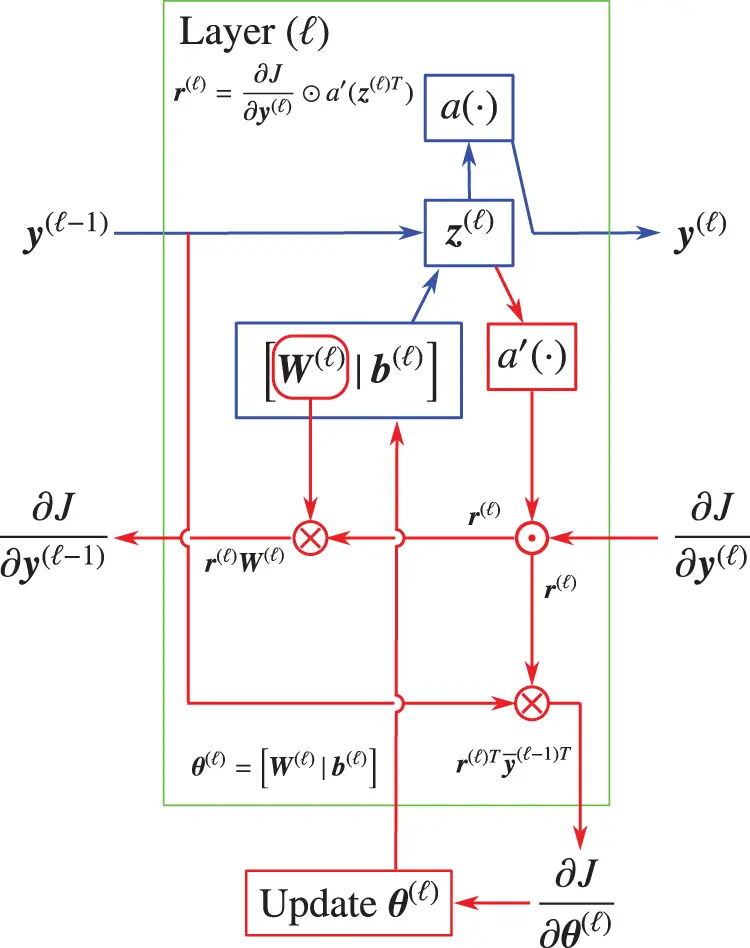Figure 47: Backpropagation building block, typical layer () (Section 5.2, Algorithm 1, Appendix 1). The forward propagation path is shown in blue, with the backpropagation path in red. The update of the parameters θ() in layer () is done as soon as the gradient J/θ() is available using a gradient descent algorithm. The row matrix r()=J/z() in Eq. (104) can be computed once for use to evaluate both the gradient J/θ() in Eq. (105) and the gradient J/y(1) in Eq. (106), then discarded to free up memory. See pseudocode in Algorithm 1.

•   Weighted sum of inputs and biases z()Rm()×1:

z()=W()y(1)+b() such that zi()=wi()y(1)+bi() ,  for i=1,,m(),(26)

•   Network parameters θ and layer parameters θ()Rm()×[m(1)+1]:

Θ()=[ θij() ]=[ θ1()θm()() ]=[ W()|b() ]m()×[m(1)+1](30)

θ={ θ(1),,θ(),,θ(L) }={ Θ(1),,Θ(),,Θ(L) }, such that θ()Θ()(31)

•   Expanded layer outputs y¯(1)[m(1)+1]×1:

z()=W()y(1)+b()[ W()|b() ][ y(1)1 ]=:θ()y¯(1),(32)

•   Activation function a():

y()=a(z()) such that yi()=a(zi())(35)

The gradient of the cost function J(θ) with respect to the parameters θ() in layer (), for =L,,1, is simply:

J(θ)θ()=J(θ)y()y()θ()Jθij()=k=1m()Jyk()yk()θij()(91)

J(θ)θ()=[Jθij()]Rm()×[m(1)+1],J(θ)y()=[Jyk()]R1×m() (row)(92)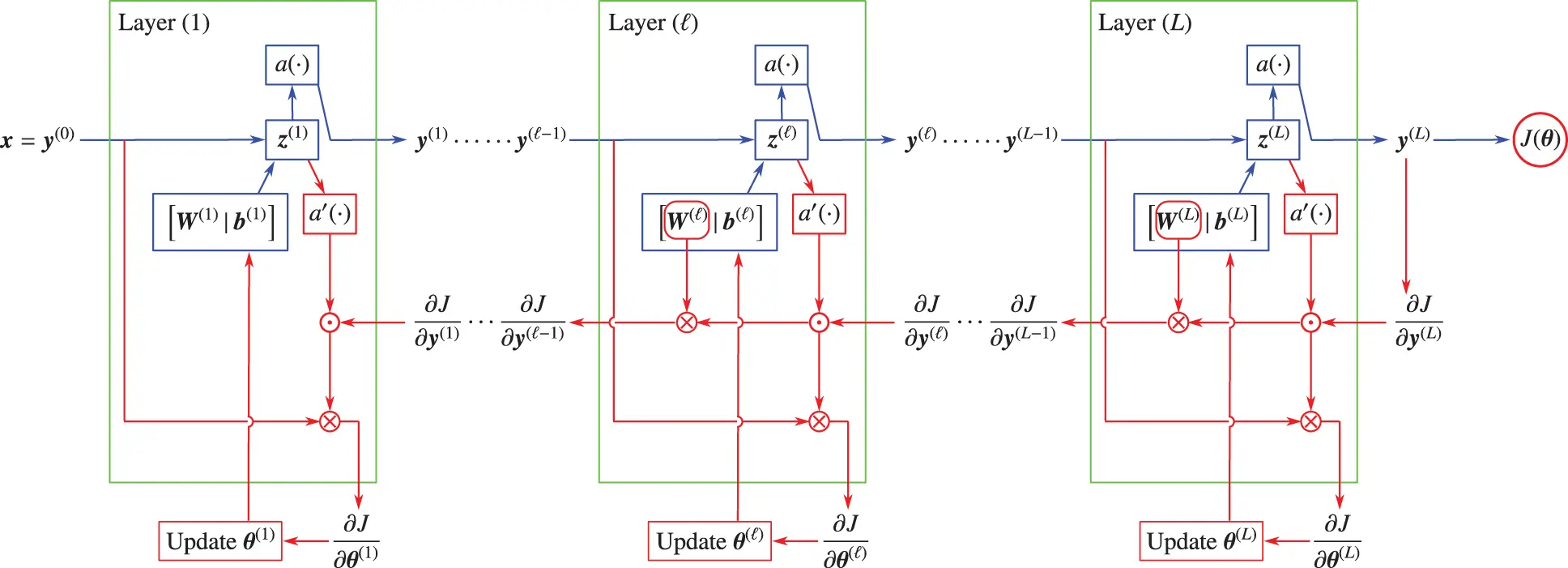Figure 48: Backpropagation in fully-connected network (Section 5.2, 5.3, Algorithm 1, Appendix 1). Starting from the predicted output y~=y(L) In the last layer (L) at the end of any forward propagation (blue arrows), and going backward (red arrows) to the first layer with =L,,1, and along the way at layer (), compute the gradient of the cost function J relative the the parameters θ() to update those parameters in a gradient descent, then compute the gradient of J relative to the outputs y(1) of the lower-level layer (1) to continue the backpropagation. See pseudocode in Algorithm 1. For a particular example of the above general case, see Figure 51.

The above equations are valid for the last layer =L, since since the predicted output y˜ is the same as the output of the last layer (L), i.e., y˜y(L) by Eq. (19). Similarly, these equations are also valid for the first layer (1) since the input for layer (1) is x(1)=x=y(0). using Eq. (35), we obtain (no sum on k)

yk()θij()=aʹ(zk())zk()θij()=paʹ(zk())θkp()θij()y¯p(1)=paʹ(zk())δkiδpjy¯p(1)=aʹ(zk())δkiy¯j(1)(93)

Using Eq. (93) in Eq. (91) leads to the expressions for the gradient, both in component form (left) and in matrix form (right):

Jθij()=Jyi()aʹ(zi())y¯j(1)(no sum on i)[ Jθij() ]= [ [ Jyi() ]T[aʹ(zi())] ][ y¯j(1) ]T,(94)

where is the elementwise multiplication, known as the Hadamard operator, defined as follows:

[pi],[qi]Rm×1[pi][qi]=[(piqi)]=[p1q1,,pmqm]TRm×1 (no sum on i),(95)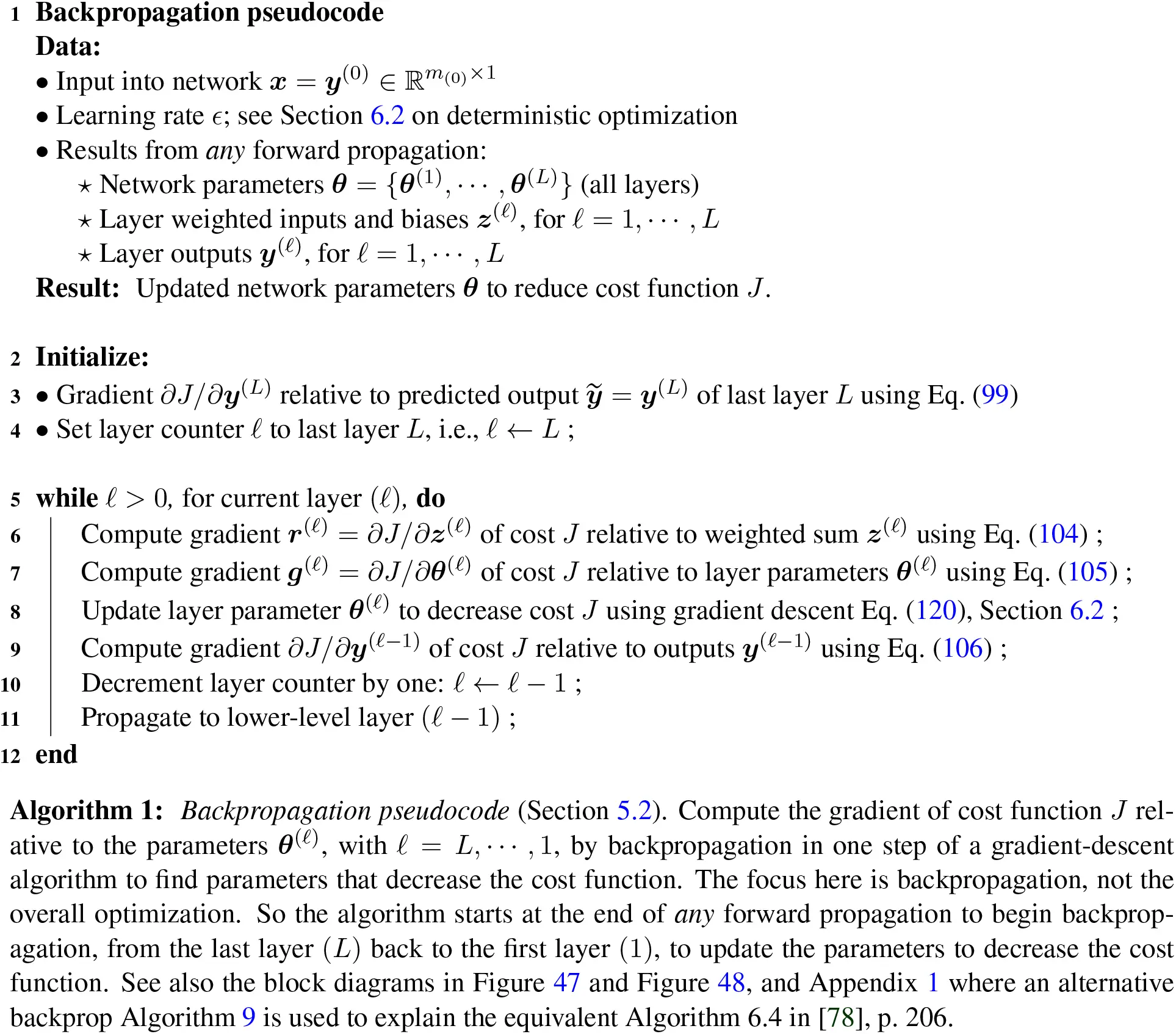and

[ Jyi() ]1×m()(row), z()=[ zk() ]m()×1(column)aʹ(zi())m()×1(column)(96)

[ [ Jyi() ]T  [aʹ(zi())] ]m()×1(column,no sum on i),  and[ y¯j(1) ][m(1)+1]×1(column)(97)

[ Jθij() ]= [ [ Jyi() ]T  [aʹ(zi())]  ][ y¯j(1) ]Tm()×[m(1)+1],(98)

which then agrees with the matrix dimension in the first expression for J/θ() in Eq. (92). For the last layer =L, all terms on the right-hand side of Eq. (98) are available for the computation of the gradient J/θij(L) since

y(L)=y˜Jy(L)=Jy˜=1𝗆k=1k=𝗆(y˜|k|y|k|)R1×m(L) (row)(99)

with m being the number of examples and m(L) the width of layer (L), is the mean error from the expression of the cost function in Eq. (64), with zi(L) and y¯j(L1) already computed in the forward propagation. To compute the gradient of the cost function with respect to the parameters θ(L1) in layer (L1), we need the derivative J/yi(L1), per Eq. (98). Thus, in general, the derivative of cost function J with respect to the output matrix y(1) of layer (1), i.e., J/yi(1), can be expressed in terms of the previously computed derivative J/yi() and other quantities for layer () as follows:

Jyi(1)=kJyk()yk()yi(1)=kJyk()aʹ(zk())wki()=kJzk()zk()yi(1)(100)

[ Jyi(1) ]= [ [ Jyk() ][aʹ(zk())]T ][ wki() ]1×m(1)(no sum on k)(101)

[ Jyi(1) ]1×m(1),[ Jyk() ]1×m()(row),   [aʹ(zk())]m()×1(column)(102)

[ Jyk() ][aʹ(zk())]T=Jzk()1×m()(no sum on k),[ wki() ]=zk()yi(1)m()×m(1).(103)

Comparing Eq. (101) and Eq. (98), when backpropagation reaches layer (), the same row matrix

r():=Jz()= [ [ Jyi() ][aʹ(zi())]T ] =Jy()aʹ(z()T)1×m()(row)(104)

is only needed to be computed once for use to compute both the gradient of the cost J relative to the parameters θ() [see Eq. (98) and Figure 47]

Jθ()=r()Ty¯(1)TRm()×[m(1)+1](105)

and the gradient of the cost J relative to the outputs y(1) of layer (1) [see Eq. (101) and Figure 47]

Jy(1)=rW()R1×m(1).(106)

The block diagram for backpropagation at layer ()—as described in Eq. (104), Eq. (105), Eq. (106)—is given in Figure 47, and for a fully-connected network in Figure 48, with pseudocode given in Algorithm 1.

To demonstrate the vanishing gradient problem, a network is used in , having an input layer containing 784 neurons, corresponding to the 28×28=784 pixels in the input image, four hidden layers, with each hidden layer containing 30 neurons, and an output layer containing 10 neurons, corresponding to the 10 possible classifications for the MNIST digits (’0’, ’1’, ’2’,..., ’9’). A key ingredient is the use of the sigmoid function as active function; see Figure 30.

We note immediately that the vanishing / exploding gradient problem can be resolved using the rectified linear function (ReLu, Figure 24) as active function in combination with “normalized initialization”100 and “intermediate normalization layers”, which are mentioned in , and which we will not discuss here.

The speed of learning of a hidden layer () in Figure 49 is defined as the norm of the gradient g() of the cost function J(θ) with respect to the parameters θ() in the hidden layer ():

g()∥=||Jθ()||(107)

The speed of learning in each of the four layers as a function of the number of epochs101 of training drops down quickly after less than 50 training epochs, then plateaued out, as depicted in Figure 49, where the speed of learning of layer (1) was 100 times less than that of layer (4) after 400 training epochs.Figure 49: Vanishing gradient problem (Section 5.3). Speed of learning of earlier layers is much slower than that of later layers. Here, after 400 epochs of training, the speed of learning of Layer (1) at 105 (blue line) is 100 times slower than that of Layer (4) at 103 (green line); , Chapter 5, ‘Why are deep neural networks hard to train ?’ (CC BY-NC 3.0).

To understand the reason for the quick and significant decrease in the speed of learning, consider a network with four layers, having one scalar input x with target scalar output y, and predicted scalar output y˜, as shown in Figure 50, where each layer has one neuron.102 The cost function and its derivative are

J(θ)=12(yy˜)2,Jy=yy˜(108)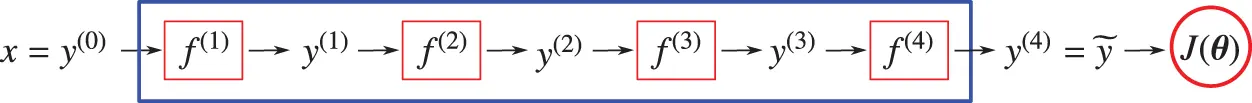Figure 50: Neural network with four layers (Section 5.3), one neuron per layer, scalar input x, scalar output y, cost function J(θ)=12(yy~)2, with y˜=y(4) being the target output and also the output of layer (4), such that f()(y(1))=a(z()), with a() being the active function, z()=w()y(1)+b(), for =1,,4, and the network parameters are θ=[w1,,w4,b1,,b4]. The detailed block diagram is in Figure 51.

The neuron in layer () accepts the scalar input y(1) to produce the scalar output y() according to

f()(y(1))=a(z()), with z()=w()y(1)+b().(109)

As an example of computing the gradient, the derivative of the cost function J with respect to the bias b(1) of layer (1) is given by

Jb(1)=(yy˜)[aʹ(z(4))w(4)][aʹ(z(3))w(3)][aʹ(z(2))w(2)][aʹ(z(1))w(1)](110)

The back propagation procedure to compute the gradient J/b(1) in Eq. (110) is depicted in Figure 51, which is a particular case of the more general Figure 48.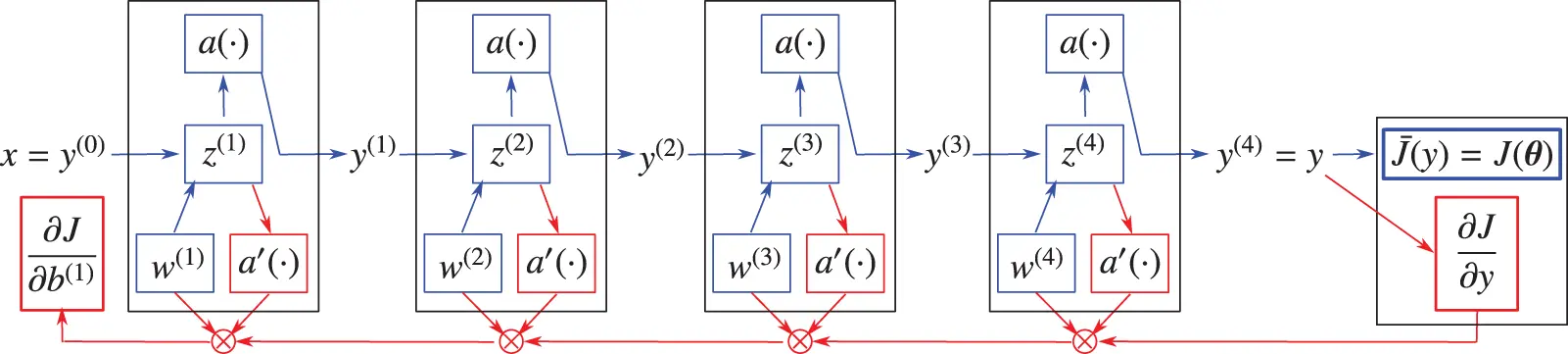Figure 51: Neural network with four layers in Figure 50 (Section 5.3). Detailed block diagram. Forward propagation (blue arrows) and backpropagation (red arrows). In the forward propagation wave, at each layer (), the product aʹw() is computed and stored, awaiting for the chain-rule derivative to arrive at this layer to multiply. The cost function J(θ) is computed together with its derivative J/y, which is the backprop starting point, from which, when following the backpropagation red arrow, the order of the factors are as in Eq. (110), until the derivative J/b(1) is reached at the head of the backprop red arrow. (Only the weights are shown, not the biases, which are not needed in the back propagation, to save space.) The speed of learning is slowed down significantly in early layers due to vanishing gradient, as shown in Figure 49. See also the more general case in Figure 48.

Whether the gradient Jb(1) in Eq. (110) vanishes or explodes depends on the magnitude of its factors

In other mixed cases, the problem of vanishing or exploding gradient could be alleviated by the changing of the magnitude |aʹ(z())w()|, above 1 and below 1, from layer to layer.

Remark 5.5. While the vanishing gradient problem for multilayer networks (static case) may be alleviated by weights that vary from layer to layer (the mixed cases mentioned above), this problem is especially critical in the case of Recurrent Neural Networks, since the weights stay constant for all state numbers (or “time”) in a sequence of data. See Remark 7.3 on “short-term memory” in Section 7.2 on Long Short-Term Memory. In back-propagation through the states in a sequence of data, from the last state back to the first state, the same weight keeps being multiplied by itself. Hence, when a weight is less than 1, successive powers of its magnitude eventually decrease to zero when progressing back the first state.    ■

5.3.1 Logistic sigmoid and hyperbolic tangent

The first derivatives of the sigmoid function and hyperbolic tangent function depicted in Figure 30 (also in Remark 5.3 on the softmax function and Figure 46) are given below:

a(z)=s(z)=11+exp(z)aʹ(z)=sʹ(z)=s(z)[1s(z)](0,1)(113)

a(z)=tanh(z)aʹ(z)=tanhʹ(z)=11+z2(0,1](114)

and are less than 1 in magnitude (everywhere for the sigmoid function, and almost everywhere for the hyperbolic tangent tanh function), except at z=0, where the derivative of the tanh function is equal to 1; Figure 52. Successive multiplications of these derivatives will result in smaller and smaller values along the back propagation path. If the weights w() in Eq. (110) are also smaller than 1, then the gradient J/b(1) will tend toward 0, i.e., vanish. The problem is further exacerbated in deeper networks with increasing number of layers, and thus increasing number of factors less than 1 (i.e., |aʹ(z())w())|<1). We have encountered the vanishing-gradient problem.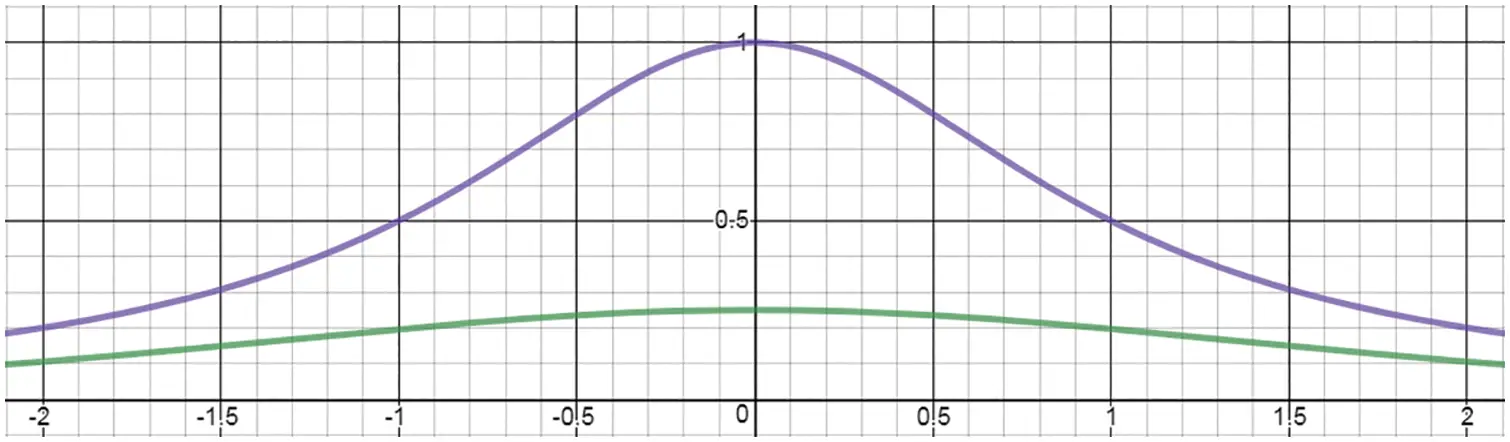Figure 52: Sigmoid and hyperbolic tangent functions, derivative (Section 5.3.1). The derivative of sigmoid function (sʹ(z)=s(z)[1s(z)], green line) is less than 1 everywhere, whereas the derivative of the hyperbolic tangent (tanhʹ(z)=(1+z2)1, purple line) is less than 1 everywhere, except at the abscissa z=0, where it is equal to 1.

The exploding gradient problem is opposite to the vanishing gradient problem, and occurs when the gradient has its magnitude increases in subsequent multiplications, particularly at a “cliff”, which is a sharp drop in the cost function in the parameter space.103 The gradient at the brink of a cliff (Figure 53) leads to large-magnitude gradients, which when multiplied with each other several times along the back propagation path would result in an exploding gradient problem.

5.3.2 Rectified linear function (ReLU)

The rectified linear function depicted in Figure 24 with its derivative (Heaviside function) equal to 1 for any input greater than zero, would resolve the vanishing-gradient problem, as it is written in :

“For a given input only a subset of neurons are active. Computation is linear on this subset ... Because of this linearity, gradients flow well on the active paths of neurons (there is no gradient vanishing effect due to activation non-linearities of sigmoid or tanh units), and mathematical investigation is easier. Computations are also cheaper: there is no need for computing the exponential function in activations, and sparsity can be exploited.”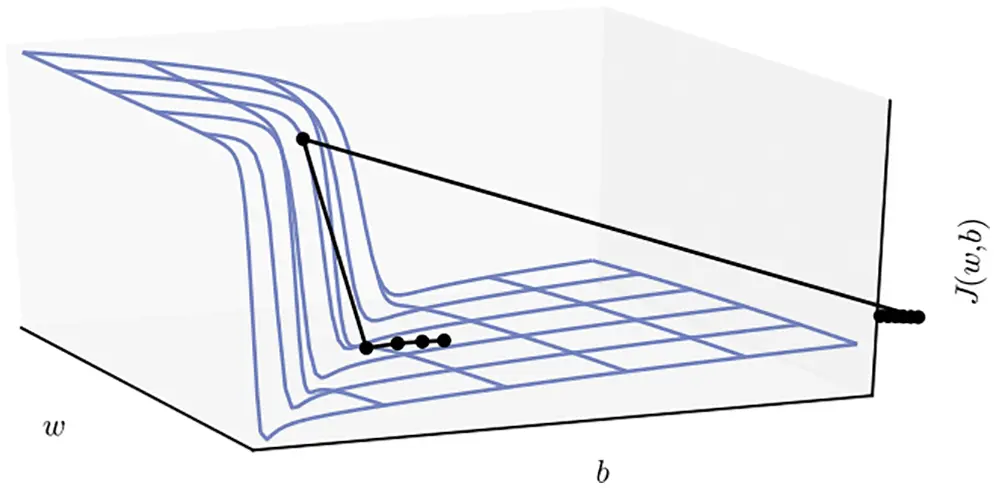Figure 53: Cost-function cliff (Section 5.3.1). A cliff, or a sharp drop in the cost function. The parameter space is represented by a weight w and a bias b. The slope at the brink of the cliff leads to large-magnitude gradients, which when multiplied with each other several times along the back propagation path would result in an exploding gradient problem. , p. 281. (Figure reproduced with permission of the authors.)

A problem with ReLU was that some neurons were never activated, and called “dying” or “dead”, as described in :

“However, ReLU units are at a potential disadvantage during optimization because the gradient is 0 whenever the unit is not active. This could lead to cases where a unit never activates as a gradient-based optimization algorithm will not adjust the weights of a unit that never activates initially. Further, like the vanishing gradients problem, we might expect learning to be slow when training ReL networks with constant 0 gradients.”

To remedy this “dying” or “dead” neuron problem, the Leaky ReLU, proposed in ,104 had the expression already given previously in Eq. (40), and can be viewed as an approximation to the leaky diode in Figure 29. Both ReLU and Leaky ReLU have been known and used in neuroscience for years before being imported into artificial neural network; see Section 13 for a historical review.

5.3.3 Parametric rectified linear unit (PReLU)

Instead of arbitrarily fixing the slope s of the Leaky ReLU at 0.01 for negative z as in Eq. (40), it is proposed to leave this slope s as a free parameter to optimize along with the weights and biases ; see Figure 54:

a(z)=max(sz,z)={sz for z0z for 0<z(115)

and thus the network adaptively learned the parameters to control the leaky part of the activation function. Using the Parametric ReLU in Eq.(115), a deep convolutional neural network (CNN) in  was able to surpass the level of human performance in image recognition for the first time in 2015; see Figure 3 on ImageNet competition results over the years.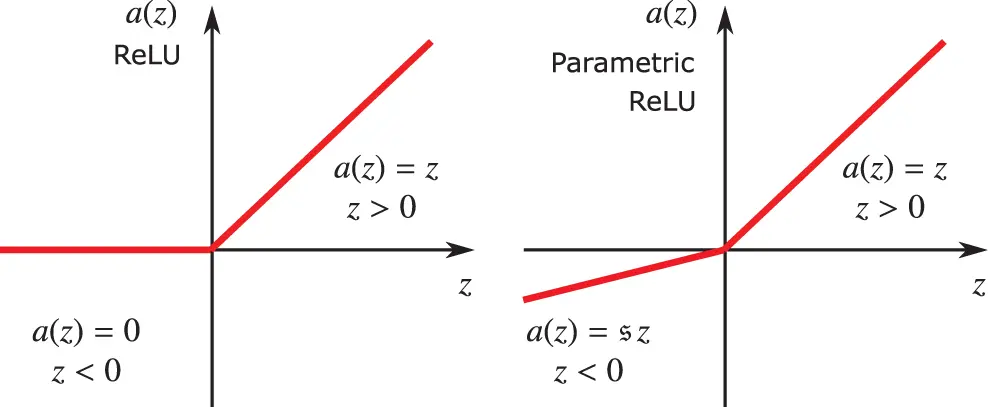Figure 54: Rectified Linear Unit (ReLU, left) and Parametric ReLU (right) (Section 5.3.2), in which the slope s is a parameter to optimize; see Section 5.3.3. See also Figure 24 on ReLU.Figure 55: Cost-function landscape (Section 6). Residual network with 56 layers (ResNet-56) on the CIFAR-10 training set. Highly non-convex, with many local minima, and deep, narrow valleys . The training error and test error for fully-connected network increased when the number of layers was increased from 20 to 56, Figure 43, motivating the introduction of residual network, Figure 44 and Figure 45, Section 4.6.2. (Figure reproduced with permission of the authors.)

6  Network training, optimization methods

For network training, i.e., to find the optimal network parameters θ that minimize the cost function J(θ), we describe here both deterministic optimization methods used in full-batch mode,105 and stochastic optimization methods used in minibatch106 mode.

Figure 55 shows the highly non-convex landscape of the cost function of a residual network with 56 layers trained using the CIFAR-10 dataset (Canadian Institute For Advanced Research), a collection of images commonly used to train machine learning and computer vision algorithms, containing 60,000 32x32 color images in 10 different classes, representing airplanes, cars, birds, cats, deer, dogs, frogs, horses, ships, and trucks. Each class has 6,000 images.107

Deterministic optimization methods (Section 6.2) include first-order gradient method (Algorithm 2) and second-order quasi-Newton method (Algorithm 3), with line searches based on different rules, introduced by Goldstein, Armijo, and Wolfe.

Stochastic optimization methods (Section 6.3) include

•   First-order stochastic gradient descent (SGD) methods (Algorithm 4), with add-on tricks such as momentum and accelerated gradient

•   Criticism of adaptive methods and SGD resurgence with add-on tricks such as effective tuning and step-length decay (or annealing)

•   Classical line search with stochasticity: SGD with Armijo line search (Algorithm 6), second-order Newton method with Armijo-like line search (Algorigthm 7)Figure 56: Training set, validation set, test set (Section 6.1). Partition of whole dataset. The examples are independent. The three subsets are identically distributed.

6.1 Training set, validation set, test set, stopping criteria

The classical (old) thinking—starting in 1992 with  and exemplified by Figures 57, 58, 59, 60 (a, left)—would surprise first-time learners that minimizing the training error is not optimal in machine learning. A reason is that training a neural network is different from using “pure optimization” since it is desired to decrease not only the error during training (called training error, and that’s pure optimization), but also the error committed by a trained network on inputs never seen before.108 Such error is called generalization error or test error. This classical thinking, known as the bias-variance trade-off, has been included in books since 2001  (p. 194) and even repeated in 2016  (p. 268). Models with lower number of parameters have higher bias and lower variance, whereas models with higher number of parameters have lower bias and higher variance; Figure 59.109

The modern thinking is exemplified by Figure 60 (b, right) and Figure 61, and does not contradict the intuitive notion that decreasing the training error to zero is indeed desirable, as overparameterizing networks beyond the interpolation threshold (zero training error) in modern practice generalizes well (small test error). In Figure 61, the test error continued to decrease significantly with increasing number of parameters N beyond the interpolation threshold N=825, whereas the classical regime (N<N) with the bias-variance trade-off (blue) in Figure 59 was restrictive, and did not generalize as well (larger test error). Beyond the interpolation threshold N, variance can be decreased by using ensemble average, as shown by the orange line in Figure 61.

Such modern practice was the motivation for research into shallow networks with infinite width as a first step to understand how overparameterized networks worked so well; see Figure 148 and Section 14.2 “Lack of understanding on why deep learning worked.”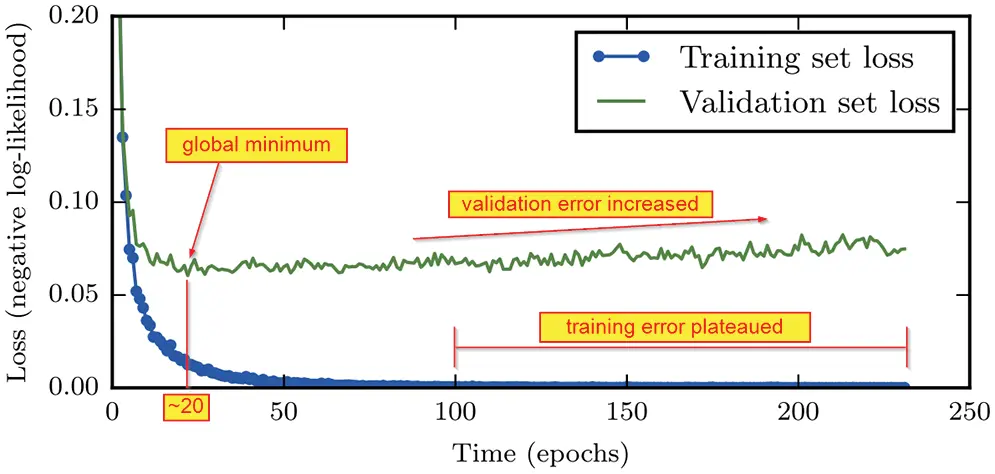Figure 57: Training and validation learning curves—Classical viewpoint (Section 6.1), i.e., plots of training error and validation errors versus epoch number (time). While the training cost decreased continuously, the validation cost reaches a minimum around epoch 20, then started to gradually increase, forming an “asymmetric U-shaped curve.” Between epoch 100 and epoch 240, the training error was essentially flat, indicating convergence. Adapted from , p. 239. See Figure 60 (a, left), where the classical risk curve is the classical viewpoint, whereas the modern interpolation viewpoint is on the right subfigure (b). (Figure reproduced with permission of the authors.)

To develop a neural-network model, a dataset governed by the same probability distribution, such as the CIFAR-10 dataset mentioned above, can be typically divided into three non-overlapping subsets called training set, validation set, and test set. The validation set is also called the development set, a terminology used in , in which an effective method of step-length decay was proposed; see Section 6.3.4.

It was suggested in , p. 61, to use 50% of the dataset as training set, 25% as validation set, and 25% as test set. On the other hand, while a validation set with size about 1/4 of the training set was suggested in , p. 118, there was no suggestion for the relative size of the test set.110 See Figure 56 for a conceptual partition of the dataset.

Examples in the training set are fed into an optimizer to find the network parameter estimate θ˜ that minimizes the cost function estimate J˜(θ~).111 As the optimization on the training set progresses from epoch to epoch,112 examples in the validation set are fed as inputs into the network to obtain the outputs for computing the cost function J˜val(θ~τ), also called validation error, at predetermined epochs { τκ } using the network parameters {θ˜τκ} obtained from the optimization on the training set at those epochs.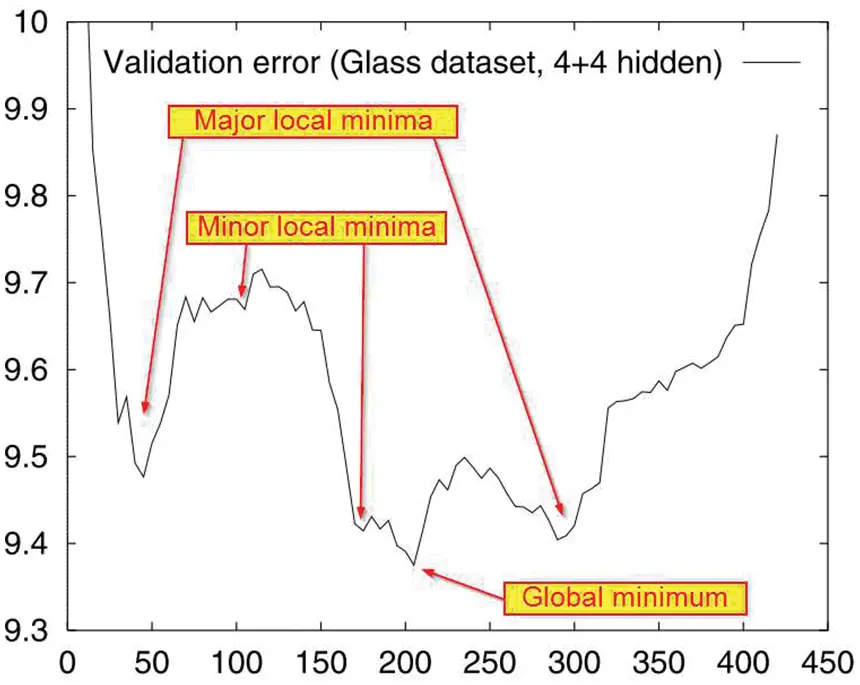Figure 58: Validation learning curve (Section 6.1, Algorithm 4). Validation error vs epoch number. Some validation error could oscillate wildly around the mean, resulting in an “ugly reality”. The global minimum validation error corresponded to epoch number τ. Since the stopping criteria may miss this global minimum, it was suggested to monitor the validation learning curve to find the epoch τ at which the network parameters θ˜τ would be declared optimal. Adapted from , p. 55. (Figure reproduced with permission of the authors.)

Figure 57 shows the different behaviour of the training error versus that of the validation error. The validation error would decrease quickly initially, reaching a global minimum, then gradually increased, whereas the training error continued to decrease and plateaued out, indicating that the gradients got smaller and smaller, and there was not much decrease in the cost. From epoch 100 to epoch 240, the traning error was at about the same level, with litte noise. The validation error, on the other hand, had a lot of noise.

Because of the “asymmetric U-shaped curve” of the validation error, the thinking was that if the optimization process could stop early at the global mininum of the validation error, then the generalization (test) error, i.e., the value of cost function on the test set, would also be small, thus the name “early stopping”. The test set contains examples that have not been used to train the network, thus simulating inputs never seen before. The validation error could have oscillations with large amplitude around a mean curve, with many local minima; see Figure 58.

The difference between the test (generalization) error and the validation error is called the generalization gap, as shown in the bias-variance trade-off  Figure 59, which qualitatively delineates these errors versus model capacity, and conceptually explains the optimal model capacity as where the generalization gap equals the training error, or the generalization error is twice the training error.

Remark 6.1. Even the best machine learning generalization capability nowadays still cannot compete with the generalization ability of human babies; see Section 14.6 on “What’s new? Teaching machines to think like babies”.    ■

Early-stopping criteria. One criterion is to first define the lowest validation error from epoch 1 up to the current epoch τ as: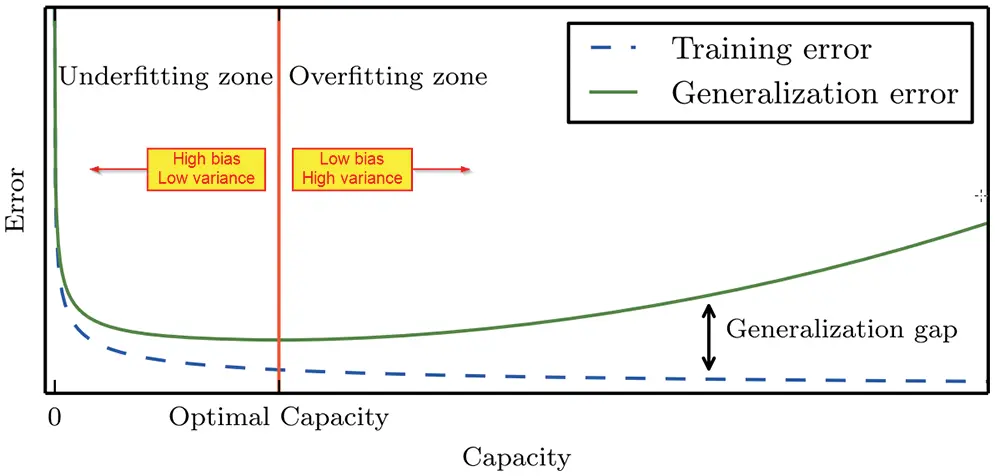Figure 59: Bias-variance trade-off (Section 6.1). Training error (cost) and test error versus model capacity. Two ways to change the model capacity: (1) change the number of network parameters, (2) change the values of these parameters (weight decay). The generalization gap is the difference between the test (generalization) error and the training error. As the model capacity increases from underfit to overfit, the training error decreases, but the generalization gap increases, past the optimal capacity. Figure 72 gives examples of underfit, appropriately fit, overfit. See , p. 112. The above is the classical viewpoint, which is still prevalent ; see Figure 60 for the modern viewpoint, in which overfitting with high capacity model generalizes well (small test error) in practice. (Figure reproduced with permission of the authors.)

J˜val(θ˜τ)=minττ{J˜val(θ˜τ)},(116)

then define the generalization loss (in percentage) at epoch τ as the increase in validation error relative to the minimum validation error from epoch 1 to the present epoch τ:

G(τ)=100(J~val(θ~τ)J~val(θ~τ)1).(117)

 then defined the “first class of stopping criteria” as follows: Stop the optimization on the training set when the generalization loss exceeds a certain threshold 𝗌 (generalization loss lower bound):

G𝗌:Stop after epoch τ if G(τ)>𝗌.(118)

The issue is how to determine the generalization loss lower bound 𝗌 so not to fall into a local minimum, and to catch the global minimum; see Figure 58. There were many more early-stopping criterion classes in . But it is not clear whether all these increasingly sophisticated stopping criteria would work to catch the validation-error global minimum in Figure 58.

Moreover, the above discussion is for the classical regime in Figure 60 (a). In the context of the modern interpolation regime in Figure 60 (b), early stopping means that the computation would cease as soon as the training error reaches “its lowest possible value (typically zero [beyond the interpolation threshold], unless two identical data points have two different labels)” . See the green line in Figure 61.

Computational budget, learning curves. A simple method would be to set an epoch budget, i.e., the largest number of epochs for computation sufficiently large for the training error to go down significantly, then monitor graphically both the training error (cost) and the validation error versus epoch number. These plots are called the learning curves; see Figure 57, for which an epoch budget of 240 was used. Select the global minimum of the validation learning curve, with epoch number τ (Figure 57), and use the corresponding network parameters θ˜τ, which were saved periodically, as optimal paramters for the network.113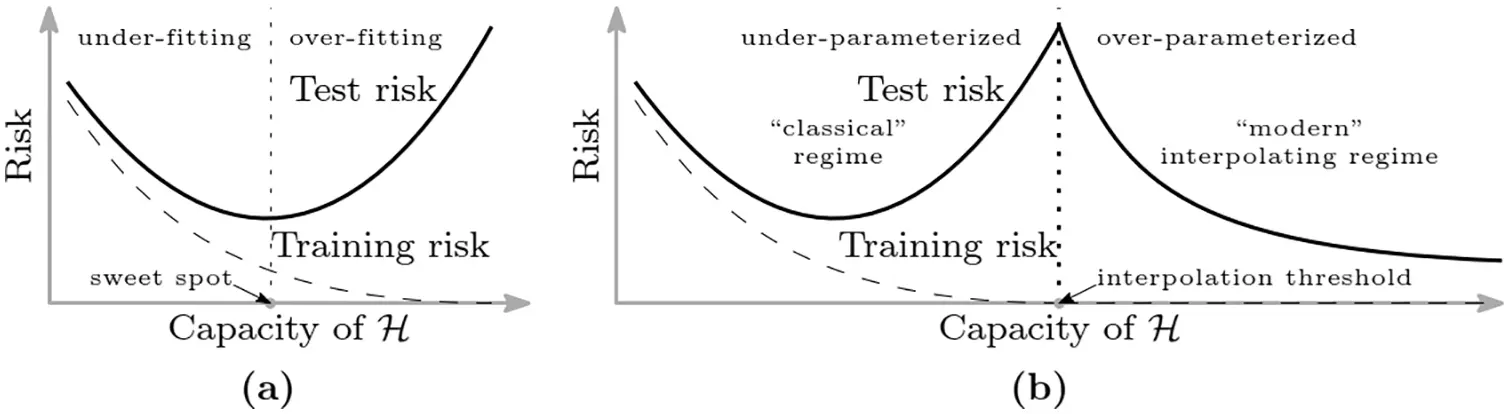Figure 60: Modern interpolation regime (Sections 6.1, 14.2). Beyond the interpolation threshold, the test error goes down as the model capacity (e.g., number of parameters) increases, describing the observation that networks with high capacity beyond the interpolation threshold generalize well, even though overfit in training. Risk = error or cost. Capacity = number of parameters (but could also be increased by weight decay). Figures 57, 59 corresponds to the classical regime, i.e., old method (thinking) . See Figure 61 for experimental evidence of the modern interpolation regime, and Figure 148 for a shallow network with infinite width. Permission of NAS.

Remark 6.2. Since it is important to monitor the validation error during training, a whole section is devoted in  (Section 8.1, p. 268) to expound on “How Learning Differs from Pure Optimization”. And also for this reason, it is not clear yet what global optimization algorithms such as in  could bring to network training, whereas the stochastic gradient descent (SGD) in Section 6.3.1 is quite efficient; see also Section 6.5.9 on criticism of adaptive methods.    ■

Remark 6.3. Epoch budget, global iteration budget. For stochastic optimization algorithms—Sections 6.3, 6.5, 6.6, 6.7—the epoch counter is τ and the epoch budget τmax. Numerical experiments in Figure 73 had an epoch budget of τmax=250, whereas numerical experiments in Figure 74 had an epoch budget of τmax=1800. The computational budget could be specified in terms of global iteration counter j as jmax. Figure 71 had a global iteration budget of jmax=5000.    ■

Before presenting the stochastic gradient-descent (SGD) methods in Section 6.3, it is important to note that classical deterministic methods of optimization in Section 6.2 continue to be useful in the age of deep learning and SGD.

“One should not lose sight of the fact that [full] batch approaches possess some intrinsic advantages. First, the use full gradient information at each iterate opens the door for many deterministic gradient-based optimization methods that have been developed over the past decades, including not only the full gradient method, but also accelerated gradient, conjugate gradient, quasi-Newton, inexact Newton methods, and can benefit from parallelization.” , p. 237.

6.2 Deterministic optimization, full batch

Once the gradient J/θ()Rm()×[m(1)+1] of the cost function J has been computed using backpropagation described in Section 5.2, the layer parameters θ() are updated to decrease cost function J using gradient descent as follows: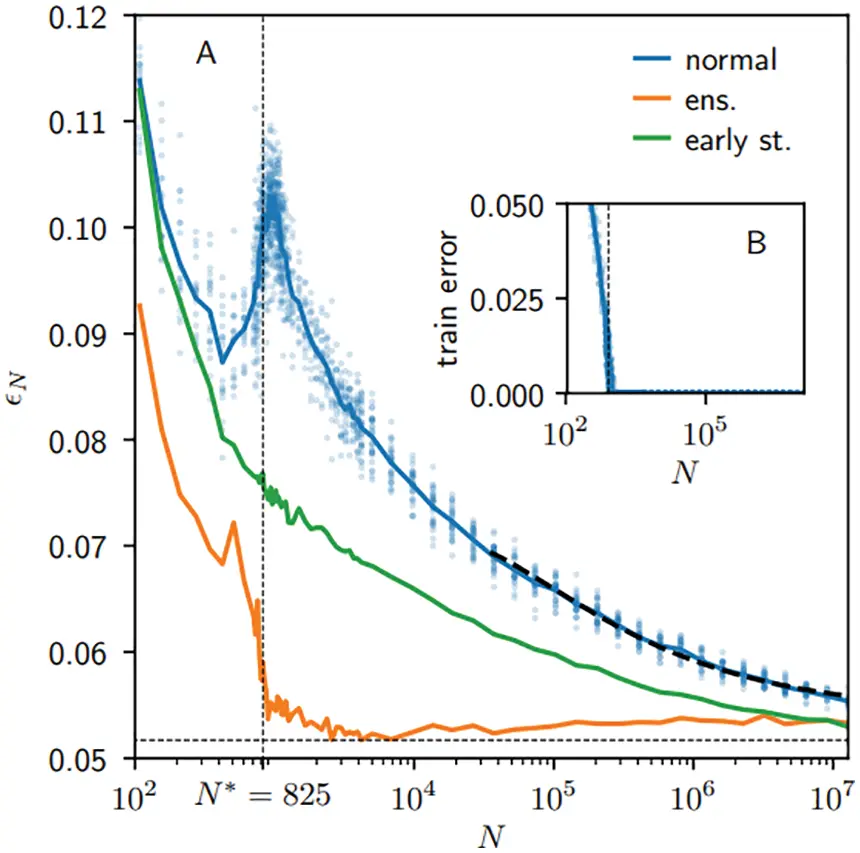Figure 61: Empirical test error vs Number of paramesters (Sections 6.1, 14.2). Experiments using the MNIST handwritten digit database in  confirmed the modern interpolation regime in Figure 60 . Blue: Average over 20 runs. Green: Early stopping. Orange: Ensemble average on n=20 samples, trained independently. See Figure 148 for a shallow network with infinite width. (Figure reproduced with permission of the authors.)

θ()θ()ϵJ/θ()=θ()ϵg() with g():=J/θ()Rm()×[m(1)+1].(119)

being the gradient direction, and ϵ called the learning rate.114 The layer-by-layer update in Eq. (119) as soon as the gradient g()=J/θ() had been computed is valid when the learning rate ϵ does not depend on the gradient g=J/θ with respect to the whole set of network parameters θ.

Otherwise, the update of the whole network parameter θ would be carried out after the complete gradient g=J/θ had been obtained, and the learning rate ϵ had been computed based on the gradient g:

θθϵJ/θ=θϵg, with g:=J/θRPT×1,(120)

where PT is the total number of network paramters defined in Eq. (34). An example of a learning-rate computation that depends on the complete gradient g=J/θ is gradient descent with Armijo line search; see Section 6.2.3 and line 8 in Algorithm 1.

“Neural network researchers have long realized that the learning rate is reliably one of the most difficult to set hyperparameters because it significantly affects model performance.” , p. 298.

In fact, it is well known in the field of optimization, where the learning rate is often mnemonically denoted by λ, being Greek for “l” and standing for “step length”; see, e.g., Polak (1971) .

“We can choose ϵ in several different ways. A popular approach is to set ϵ to a small constant. Sometimes, we can solve for the step size that makes the directional derivative vanish. Another approach is to evaluate f(xϵxf(x)) for several values of ϵ and choose the one that results in the smallest objective function value. This last strategy is called a line search.” , p. 82.

Choosing an arbitrarily small ϵ, without guidance on how small is small, is not a good approach, since exceedingly slow convergence could result for too small ϵ. In general, it would not be possible to solve for the step size to make the directional derivative vanish. There are several variants of line search for computing the step size to decrease the cost function, based on the “decrease” conditions,115 among which some are mentioned below.116

Remark 6.4. Line search in deep-learning training. Line search methods are not only important for use in deterministic optimization with full batch of examples,117 but also in stochastic optimization (see Section 6.3) with random mini-batches of examples . The difficulty of using stochastic gradient coming from random mini-batches is the presence of noise or “discontinuities”118 in the cost function and in the gradient. Recent stochastic optimization methods—such as the sub-sampled Hessian-free Newton method reviewed in , the probabilisitic line search in , the first-order stochastic Armijo line search in , the second-order sub-sampling line search method in , quasi-Newton method with probabilitic line search in , etc.—where line search forms a key subprocedure, are designed to address or circumvent the noisy gradient problem. For this reason, claims that line search methods have “fallen out of favor”119 would be misleading, as they may encourage students not to learn the classics. A classic never dies; it just re-emerges in a different form with additional developments to tackle new problems.    ■

In view of Remark 6.4, a goal of this section is to develop a feel for some classical deterministic line search methods for readers not familiar with these concepts to prepare for reading extensions of these methods to stochastic line search methods.

6.2.1 Exact line search

Find a positive step length ϵ that minimizes the cost function J along the descent direction d such that the scalar (dot) product between g and dRPT×1:

<g,d>=gd=ijgijdij<0(121)

is negative, i.e., the descent direction d and the gradient g form an obtuse angle bounded away from 90°,120 and

J(θ+ϵd)=minλ{J(θ+λd)|λ0}.(122)

The minimization problem in Eq. (122) can be implemented using the Golden section search (or infinite Fibonacci search) for unimodal functions.121 For more general non-convex cost functions, a minimizing step length may be non-existent, or difficult to compute exactly.122 In addition, a line search for a minimizing step length is only an auxilliary step in an overall optimization algorithm. It is therefore sufficient to find an approximate step length satisfying some decrease conditions to ensure convergence to a local minimum, while keeping the step length from being too small that would hinder a reasonable advance toward such local minimum. For these reasons, inexact line search methods (rules) were introduced, first in , followed by , then  and . In view of Remark 6.4 and Footnote 119, as we present these deterministic line-search rules, we will also immediately recall, where applicable, the recent references that generalize these rules by adding stochasticity for use as a subprocedure (inner loop) for the stochastic gradient-descent (SGD) algorithm.

6.2.2 Inexact line-search, Goldstein’s rule

The method is inexact since the search for an acceptable step length would stop before a minimum is reached, once the rule is satisfied.123 For a fixed constant α(0,12), select a learning rate (step length) ϵ such that124

1αJ(θ+ϵd)J(θ)ϵgdα(123)

where both the numerator and the denominator are negative, i.e., J(θ+ϵd)J(θ)<0 and gd<0 by Eq. (121). Eq. (123) can be recast into a slightly more general form: For 0<α<β<1, choose a learning rate ϵ such that125

βϵgdΔJ(ϵ)αϵgd with ΔJ(ϵ):=J(θ+ϵd)J(θ).(124)

A reason could be that the sector bounded by the two lines (1α)ϵgd and αϵgd may be too narrow when α is close to 0.5 from below, making (1α) also close to 0.5 from above. For example, it was recommended in , p. 33 and p. 37, to use α=0.4, and hence 1α=0.6, making a tight sector, but we could enlarge such sector by choosing 0.6<β<1.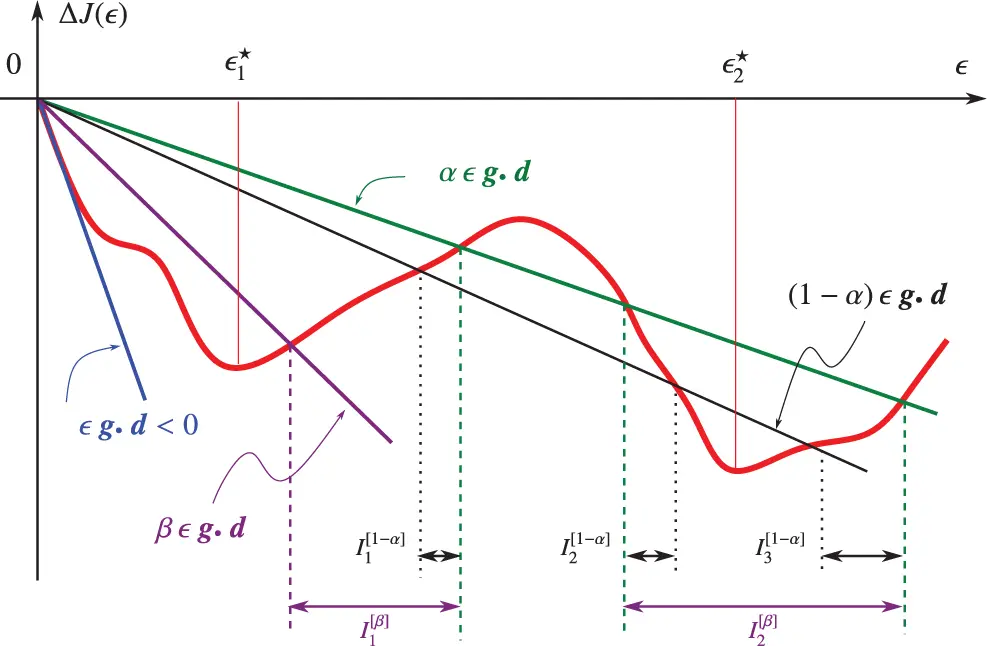Figure 62: Inexact line search, Goldstein’s rule (Section 6.2.4). acceptable step lengths would be such that a decrease in the cost function J, denoted by ΔJ in Eq. (124), falls into an acceptable sector formed by an upper-bound line and a lower-bound line. the upper bound is given by the straight line αϵgd (green), with fixed constant α(0,12) and ϵgd<0 being the slope to the curve ΔJ(ϵ) at ϵ=0. The lower-bound line (1α)ϵgd (black), adopted in  and , would be too narrow when α is close to 12, leaving all local minimizers such as ϵ1 and ϵ2 outside of the acceptable intevals I1[1α], I2[1α], and I3[1α] (black), which are themselves narrow. The lower-bound line βϵgd (purple) proposed in , p. 256, and , p. 55, with (1α)<β<1, would enlarge the acceptable sector, which then may contain the minimizers inside the corresponding acceptable intervals I1[β] and I2[β] (purple).

The search for an appropriate step length that satisfies Eq. (123) or Eq. (124) could be carried out by a subprocedure based on, e.g., the bisection method, as suggested in , p. 33. Goldstein’rule—also designated as Goldstein principle in the classic book , p. 256, since it ensured a decrease in the cost function—has been “used only occasionally” per Polak (1997) , p. 55, largely superceded by Armijo’s rule, and has not been generalized to add stochasticity. On the other hand, the idea behind Armijo’s rule is similar to Goldstein’s rule, but with a convenient subprocedure126 to find the appropriate step length.

6.2.3 Inexact line-search, Armijo’s rule

Apparently without the knowledge of , it was proposed in  the following highly popular Armijo step-length search,127 which recently forms the basis for stochastic line search for use in stochastic gradient-descent algorithm described in Section 6.3: Stochasticity was added to Armijo’s rule in , and the concept was extended to second-order line search . Line search based on Armijo’s rule is also applied to quasi-Newton method for noisy functions in , and to exact and inexact subsampled Newton methods in .128

Armijo’s rule is stated as follows: For α(0,1), β(0,1), and ρ>0, use the step length ϵ such that:129

ϵ(θ)=mina{βaρ|J(θ+βjd)J(θ)αβaρgd}(125)

where the decrease in the cost function along the descent direction d, denoted by ΔJ, was defined in Eq. (124), and the descent direction d is related to the gradient g via Eq. (121). The Armijo condition in Eq. (125) can be rewritten as

J(θ+ϵd)J(θ)+αϵgd,(126)

which is also known as the Armijo sufficient decrease condition, the first of the two Wolfe conditions presented below; see , , p. 55.130

Regarding the paramters α, β, and ρ in the Armijo’s rule Eq. (125),  selected to fix

α=β=12, and ρ(0,+),(127)

and proved a convergence theorem. In practice, ρ cannot be arbitrarily large. Polak (1971) , p. 36, also fixed α=12, but recommended to select β(0.5,0.8), based on numerical experiments.131, and to select132 ρ=1 to minimize the rate r of geometric progression (from the iterate θi, for i=0,1,2,, toward the local minimizer θ) for linear convergence:133

|J(θi+1)J(θ)|ri|J(θ0)J(θ)|, with r=1(ρmM)2,(128)

where m and M are the lower and upper bounds of the eigenvalues134 of the Hessian 2J, thus mM<1. In summary,  recommended:

α=12, β(0.5,0.8), and ρ=1.(129)

The pseudocode for deterministic gradient descent with Armijo line search is Algorithm 2, and the pseudocode for deterministic quasi-Newton / Newton with Armijo line search is Algorithm 3. When the Hessian H(θ)=2J(θ)/(θ)2 is positive definite, the Newton descent direction is:

d=H1(θ)g,(130)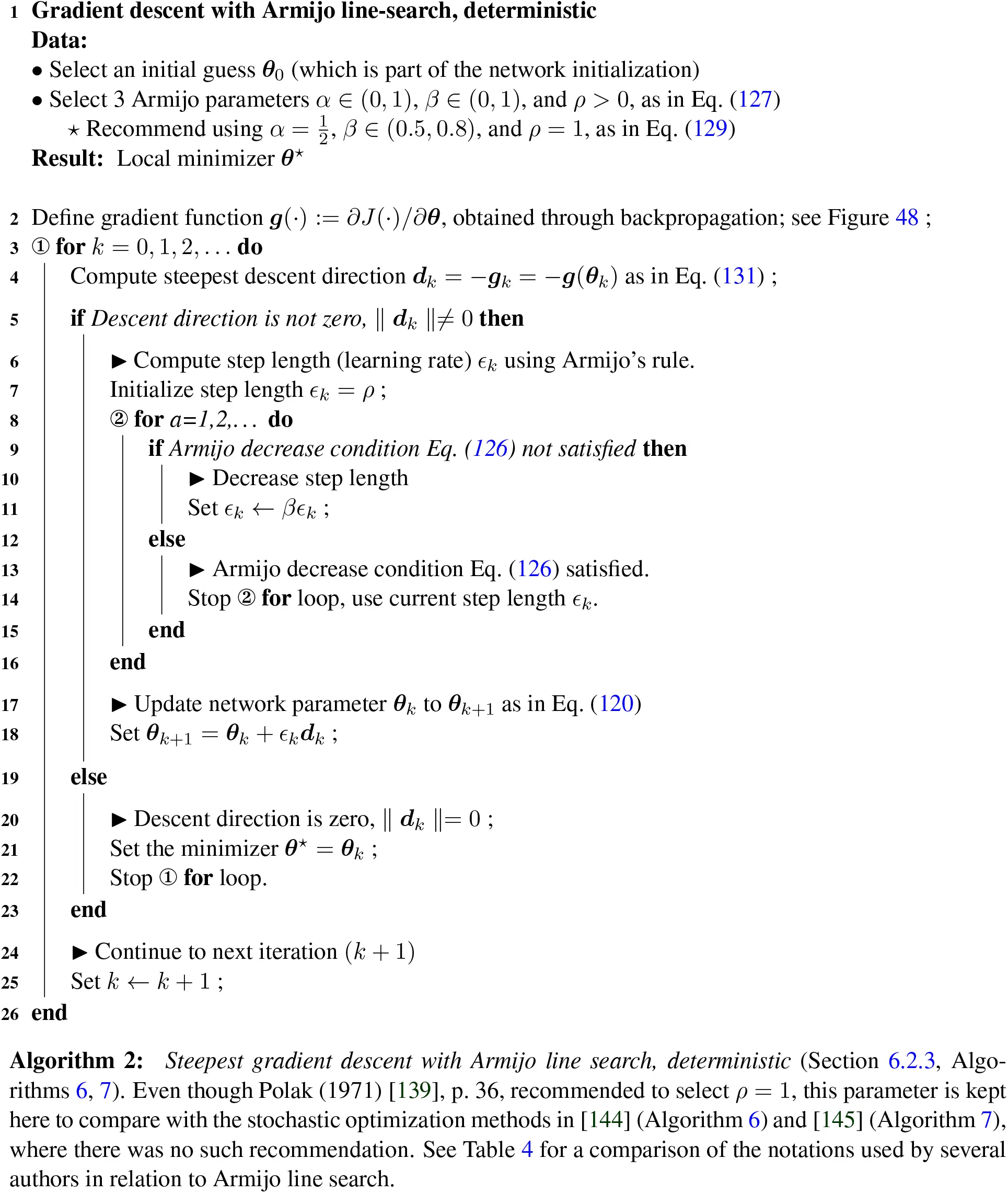When the Hessian H(θ) is not positive definite, e.g., near a saddle point, then quasi-Newton method uses the gradient descent direction (g=J/θ) as the descent direction d as in Eq. (120),

d=g=J(θ)/θ,(131)

and regularized Newton method uses a descent direction based on a regularized Hessian of the form:

d=[H(θ)+δI]1g,(132)

where δ is a small perturbation parameter (line 15 in Algorithm 3 for deterministic Newton and line 17 in Algorithm 7 for stochastic Newton).135

6.2.4 Inexact line-search, Wolfe’s rule

The rule introduced in  and ,136 sometimes called the Armijo-Goldstein-Wolfe’s rule (or conditions), particularly in  and ,137 has been extended to add stochasticity ,138 is stated as follows: For 0<α<β<1, select the step length (learning rate) ϵ such that (see, e.g., , p. 55):

J(θ+ϵd)J(θ)+αϵgd(133)

J(θ+ϵd)θdβgd.(134)

The first Wolfe’s rule in Eq. (133) is the same as the Armijo’s rule in Eq. (126), which ensures that at the updated point (θ+ϵd) the cost function value J(θ+ϵd) is below the green line αϵgd in Figure 62.

The second Wolfe’s rule in Eq. (134) is to ensure that at the updated point (θ+ϵd) the slope of the cost function cannot fall below the (negative) slope of the purple line βϵgd in Figure 62.

For other variants of line search, we refer to .

To avoid confusion,139 we will use the terminology “full batch” (instead of just “batch”) when the entire training set is used for training. a minibatch is a small subset of the training set.

In fact, as we shall see, and as mentioned in Remark 6.4, classical optimization methods mentioned in Section 6.2 have been developed further to tackle new problems, such as noisy gradients, encountered in deep-learning training with random mini-batches. There is indeed much room for new research on learning rate since:

“The learning rate may be chosen by trial and error. This is more of an art than a science, and most guidance on this subject should be regarded with some skepticism.” , p. 287.

At the time of this writing, we are aware of two review papers on optimization algorithms for machine learning, and in particular deep learning, aiming particularly at experts in the field: , as mentioned above, and . Our review complements these two review papers. We are aiming here at bringing first-time learners up to speed to benefit from, and even to hopefully enjoy, reading these and others related papers. To this end, we deliberately avoid the dense mathematical-programming language, not familiar to readers outside the field, as used in , while providing more details on algorithms that have proved important in deep learning than .

Listed below are the points that distinguish the present paper from other reviews. Similar to , both  and :

•   Only mentioned briefly in words the connection between SGD with momentum to mechanics without detailed explanation using the equation of motion of the “heavy ball”, a name not as accurate as the original name “small heavy sphere” by Polyak (1964) . These references also did not explain how such motion help to accelerate convergence; see Section 6.3.2.

•   Did not discuss recent practical add-on improvements to SGD such as step-length tuning (Section 6.3.3) and step-length decay (Section 6.3.4), as proposed in . This information would be useful for first-time learners.

•   Did not connect step-length decay to simulated annealing, and did not explain the reason for using the name “annealing”140 in deep learning by connecting to stochastic differential equation and physics; see Remark 6.9 in Section 6.3.5.

•   Did not review an alternative to step-length decay by increasing minibatch size, which could be more efficient, as proposed in ; see Section 6.3.5.

•   Did not point out that the exponential smoothing method (or running average) used in adaptive learning-rate algorithms dated since the 1950s in the field of forecasting. None of these references acknowledged the contributions made in  and , in which exponential smoothing from time series in forecasting was probably first brought to machine learning. See Section 6.5.3.

•   Did not discuss recent adaptive learning-rate algorithms such as AdamW .141 These authors also did not discuss the criticism of adaptive methods in ; see Section 6.5.10.

•   Did not discuss classical line-search rules—such as , ,142  (Sections 6.2.2, 6.2.3, 6.2.4)—that have been recently generalized to add stochasticity, e.g., , , ; see Sections 6.6, 6.7.

6.3.1 Standard SGD, minibatch, fixed learning-rate schedule

The stochastic gradient descent algorithm, originally introduced by Robbins & Monro (1951a)  (another classic) according to many sources,143 has been playing an important role in training deep-learning networks:

“Nearly all of deep learning is powered by one very important algorithm: stochastic gradient descent (SGD). Stochastic gradient descent is an extension of the gradient descent algorithm.” , p. 147.

Minibatch. The number 𝖬 of examples in a training set 𝕩 could be very large, rendering prohibitively expensive to evaluate the cost function and to compute the gradient of the cost function with respect to the number of parameters, which by itself could also be very large. At iteration k within a training session τ, let 𝕀k|𝗆| be a randomly selected set of 𝗆 indices, which are elements of the training-set indices [𝖬]={1,,𝖬}. Typically, 𝗆 is much smaller than 𝖬:144

“The minibatch size 𝗆 is typically chosen to be a relatively small number of examples, ranging from one to a few hundred. Crucially, 𝗆 is usually held fixed as the training set size 𝖬 grows. We may fit a training set with billions of examples using updates computed on only a hundred examples.” , p. 148.

Generated as in Eq. (136), the random-index sets 𝕀k|𝗆|, for k=1,2,, are non-overlapping such that after kmax=𝖬/𝗆 iterations, all examples in the training set are covered, and a training session, or training epoch,145 is completed (line 6 in Algorithm 4). At iteration k of a training epoch τ, the random minibatch 𝔹k|𝗆| is a set of 𝗆 examples pulled out from the much larger training set 𝕩 using the random indices in 𝕀k|𝗆|, with the corresponding targets in the set 𝕋k|𝗆|:

M1={1,,𝖬}=:[𝖬], kmax=𝖬/𝗆(135)

Ik|𝗆|={i1,k,,i𝗆,k}Mk,, Mk+1=MkIk|𝗆| for k=1,,kmax(136)

Bk|𝗆|={x|i1,k|,,x|i𝗆,k|}X={x|1|,,x|𝖬|}(137)

Tk|𝗆|={y|i1,k|,,y|i𝗆,k|}Y={y|1|,,y|𝖬|}(138)

Note that once the random index set 𝕀k|𝗆| had been selected, it was deleted from its superset Mk to form Mk+1 so the next random set 𝕀j+1|𝗆| would not contain indices already selected in 𝕀k|𝗆|.

Unlike the iteration counter k within a training epoch τ, the global iteration counter j is not reset to 1 at the beginning of a new training epoch τ+1, but continues to increment for each new minibatch. Plots versus epoch counter τ and plots versus global iteration counter j could be confusing; see Remark 6.17 and Figure 78.

Cost and gradient estimates. The cost-function estimate is the average of the cost functions, each of which is the cost function of an example x|ik| in the minibatch for iteration k in training epoch τ:

J~(θ)=1𝗆a=1a=𝗆𝖬Jia(θ), with Jia(θ)=J(f(x|ia|,θ),y|ia|), and x|ia|Bk|𝗆|,,y|ia|Tk|𝗆|,(139)

where we wrote the random index as ia instead of ia,k as in Eq. (136) to alleviate the notation. The corresponding gradient estimate is:

g~(θ)=J~(θ)θ=1𝗆a=1a=𝗆𝖬Jia(θ)θ=1𝗆a=1a=𝗆𝖬gia.(140)

The pseudocode for the standard SGD146 is given in Algorithm 4. The epoch stopping criterion (line 1 in Algorithm 4) is usually determined by a computation “budget”, i.e., the maximum number of epochs allowed. For example,  set a budget of 1,600 epochs maximum in their numerical examples.

Problems and resurgence of SGD. There are several known problems with SGD:

“Despite the prevalent use of SGD, it has known challenges and inefficiencies. First, the direction may not represent a descent direction, and second, the method is sensitive to the step-size (learning rate) which is often poorly overestimated.” 

For the above reasons, it may not be appropriate to use the norm of the gradient estimate being small as stationarity condition, i.e., where the local minimizer or saddle point is located; see the discussion in  and stochastic Newton Algorithm 7 in Section 6.7.

Despite the above problems, SGD has been brought back to the forefront state-of-the-art algorithm to beat, surpassing the performance of adaptive methods, as confirmed by three recent papers: , , ; see Section 6.5.9 on criticism of adaptive methods.

Add-on tricks to improve SGD. The following tricks can be added onto the vanilla (standard) SGD to improve its performance; see also the pseudocode in Algorithm 4:

•   Momentum and accelerated gradient: Improve (accelerate) convergence in narrow valleys, Section 6.3.2

•   Initial-step-length tuning: Find effective initial step length ϵ0, Section 6.3.3

•   Step-length decaying or annealing: Find an effective learning-rate schedule147 to decrease the step length ϵ as a function of epoch counter τ or global iteration counter j, cyclic annealing, Section 6.3.4

•   Minibatch-size increase, keeping step length fixed, equivalent annealing, Section 6.3.5

•   Weight decay, Section 6.3.6

6.3.2 Momentum and fast (accelerated) gradient

The standard update for gradient descent is Eq. (120) would be slow when encountering deep and narrow valley, as shown in Figure 63, and can be replaced by the general update with momentum as follows:

θ~k+1=θ~kϵkg~(θ~k+γk(θ~kθ~k1))+ζk(θ~kθ~k1)(141)

from which the following methods are obtained (line 10 in Algorithm 4):

•   Standard SGD update Eq. (120) with γk=ζk=0 

•   SGD with classical momentum: γk=0 and ζk(0,1) (“small heavy sphere” or heavy point mass)148 

•   SGD with fast (accelerated) gradient:149 γk=ζk(0,1), Nesterov (1983 , 2018 )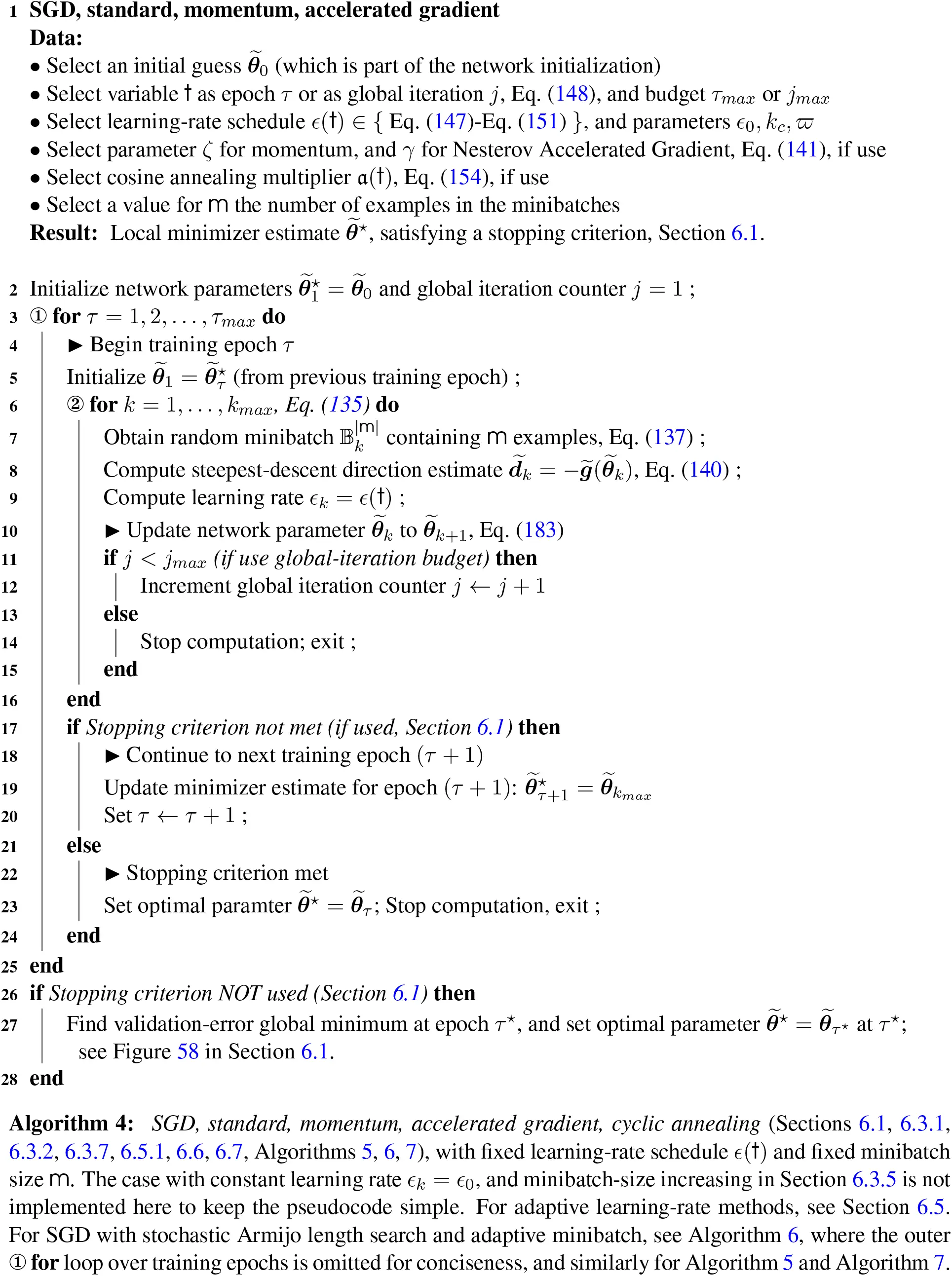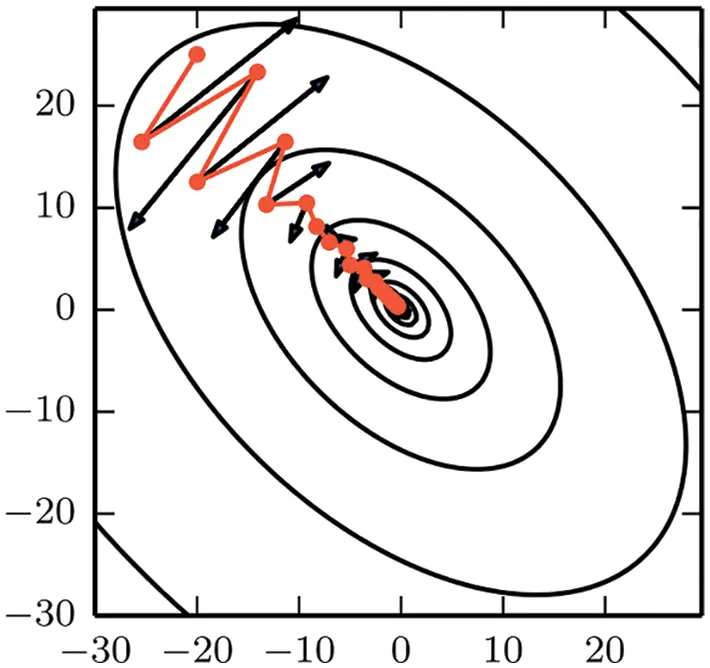Figure 63: SGD with momentum, small heavy sphere Section 6.3.2. The descent direction (negative gradient, black arrows) bounces back and forth between the steep slopes of a deep and narrow valley. The small-heavy-sphere method, or SGD with momentum, follows a faster descent (red path) toward the bottom of the valley. See the cost-function landscape with deep valleys in Figure 55. Figure from , p. 289. (Figure reproduced with permission of the authors.)

The continuous counterpart of the parameter update Eq. (141) with classical momentum, i.e., when γk(0,1) and ζk=0, is the equation of motion of a heavy point mass (thus no rotatory inertia) under viscous friction at slow motion (proportional to velocity) and applied force g~ given below with its discretization by finite difference in time, where hk and hk1 are the time-step sizes :

d2θ~(dt)2+νdθ~dt=g~θ~k+1θ~khkθ~kθ~k1hk1hk+νθ~k+1θ~khk=g~k,(142)

θ~k+1θ~kζk(θ~kθ~k1)=ϵkg~k, with ζk=hk1hk11+νhk and ϵk=(hk)211+νhk,(143)

which is the same as the update Eq. (141) with γk=0. The term ζk(θkθk1) is often called the “momentum” term since it is proportional to (discretized) velocity.  on the other hand explained the term ζk(θkθk1) as “giving inertia to the motion, [leading] to motion along the “essential” direction, i.e. along ‘the bottom of the trough’ ”, and recommended to select ζk(0.8,0.99), i.e., close to 1, without explanation. The reason is to have low friction, i.e., ν small, but not zero friction (ν=0), since friction is important to slow down the motion of the sphere up and down the valley sides (like skateboarding from side to side in a half-pipe), thus accelerate convergence toward the trough of the valley; from Eq. (143), we have

hk=hk1=h and ν[0,+)ζk(0,1], with ν=0ζk=1(144)

Remark 6.5. The choice of the momentum parameter ζ in Eq. (141) is not trivial. If ζ is too small, the signal will be too noisy; if ζ is too large, “the average will lag too far behind the (drifting) signal” , p. 212. Even though Polyak (1964)  recommended to select ζ(0.8,0.99), as explained above, it was reported in , p. 290: “Common values of ζ used in practice include 0.5, 0.9, and 0.99. Like the learning rate, ζ may also be adapted over time. Typically it begins with a small value and is later raised. Adapting ζ over time is less important than shrinking ϵ over time”. The value of ζ=0.5 would correspond to relatively high friction μ, slowing down the motion of the sphere, compared to ζ=0.99.

In their remarkable paper, the authors of  used a constant momentum parameter ζ=0.9; see criticism of adaptive methods in Section 6.5 and Figure 73 comparing SGD, SGD with momentum, AdaGrad, RMSProp, Adam.150

See Figure 151 in Section 14.7 on “Lack of transparency and irreproducibility of results” in recent deep-learning papers.    ■

For more insight into the update Eq. (143), consider the case of constant coefficients ζk=ζ and ϵk=ϵ, and rewrite this recursive relation in the form:

θ~k+1θ~k=ϵi=0kζig~ki, using θ~1θ~0=ϵg~0,(145)

i.e., without momentum for the first term. So the effective gradient is the sum of all gradients from the beginning i=0 until the present i=k weighted by the exponential function ζi so there is a fading memory effect, i.e., gradients that are farther back in time have less influence than those closer to the present time.151 The summation term in Eq. (145) also provides an explanation of how the “inertia” (or momentum) term work: (1) Two successive opposite gradients would cancel each other, whereas (2) Two successive gradients in the same direction (toward the trough of the valley) would reinforce each other. See also , pp. 104-105, and  who provided a similar explanation:

“Momentum is a simple method for increasing the speed of learning when the objective function contains long, narrow and fairly straight ravines with a gentle but consistent gradient along the floor of the ravine and much steeper gradients up the sides of the ravine. The momentum method simulates a heavy ball rolling down a surface. The ball builds up velocity along the floor of the ravine, but not across the ravine because the opposing gradients on opposite sides of the ravine cancel each other out over time.”

In recent years, Polyak (1964)  (English version)152 has often been cited for the classical momentum (“small heavy sphere”) method to accelerate the convergence in gradient descent, but not so before, e.g., the authors of      used the same method without citing . Several books on optimization not related to neural networks, many of them well-known, also did not mention this method:      . Both the original Russian version and the English translated version  (whose author’s name was spelled as “Poljak” before 1990) were cited in the book on neural networks , in which another neural-network book  was referred to for a discussion of the formulation.153

Remark 6.6. Small heavy sphere, or heavy point mass, is better name. Because the rotatory motion is not considered in Eq. (142), the name “small heavy sphere” given in  is more precise than the more colloquial name “heavy ball” often given to the SGD with classical momentum,154 since “small” implies that rotatory motion was neglected, and a “heavy ball” could be as big as a bowling ball155 for which rotatory motion cannot be neglected. For this reason, “heavy point mass” would be a precise alternative name.    ■

Remark 6.7. For Nesterov’s fast (accelerated) gradient method, many references referred to .156 The authors of , p. 291, also referred to Nesterov’s 2004 monograph, which was mentioned in the Preface of, and the material of which was included in, . For a special class of strongly convex functions,157 the step length can be kept constant, while the coefficients in Nesterov’s fast gradient method varied, to achieve optimal performance, , p. 92. “Unfortunately, in the stochastic gradient case, Nesterov momentum does not improve the rate of convergence” , p. 292.    ■

6.3.3 Initial-step-length tuning

The initial step length ϵ0, or learning-rate initial value, is one of the two most influential hyperparameters to tune, i.e., to find the best performing values. During tuning, the step length ϵ is kept constant at ϵ0 in the parameter update Eq. (120) throughout the optimization process, i.e., a fixed step length is used, without decay as in Eqs. (147-150) in Section 6.3.4.

The following simple tuning method was proposed in :

“To tune the step sizes, we evaluated a logarithmically-spaced grid of five step sizes. If the best performance was ever at one of the extremes of the grid, we would try new grid points so that the best performance was contained in the middle of the parameters. For example, if we initially tried step sizes 2, 1, 0.5, 0.25, and 0.125 and found that 2 was the best performing, we would have tried the step size 4 to see if performance was improved. If performance improved, we would have tried 8 and so on.”

The above logarithmically-spaced grid was given by 2k, with k=1,0,1,2,3. This tuning method appears effective as shown in Figure 73 on the CIFAR-10 dataset mentioned above, for which the following values for ϵ0 had been tried for different optimizers, even though the values did not always belong to the sequence {ak}, but could include close, rounded values:

•   SGD (Section 6.3.1): 2, 1, 0.5 (best), 0.25, 0.05, 0.01158

•   SGD with momentum (Section 6.3.2): 2, 1, 0.5 (best), 0.25, 0.05, 0.01

•   RMSProp (Section 6.5): 0.005, 0.001, 0.0005, 0.0003 (best), 0.0001

•   Adam (Section 6.5): 0.005, 0.001 (default), 0.0005, 0.0003 (best), 0.0001, 0.00005

6.3.4 Step-length decay, annealing and cyclic annealing

In the update of the parameter θ as in Eq. (120), the learning rate (step length) ϵ has to be reduced gradually as a function of either the epoch counter τ or of the global iteration counter j. Let represents either τ or j, depending on user’s choice.159 If the learning rate ϵ is a function of epoch τ, then ϵ is held constant in all iterations k=1,,kmax within epoch τ, and we have the relation:

j=(τ1)kmax+k.(146)

The following learning-rate scheduling, linear with respect to , is one option:160

ϵ()={(1c)ϵ0+cϵc for 0cϵc for c(147)

=epoch τ or global iteration j(148)

where ϵ0 is the learning-rate initial value, and ϵc the constant learning-rate value when c. Other possible learning-rate schedules are:161

ϵ()=ϵ00 as ,(149)

ϵ()=ϵ00 as ,(150)

with defined as in Eq. (147), even though authors such as  and  used Eq. (149) and Eq. (150) with =j as global iteration counter.

Another step-length decay method proposed in  is to reduce the step length ϵ(τ) for the current epoch τ by a factor ϖ(0,1) when the cost estimate J˜τ1 at the end of the last epoch (τ1) is greater than the lowest cost in all previous global iterations, with J˜j denoting the cost estimate at global iteration j, and kmax(τ1) the global iteration number at the end of epoch (τ1):

ϵ(τ)={ϖϵ(τ1) if J~τ1>minj{J~j,j=1,,kmax(τ1)}ϵ(τ1) Otherwise(151)

Recall, kmax is the number of non-overlapping minibatches that cover the training set, as defined in Eq. (135).  set the step-length decay parameter ϖ=0.9 in their numerical examples, in particular Figure 73.

Cyclic annealing. In additional to decaying the step length ϵ, which is already annealing, cyclic annealing is introduced to further reduce the step length down to zero (“cooling”), quicker than decaying, then bring the step length back up rapidly (heating), and doing so for several cycles. The cosine function is typically used, such as shown in Figure 75, as a multiplicative factor 𝔞k[0,1] to the step length ϵk in the parameter update, and thus the name “cosine annealing”:

θ~k+1=θ~kakϵkg~k,(152)

as an add-on to the parameter update for vanilla SGD Eq. (120), or

θ~k+1=θ~kakϵkg~(θ~k+γk(θ~kθ~k1))+ζk(θ~kθ~k1)(153)

as an add-on to the parameter update for SGD with momentum and accelerated gradient Eq. (141). The cosine annealing factor can take the form :

ak=0.5+0.5cos(πTcur/Tp)[0,1], with Tcur:=jq=1q=p1Tq(154)

where Tcur is the number of epochs from the start of the last warm restart at the end of epoch q=1q=p1Tq, where 𝔞k=1 (“maximum heating”), j the current global iteration counter, Tp the maximum number of epochs allowed for the current pth annealing cycle, during which Tcur would go from 0 to Tp, when 𝔞k=0 (“complete cooling”). Figure 75 shows 4 annealing cycles, which helped reduce dramatically the number of epochs needed to achieve the same lower cost as obtained without annealing.

Figure 74 shows the effectiveness of cosine annealing in bringing down the cost rapidly in the early stage, but there is a diminishing return, as the cost reduction decreases with the number of annealing cycle. Up to a point, it is no longer as effective as SGD with weight decay in Section 6.3.6.

Convergence conditions. The sufficient conditions for convergence, for convex functions, are162

j=1ϵj2<, and j=1ϵj=.(155)

The inequality on the left of Eq. (155), i.e., the sum of the squared of the step lengths being finite, ensures that the step length would decay quickly to reach the minimum, but is valid only when the minibatch size is fixed. The equation on the right of Eq. (155) ensures convergence, no matter how far the initial guess was from the minimum .

In Section 6.3.5, the step-length decay is shown to be equivalent to minibatch-size increase and simulated annealing in the sense that there would be less fluctuation, and thus lower “temperature” (cooling) by analogy to the physics governed by the Langevin stochastic differential equation and its discrete version, which is analogous to the network parameter update.

6.3.5 Minibatch-size increase, fixed step length, equivalent annealing

The minibatch parameter update from Eq. (141), without momentum and accelerated gradient, which becomes Eq. (120), can be rewritten to introduce the error due to the use of the minibatch gradient estimate g˜ instead of the full-batch gradient g as follows:

θ˜k+1= θ˜kϵk g˜k= θ˜kϵk[ gk+( g˜kgk) ]Δ θ˜kϵk=θ˜k+1 θ˜kϵk=gk+(gk g˜k)=gk+ek  (156)

where gk=g(θk) and g˜k=g~b(θk), with b=k, and g˜b() is the gradient estimate function using minibatch b=1,,kmax.

To show that the gradient error has zero mean (average), based on the linearity of the expectation function 𝔼()= defined in Eq. (67) (Footnote 89), i.e.,

αu+βv=αu+βu,(157)

ek=gkg~k=gkg~k=gkg~k,(158)

from Eqs. (135)-(137) on the definition of minibatches and Eqs. (139)-(140) on the definition of the cost and gradient estimates (without omitting the iteration counter k), we have

gk=g(θk)=1kmaxb=1b=kmax1𝗆a=1a=𝗆gia,bgk=gk=1kmaxb=1b=kmax1𝗆a=1a=𝗆gia,b=gia,b(159)

g~k=1𝗆a=1a=𝗆𝖬gia,kg~k=1kmaxk=1k=kmaxgia,k=1kmaxk=1k=kmaxgk=gkek=0.(160)

Or alternatively, the same result can be obtained with:

gk=1kmaxb=1b=kmaxg~b(θk)gk=g~b(θk)=g~k(θk)=g~kek=0.(161)

Next, the mean value of the “square” of the gradient error, i.e., eTe, in which we omitted the iteration counter subscript k to alleviate the notation, relies on some identities related to the covariance matrix e,e. The mean of the square matrix xiTxj, where {xixj} are two random row matrices, is the sum of the product of the mean values and the covariance matrix of these matrices163

xiTxj=xiTxj+xi,xj or xi,xj=xiTxjxiTxj(162)

where xi,xj is the covariance matrix of xi and xj, and thus the covariance operator , is bilinear due to the linearity of the mean (expectation) operator in Eq. (157):

iαiui,jβjvj=iαiβjui,vj,αi,βjR and ui,vjRn random.(163)

Eq. (163) is the key relation to derive an expression for the square of the gradient error eTe, which can be rewritten as the sum of four covariance matrices upon using Eq. (162)1 and either Eq. (160) or Eq. (161), i.e., g~k=gk=gk, as the four terms gkTgk cancel each other out:

eTe=(g~g)T(g~g)=g~,g~g~,gg,g~+g,g,(164)

where the iteration counter k had been omitted to alleviate the notation. Moreover, to simplify the notation further, the gradient related to an example is simply denoted by ga or gb, with a,b=1,,𝗆 for a minibatch, and a,b=1,,𝖬 for the full batch:

g~=g~k=1𝗆a=1𝗆gia,k=1𝗆a=1𝗆gia=1𝗆a=1𝗆ga,(165)

g=1kmaxk=1kmax1𝗆a=1𝗆gia,k=1𝖬b=1𝖬gb.(166)

Now assume the covariance matrix of any pair of single-example gradients ga and gb depends only on the parameters θ, and is of the form:

ga,gb=C(θ)δab,a,b{1,,𝖬},(167)

where δab is the Kronecker delta. Using Eqs. (165)-(166) and Eq. (167) in Eq. (164), we obtain a simple expression for eTe:164

eTe=(1𝗆1𝖬)C.(168)

The authors of  introduced the following stochastic differential equation as a continuous counterpart of the discrete parameter update Eq. (156), as ϵk0:

dθdt=g+n(t)=dJdθ+n(t),(169)

where 𝔫(t) is the noise function, the continuous counterpart of the gradient error 𝔢k:=(gkg~k). The noise 𝔫(t) is assumed to be Gaussian, i.e., with zero expectation (mean) and with covariance function of the form (see Remark 6.9 on Langevin stochastic differential equation):

n(t)=0,  andn(t)n(t)= 𝓕(θ)δ(tt]),(170)

where 𝔼[]= is the expectation of a function, 𝓕 the “noise scale” or fluctuation factor, C(θ) the same gradient-error covariance matrix in Eq. (167), and δ(tt) the Dirac delta. Integrating Eq. (169), we obtain:

t=0t=ϵkdθkdtdt=θk+1θk=ϵkgk+t=0t=ϵkn(t)dt, and t=0t=ϵkn(t)dt=t=0t=ϵkn(t)dt=0.(171)

The fluctuation factor 𝓕 can be identified by equating the square of the error in Eq. (156) to that in Eq. (171), i.e.,

ϵ2eTe=t=0t=ϵt=0t=ϵn(t)n(t)dtdtϵ2(1𝗆1𝖬)=ϵ𝓕 𝓕=ϵ(1𝗆1𝖬)(172)

Remark 6.8. Fluctuation factor for large training set. For large 𝖬, our fluctuation factor 𝓕 is roughly proportional to the ratio of the step length over the minibatch size, i.e., 𝓕ϵ/𝗆. Thus step-length ϵ decay, or equivalenly minibatch size 𝗆 increase, corresponds to a decrease in the fluctuation factor 𝓕. On the other hand,  obtained their fluctuation factor 𝓖 as165

𝓖=ϵ(𝖬𝗆1)=ϵ𝖬(1𝗆1𝖬)=𝖬𝓕(173)

since their cost function was not an average, i.e., not divided by the minibatch size 𝗆, unlike our cost function in Eq. (139). When 𝖬, our fluctuation factor 𝓕ϵ/𝗆 in Eq. (172), but their fluctuation factor 𝓖ϵ𝖬/𝗆, i.e., for increasingly large 𝖬, our fluctuation factor 𝓕 is bounded, but not their fluctuation factor 𝓖.  then went on to show empirically that their fluctation factor 𝓖 was proportional to the training-set size 𝖬 for large 𝖬, as shown in Figure 64. On the other hand, our fluctuation factor 𝓕 does not depend on the training set size 𝖬. As a result, unlike  in Figure 64, our optimal minibatch size would not depend of the training-set size 𝖬.    ■Figure 64 Optimal minibatch size vs. training-set size (Section 6.3.5). For a given trainingset size, the smallest minibatch size that achieves the highest accuracy is optimal. Left figure: The optimal mimibatch size was moving to the right with increasing training-set size M. Right figure: The optimal minibatch size in  is linearly proportional to the training-set size M for large training sets (i.e., M ← ∞), Eq. (173), but our fluctuation factor ℱ is independent of M when M ← ∞; see Remark 6.8. (Figure reproduced with permission of the authors.)

It was suggested in  to follow the same step-length decay schedules166 ϵ() in Section 6.3.4 to adjust the size of the minibatches, while keeping the step length constant at its initial value ϵ0. To demonstrate the equivalence between decreasing the step length and increasing minibatch size, the CIFAR-10 dataset with three different training schedules as shown in Figure 65 was used in .

The results are shown in Figure 66, where it was shown that the number of updates decreased drastically with minibatch-size increase, allowing for significantly shortening the training wall-clock time.

Remark 6.9. Langevin stochastic differential equation, annealing. Because the fluctuation factor 𝓕 was proportional to the step length, and in physics, fluctuation decreases with temperature (cooling), “decaying the learning rate (step length) is simulated annealing”168 . Here, we will connect the step length to “temperature” based on the analogy of Eq. (169), the continuous counterpart of the parameter update Eq. (156). In particular, we point exact references that justify the assumptions in Eq. (170).Figure 65: Minibatch-size increase vs. step-length decay, training schedules (Section 6.3.5). Left figure: Step length (learning rate) vs. number of epochs. Right figure: Minibatch size vs. number of epochs. Three learning-rate schedules167 were used for training: (1) The step length was decayed by a factor of 5, from an initial value of 101, at specific epochs (60, 120, 160), while the minibatch size was kept constant (blue line); (2) Hybrid, i.e., the step length was initially kept constant until epoch 120, then decreased by a factor of 5 at epoch 120, and by another factor of 5 at epoch 160 (green line); (3) The step length was kept constant, while the minibatch size was increased by a factor of 5, from an initial value of 128, at the same specific epochs, 60, 120, 160 (red line). See Figure 66 for the results using the CIFAR-10 dataset  (Figure reproduced with permission of the authors.)

Even though the authors of  referred to  for Eq. (169), the decomposition of the parameter update in :

θ~k+1=θ~kϵkgk+ϵkvk, with vk:=ϵk[gkg~k]=ϵkek(174)

with the intriguing factor ϵk was consistent with the equivalent expression in , p. 53, Eq. (3.5.10),169 which was obtained from the Fokker-Planck equation:

θ(t+Δt)=θ(t)+A(θ(t),t)Δ(t)+Δtη(t),(175)

where A(θ(t),t) is a nonlinear operator. The noise term Δtη(t) is not related to the gradient error as in Eq. (174), and is Gaussian with zero mean and covariance matrix of the form:

Δtη(t)=0, and Δtη(t),η(t)=ΔtB(t).(176)

The column matrix (or vector) A(θ(t),t) in Eq. (175) is called the drift vector, and the square matrix B in Eq. (176) the diffusion matrix, , p. 52. Eq. (175) implies that θ() is a continuous function, called the “sample path”.

To obtain a differential equation, Eq. (175) can be rewritten as

θ(t+Δt)θ(t)Δt=A(θ(t),t)+η(t)Δt,(177)

which shows that the derivative of θ(t) does not exist when taking the limit as Δt0, not only due to the factor 1/Δt, but also due to the noise η(t), , p. 53.

The last term η/Δt in Eq. (177) corresponds to the random force X exerted on a pollen particle by the viscous fluid molecules in the 1-D equation of motion of the pollen particle, as derived by Langevin and in his original notation, :170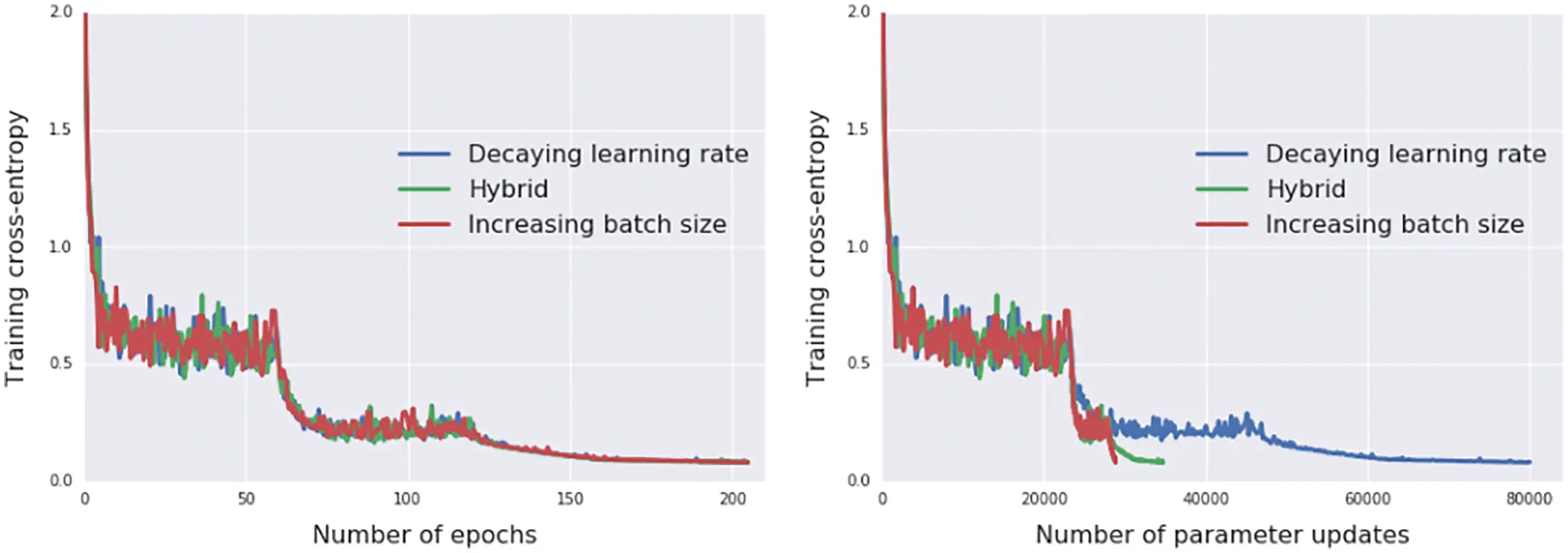Figure 66: Minibatch-size increase, fewer parameter updates, faster comutation (Section 6.3.5). For each of the three training schedules in Figure 65, the same learning curve is plotted in terms of the number of epochs (left figure), and again in terms of the number of parameter updates (right figure), which shows the significant decrease in the number of parameter updates, and thus computational cost, for the training schedule with minibatch-size decrease. The blue curve ends at about 80,000 parameter updates for step-length decrease, whereas the red curve ends at about 29,000 parameter updates for minibatch-size decrease  (Figure reproduced with permission of the authors.)

where m is the mass of the pollen particle, x(t) its displacement, μ the fluid viscosity, a the particle radius, v(t) the particle velocity, and 𝔣 the friction coefficient between the particle and the fluid. The random (noise) force X(t) by the fluid molecules impacting the pollen particle is assumed to (1) be independent of the position x, (2) vary extremely rapidly compared to the change of x, (3) have zero mean as in Eq. (176). The covariance of this noise force X is proportional to the absolute temperature T, and takes the form, , p. 12,171

X(t),X(t)=2fkTδ(tt),(179)

where k denotes the Boltzmann constant.

The covariance of the noise 𝔫(t) in Eq. (170) is similar to the covariance of the noise X(t) in Eq. (179), and thus the fluctuation factor 𝓕, and hence the step length ϵ in Eq. (172), can be interpreted as being proportional to temperature T. Therefore, decaying the step length ϵ, or increasing the minibatch size 𝗆, is equivalent to cooling down the temperature T, and simulating the physical annealing, and hence the name simulated annealing (see Remark 6.10).

Eq. (178) cannot be directly integrated to obtain the velocity v in terms of the noise force X since the derivative does not exist, as interpreted in Eq. (177). Langevin went around this problem by multiplying Eq. (178) by the displacement x(t) and take the average to obtain, :

m2dzdt=3πμaz+RTN(180)

where z=d(x2)¯/dt is the time derivative of the mean square displacement, R the ideal gas constant, and N the Avogadro number. Eq. (180) can be integrated to yield an expression for z, which led to Einstein’s result for Brownian motion.    ■

Remark 6.10. Metaheuristics and nature-inspired optimization algorithms. There is a large class of nature-inspired optimization algorithms that implemented the general conceptual metaheuristics—such as neighborhood search, multi-start, hill climbing, accepting negative moves, etc.—and that include many well-known methods such as Evolutionary Algorithms (EAs), Artificial Bee Colony (ABC), Firefly Algorithm, etc. .

The most famous of these nature-inspired algorithms would be perhaps simulated annealing in , which is described in , p. 18, as being “inspired by the annealing process of metals. It is a trajectory-based search algorithm starting with an initial guess solution at a high temperature and gradually cooling down the system. A move or new solution is accepted if it is better; otherwise, it is accepted with a probability, which makes it possible for the system to escape any local optima”, i.e., the metaheuristic “accepting negative moves” mentioned in . “It is then expected that if the system is cooled down slowly enough, the global optimal solution can be reached”, , p. 18; that’s step-length decay or minibatch-size increase, as mentioned above. See also Footnotes 140 and 168.

For applications of these nature-inspired algorithms, we cite the following works, without detailed review:         .    ■

6.3.6 Weight decay, avoiding overfit

Reducing, or decaying, the network parameters θ (which include the weights and the biases) is one method to avoid overfitting by adding a parameter-decay term to the update equation:

θ~k+1=θ~k+ϵkd~kdθ~k,(181)

where 𝔡(0,1) is the decay parameter, and there the name “weight decay”, which is equivalent to SGD with L2 regularization, by adding an extra penalty term in the cost function; see Eq. (248) in Section 6.5.10 on the adaptive learning-rate method AdamW, where such equivalence is explaned following . “Regularization is any modification we make to a learning algorithm that is intended to reduce its generalization error but not its training error” , p. 117. Weight decay is only one among other forms of regularization, such as large learning rates, small batch sizes, and dropout, . The effects of the weight-decay parameter 𝔡 in avoiding network model overfit is shown in Figure 67.

It was written in  that: “In the neural network community the two most common methods to avoid overfitting are early stopping and weight decay . Early stopping has the advantage of being quick, since it shortens the training time, but the disadvantage of being poorly defined and not making full use of the available data. Weight decay, on the other hand, has the advantage of being well defined, but the disadvantage of being quite time consuming” (because of tuning). For examples of tuning the weight decay parameter 𝔡, which is of the order of 103, see  .

In the case of weight decay with cyclic annealing, both the step length ϵk and the weight decay parameter 𝔡 are scaled by the annealing multiplier 𝔞k in the parameter update :

θ~k+1=θ~k+ak(ϵkd~kdθ~k)=θ~kak(ϵkg~k+dθ~k).(182)

The effectiveness of SGD with weight decay, with and without cyclic annealing, is presented in Figure 74.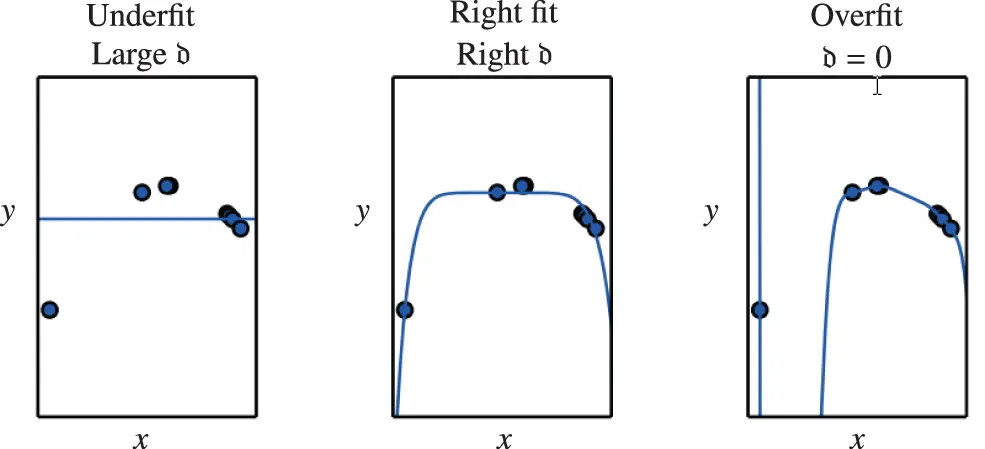Figure 67: Weight decay (Section 6.3.6). Effects of magnitude of weight-decay parameter 𝔡. Adapted from , p. 116. (Figure reproduced with permission of the authors.)

To have a general parameter-update equation that combines all of the above add-on improvement tricks, start with the parameter update with momentum and accelerated gradient Eq. (141)

θ˜k+1= θ˜kϵk g˜(θ˜k+γk(θ˜k θ˜k1))+ζk(θ˜k θ˜k1),

and add the weight-decay term 𝔡θ~k from Eq. (181), then scale both the weight-decay term and the gradient-descent term (ϵkg~k) by the cyclic annealing multiplier 𝔞k in Eq. (182), leaving the momentum term ζk(θ~kθ~k1) alone, to obtain:

θ~k+1=θ~kak[ϵkg~(θ~k+γk(θ~kθ~k1))+dkθ~k]+ζk(θ~kθ~k1),(183)

which is included in Algorithm 4.

6.4 Kaiming He initialization

All optimization algorithms discussed above share a crucial step, which crucially affects the convergence of training, especially as neural networks become ‘deep’: The initialization of the network’s parameters θ˜0 is not only related to the speed of convergence, it “can determine whether the algorithm converges at all.”172 Convergence problems are typically related to the scaling of initial parameters. Large parameters result in large activations, which leads to exploding values during forward and backward propagation, i.e., evaluation of the loss function and computation of its gradient with respect to the parameters. Small parameters, on the other hand, may result in vanishing gradients, i.e., the loss function becomes insensitive to parameters, which causes the training process to stall. To be precise, considerations regarding initialization are related to weight-matrices W(), see Section 4.4 on “Network layer, detailed construct”; bias vectors b() are usually initialized to zero, which is also assumed in the subsequent considerations.173

The Kaiming He initialization provides equally effective as simple means to overcome scaling issues observed when weights are randomly initialized using a normal distribution with fixed standard deviation. The key idea of the authors  is to have the same variance of weights for each of the network’s layers. As opposed to the Xavier initialization174, the nonlinearity of activation functions is accounted for. Consider the l-th layer of a feedforward neural network, where the vector z() follows as affine function of inputs y(1)

z()=W()y(1)+b(),y()=a(z()),(184)

where W() and b() denote the layer’s weight matrix and bias vector, respectively. The output of the layer y() is given by element-wise application of the activation function a, see Sections 4.4.1 and 4.4.2 for a detailed presentation. All components of the weight matrix W() are assumed to be independent of each other and to share the same probability distribution. The same holds for the components of the input vector y(1) and the output vector y(). Additionally, elements of W() and y() shall be mutually independent. Further, it is assumed that W() and y() have zero mean (i.e., expectation, cf. Eq. (67)) and are symmetric around zero. In this case, the variance of z()Rm()×1 is given by

Varzi()=m()Var(Wij()yj(1))(185)

=m()(VarWij()Varyj(1)+E(Wij())2Varyj(1)+VarWij()E(yj(1))2)(186)

=m()VarWij()(Varyj(1)+E(yj(1))2),(187)

where m() denotes the width of the -th layer and the fundamental relation Var(XY)=VarXVarY+E(X)2VarY+E(Y)2VarX has been used along with the assumption of weights having a zero mean, i.e., 𝔼(Wij())=0. The variance of some random variable X, which is the expectation of the squared deviation of X from its mean, i.e.,

VarX=E((XE(X))2),(188)

is a measure of the “dispersion” of X around its mean value. The variance is the square of the standard deviation σ of the random variable X, or, conversely,

σ(X)=VarX=E((XE(X))2.(189)

As opposed to the variance, the standard deviation of some random variable X has the same physical dimension as X itself.

The elementary relation VarX2=E(X2)E(X)2 gives

Varzi()=m()VarWij()E((yj(1))2),(190)

Note that the mean of inputs does not vanish for activation functions that are not symmetric about zero as, e.g., the ReLU functions (see Section 5.3.2). For the ReLU activation function, y=a(x)=max(0,x) the mean value of the squared output and the variance of the input are related by

E(y2)=y2P(y)dy(191)

=max(0,x)2P(x)dx=0x2P(x)dx=12x2P(x)dx(192)

=12Varx.(193)

Substituting the above result in Eq. (190) provides the following relationship among the variances of the inputs to the activation function of two consecutive layers, i.e., Varz() and Varz(1), respectively:

Varzi()=m()2VarWij()Varzj(1).(194)

For a network with L layers, the following relation between the variance of inputs Varz(1) and outputs Varz(L) is obtained:

zi(L)=Varzj(1)=2Lm()2VarWij().(195)

To preserve the variance through all layers of the network, the following condition must be fulfilled regarding the variance of weight matrices:

m()2VarWij()=1